path

sankey

# pyplot¶

## matplotlib.pyplot¶

Provides a MATLAB-like plotting framework.

pylab combines pyplot with numpy into a single namespace. This is convenient for interactive work, but for programming it is recommended that the namespaces be kept separate, e.g.:

```import numpy as np
import matplotlib.pyplot as plt

x = np.arange(0, 5, 0.1);
y = np.sin(x)
plt.plot(x, y)
```
matplotlib.pyplot.acorr(x, hold=None, **kwargs)

Plot the autocorrelation of x.

Call signature:

```acorr(x, normed=True, detrend=mlab.detrend_none, usevlines=True,
maxlags=10, **kwargs)
```

If normed = True, normalize the data by the autocorrelation at 0-th lag. x is detrended by the detrend callable (default no normalization).

Data are plotted as plot(lags, c, **kwargs)

Return value is a tuple (lags, c, line) where:

• lags are a length 2*maxlags+1 lag vector
• c is the 2*maxlags+1 auto correlation vector
• line is a Line2D instance returned by plot()

The default linestyle is None and the default marker is 'o', though these can be overridden with keyword args. The cross correlation is performed with numpy.correlate() with mode = 2.

If usevlines is True, vlines() rather than plot() is used to draw vertical lines from the origin to the acorr. Otherwise, the plot style is determined by the kwargs, which are Line2D properties.

maxlags is a positive integer detailing the number of lags to show. The default value of None will return all (2*len(x)-1) lags.

The return value is a tuple (lags, c, linecol, b) where

plot() or vlines() For documentation on valid kwargs.

Example:

xcorr() is top graph, and acorr() is bottom graph.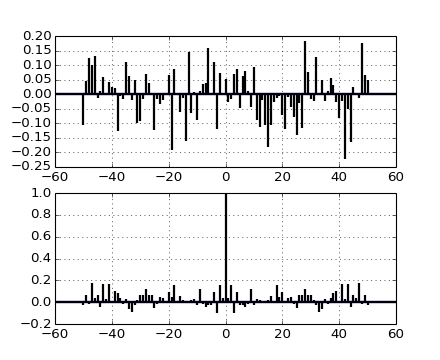Additional kwargs: hold = [True|False] overrides default hold state

matplotlib.pyplot.annotate(*args, **kwargs)

Create an annotation: a piece of text referring to a data point.

Call signature:

```annotate(s, xy, xytext=None, xycoords='data',
textcoords='data', arrowprops=None, **kwargs)
```

Keyword arguments:

Annotate the x, y point xy with text s at x, y location xytext. (If xytext = None, defaults to xy, and if textcoords = None, defaults to xycoords).

arrowprops, if not None, is a dictionary of line properties (see matplotlib.lines.Line2D) for the arrow that connects annotation to the point.

If the dictionary has a key arrowstyle, a FancyArrowPatch instance is created with the given dictionary and is drawn. Otherwise, a YAArow patch instance is created and drawn. Valid keys for YAArow are

Key Description
width the width of the arrow in points
frac the fraction of the arrow length occupied by the head
headwidth the width of the base of the arrow head in points
shrink oftentimes it is convenient to have the arrowtip and base a bit away from the text and point being annotated. If d is the distance between the text and annotated point, shrink will shorten the arrow so the tip and base are shink percent of the distance d away from the endpoints. ie, shrink=0.05 is 5%
? any key for matplotlib.patches.polygon

Valid keys for FancyArrowPatch are

Key Description
arrowstyle the arrow style
connectionstyle the connection style
relpos default is (0.5, 0.5)
patchA default is bounding box of the text
patchB default is None
shrinkA default is 2 points
shrinkB default is 2 points
mutation_scale default is text size (in points)
mutation_aspect default is 1.
? any key for matplotlib.patches.PathPatch

xycoords and textcoords are strings that indicate the coordinates of xy and xytext.

Property Description
‘figure points’ points from the lower left corner of the figure
‘figure pixels’ pixels from the lower left corner of the figure
‘figure fraction’ 0,0 is lower left of figure and 1,1 is upper right
‘axes points’ points from lower left corner of axes
‘axes pixels’ pixels from lower left corner of axes
‘axes fraction’ 0,0 is lower left of axes and 1,1 is upper right
‘data’ use the coordinate system of the object being annotated (default)
‘offset points’ Specify an offset (in points) from the xy value
‘polar’ you can specify theta, r for the annotation, even in cartesian plots. Note that if you are using a polar axes, you do not need to specify polar for the coordinate system since that is the native “data” coordinate system.

If a ‘points’ or ‘pixels’ option is specified, values will be added to the bottom-left and if negative, values will be subtracted from the top-right. e.g.:

```# 10 points to the right of the left border of the axes and
# 5 points below the top border
xy=(10,-5), xycoords='axes points'
```

You may use an instance of Transform or Artist. See Annotating Axes for more details.

The annotation_clip attribute contols the visibility of the annotation when it goes outside the axes area. If True, the annotation will only be drawn when the xy is inside the axes. If False, the annotation will always be drawn regardless of its position. The default is None, which behave as True only if xycoords is”data”.

Property Description
agg_filter unknown
alpha float (0.0 transparent through 1.0 opaque)
animated [True | False]
axes an Axes instance
backgroundcolor any matplotlib color
bbox rectangle prop dict
clip_box a matplotlib.transforms.Bbox instance
clip_on [True | False]
clip_path [ (Path, Transform) | Patch | None ]
color any matplotlib color
contains a callable function
family or fontfamily or fontname or name [FONTNAME | ‘serif’ | ‘sans-serif’ | ‘cursive’ | ‘fantasy’ | ‘monospace’ ]
figure a matplotlib.figure.Figure instance
fontproperties or font_properties a matplotlib.font_manager.FontProperties instance
gid an id string
horizontalalignment or ha [ ‘center’ | ‘right’ | ‘left’ ]
label string or anything printable with ‘%s’ conversion.
linespacing float (multiple of font size)
lod [True | False]
multialignment [‘left’ | ‘right’ | ‘center’ ]
path_effects unknown
picker [None|float|boolean|callable]
position (x,y)
rasterized [True | False | None]
rotation [ angle in degrees | ‘vertical’ | ‘horizontal’ ]
rotation_mode unknown
size or fontsize [size in points | ‘xx-small’ | ‘x-small’ | ‘small’ | ‘medium’ | ‘large’ | ‘x-large’ | ‘xx-large’ ]
sketch_params unknown
snap unknown
stretch or fontstretch [a numeric value in range 0-1000 | ‘ultra-condensed’ | ‘extra-condensed’ | ‘condensed’ | ‘semi-condensed’ | ‘normal’ | ‘semi-expanded’ | ‘expanded’ | ‘extra-expanded’ | ‘ultra-expanded’ ]
style or fontstyle [ ‘normal’ | ‘italic’ | ‘oblique’]
text string or anything printable with ‘%s’ conversion.
transform Transform instance
url a url string
variant or fontvariant [ ‘normal’ | ‘small-caps’ ]
verticalalignment or va or ma [ ‘center’ | ‘top’ | ‘bottom’ | ‘baseline’ ]
visible [True | False]
weight or fontweight [a numeric value in range 0-1000 | ‘ultralight’ | ‘light’ | ‘normal’ | ‘regular’ | ‘book’ | ‘medium’ | ‘roman’ | ‘semibold’ | ‘demibold’ | ‘demi’ | ‘bold’ | ‘heavy’ | ‘extra bold’ | ‘black’ ]
x float
y float
zorder any number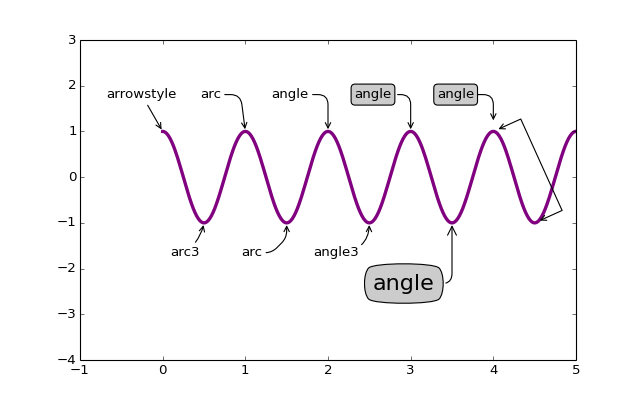(png, hires.png, pdf)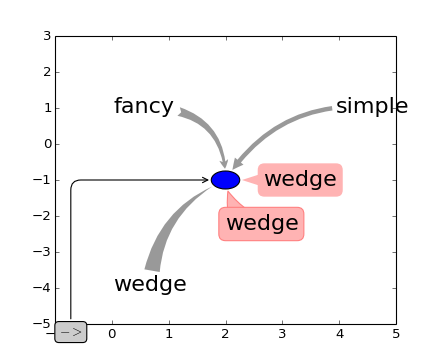(png, hires.png, pdf)

matplotlib.pyplot.arrow(x, y, dx, dy, hold=None, **kwargs)

Add an arrow to the axes.

Call signature:

```arrow(x, y, dx, dy, **kwargs)
```

Draws arrow on specified axis from (x, y) to (x + dx, y + dy). Uses FancyArrow patch to construct the arrow.

The resulting arrow is affected by the axes aspect ratio and limits. This may produce an arrow whose head is not square with its stem. To create an arrow whose head is square with its stem, use annotate().

Optional kwargs control the arrow construction and properties:

Constructor arguments
width: float (default: 0.001)
width of full arrow tail
length_includes_head: [True | False] (default: False)
True if head is to be counted in calculating the length.
head_width: float or None (default: 3*width)
total width of the full arrow head
shape: [‘full’, ‘left’, ‘right’] (default: ‘full’)
draw the left-half, right-half, or full arrow
overhang: float (default: 0)
fraction that the arrow is swept back (0 overhang means triangular shape). Can be negative or greater than one.
head_starts_at_zero: [True | False] (default: False)
if True, the head starts being drawn at coordinate 0 instead of ending at coordinate 0.

Other valid kwargs (inherited from Patch) are:

Property Description
agg_filter unknown
alpha float or None
animated [True | False]
antialiased or aa [True | False] or None for default
axes an Axes instance
clip_box a matplotlib.transforms.Bbox instance
clip_on [True | False]
clip_path [ (Path, Transform) | Patch | None ]
color matplotlib color spec
contains a callable function
edgecolor or ec mpl color spec, or None for default, or ‘none’ for no color
facecolor or fc mpl color spec, or None for default, or ‘none’ for no color
figure a matplotlib.figure.Figure instance
fill [True | False]
gid an id string
hatch [‘/’ | ‘\’ | ‘|’ | ‘-‘ | ‘+’ | ‘x’ | ‘o’ | ‘O’ | ‘.’ | ‘*’]
label string or anything printable with ‘%s’ conversion.
linestyle or ls [‘solid’ | ‘dashed’ | ‘dashdot’ | ‘dotted’]
linewidth or lw float or None for default
lod [True | False]
path_effects unknown
picker [None|float|boolean|callable]
rasterized [True | False | None]
sketch_params unknown
snap unknown
transform Transform instance
url a url string
visible [True | False]
zorder any number

Example: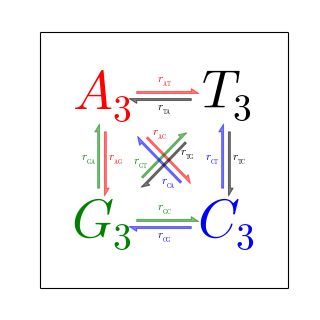Additional kwargs: hold = [True|False] overrides default hold state

matplotlib.pyplot.autoscale(enable=True, axis='both', tight=None)

Autoscale the axis view to the data (toggle).

Convenience method for simple axis view autoscaling. It turns autoscaling on or off, and then, if autoscaling for either axis is on, it performs the autoscaling on the specified axis or axes.

enable: [True | False | None]
True (default) turns autoscaling on, False turns it off. None leaves the autoscaling state unchanged.
axis: [‘x’ | ‘y’ | ‘both’]
which axis to operate on; default is ‘both’
tight: [True | False | None]
If True, set view limits to data limits; if False, let the locator and margins expand the view limits; if None, use tight scaling if the only artist is an image, otherwise treat tight as False. The tight setting is retained for future autoscaling until it is explicitly changed.

Returns None.

matplotlib.pyplot.autumn()

set the default colormap to autumn and apply to current image if any. See help(colormaps) for more information

matplotlib.pyplot.axes(*args, **kwargs)

Add an axes to the figure.

The axes is added at position rect specified by:

• axes() by itself creates a default full subplot(111) window axis.
• axes(rect, axisbg='w') where rect = [left, bottom, width, height] in normalized (0, 1) units. axisbg is the background color for the axis, default white.
• axes(h) where h is an axes instance makes h the current axis. An Axes instance is returned.
kwarg Accepts Description
axisbg color the axes background color
frameon [True|False] display the frame?
sharex otherax current axes shares xaxis attribute with otherax
sharey otherax current axes shares yaxis attribute with otherax
polar [True|False] use a polar axes?

Examples:

• examples/pylab_examples/axes_demo.py places custom axes.
• examples/pylab_examples/shared_axis_demo.py uses sharex and sharey.
matplotlib.pyplot.axhline(y=0, xmin=0, xmax=1, hold=None, **kwargs)

Add a horizontal line across the axis.

Call signature:

```axhline(y=0, xmin=0, xmax=1, **kwargs)
```

Draw a horizontal line at y from xmin to xmax. With the default values of xmin = 0 and xmax = 1, this line will always span the horizontal extent of the axes, regardless of the xlim settings, even if you change them, e.g., with the set_xlim() command. That is, the horizontal extent is in axes coords: 0=left, 0.5=middle, 1.0=right but the y location is in data coordinates.

Return value is the Line2D instance. kwargs are the same as kwargs to plot, and can be used to control the line properties. e.g.,

• draw a thick red hline at y = 0 that spans the xrange:

```>>> axhline(linewidth=4, color='r')
```
• draw a default hline at y = 1 that spans the xrange:

```>>> axhline(y=1)
```
• draw a default hline at y = .5 that spans the the middle half of the xrange:

```>>> axhline(y=.5, xmin=0.25, xmax=0.75)
```

Valid kwargs are Line2D properties, with the exception of ‘transform’:

Property Description
agg_filter unknown
alpha float (0.0 transparent through 1.0 opaque)
animated [True | False]
antialiased or aa [True | False]
axes an Axes instance
clip_box a matplotlib.transforms.Bbox instance
clip_on [True | False]
clip_path [ (Path, Transform) | Patch | None ]
color or c any matplotlib color
contains a callable function
dash_capstyle [‘butt’ | ‘round’ | ‘projecting’]
dash_joinstyle [‘miter’ | ‘round’ | ‘bevel’]
dashes sequence of on/off ink in points
drawstyle [‘default’ | ‘steps’ | ‘steps-pre’ | ‘steps-mid’ | ‘steps-post’]
figure a matplotlib.figure.Figure instance
fillstyle [‘full’ | ‘left’ | ‘right’ | ‘bottom’ | ‘top’ | ‘none’]
gid an id string
label string or anything printable with ‘%s’ conversion.
linestyle or ls ['-' | '--' | '-.' | ':' | 'None' | ' ' | ''] and any drawstyle in combination with a linestyle, e.g., 'steps--'.
linewidth or lw float value in points
lod [True | False]
marker unknown
markeredgecolor or mec any matplotlib color
markeredgewidth or mew float value in points
markerfacecolor or mfc any matplotlib color
markerfacecoloralt or mfcalt any matplotlib color
markersize or ms float
markevery None | integer | (startind, stride)
path_effects unknown
picker float distance in points or callable pick function fn(artist, event)
rasterized [True | False | None]
sketch_params unknown
snap unknown
solid_capstyle [‘butt’ | ‘round’ | ‘projecting’]
solid_joinstyle [‘miter’ | ‘round’ | ‘bevel’]
transform a matplotlib.transforms.Transform instance
url a url string
visible [True | False]
xdata 1D array
ydata 1D array
zorder any number

axhspan()
for example plot and source code

Additional kwargs: hold = [True|False] overrides default hold state

matplotlib.pyplot.axhspan(ymin, ymax, xmin=0, xmax=1, hold=None, **kwargs)

Add a horizontal span (rectangle) across the axis.

Call signature:

```axhspan(ymin, ymax, xmin=0, xmax=1, **kwargs)
```

y coords are in data units and x coords are in axes (relative 0-1) units.

Draw a horizontal span (rectangle) from ymin to ymax. With the default values of xmin = 0 and xmax = 1, this always spans the xrange, regardless of the xlim settings, even if you change them, e.g., with the set_xlim() command. That is, the horizontal extent is in axes coords: 0=left, 0.5=middle, 1.0=right but the y location is in data coordinates.

Return value is a matplotlib.patches.Polygon instance.

Examples:

• draw a gray rectangle from y = 0.25-0.75 that spans the horizontal extent of the axes:

```>>> axhspan(0.25, 0.75, facecolor='0.5', alpha=0.5)
```

Valid kwargs are Polygon properties:

Property Description
agg_filter unknown
alpha float or None
animated [True | False]
antialiased or aa [True | False] or None for default
axes an Axes instance
clip_box a matplotlib.transforms.Bbox instance
clip_on [True | False]
clip_path [ (Path, Transform) | Patch | None ]
color matplotlib color spec
contains a callable function
edgecolor or ec mpl color spec, or None for default, or ‘none’ for no color
facecolor or fc mpl color spec, or None for default, or ‘none’ for no color
figure a matplotlib.figure.Figure instance
fill [True | False]
gid an id string
hatch [‘/’ | ‘\’ | ‘|’ | ‘-‘ | ‘+’ | ‘x’ | ‘o’ | ‘O’ | ‘.’ | ‘*’]
label string or anything printable with ‘%s’ conversion.
linestyle or ls [‘solid’ | ‘dashed’ | ‘dashdot’ | ‘dotted’]
linewidth or lw float or None for default
lod [True | False]
path_effects unknown
picker [None|float|boolean|callable]
rasterized [True | False | None]
sketch_params unknown
snap unknown
transform Transform instance
url a url string
visible [True | False]
zorder any number

Example: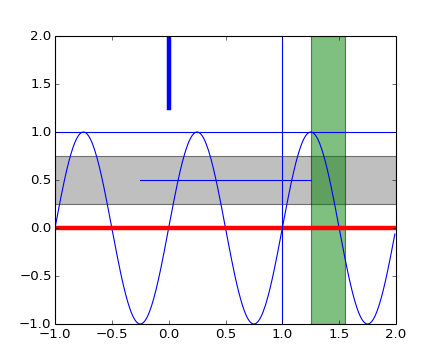Additional kwargs: hold = [True|False] overrides default hold state

matplotlib.pyplot.axis(*v, **kwargs)

Convenience method to get or set axis properties.

Calling with no arguments:

```>>> axis()
```

returns the current axes limits [xmin, xmax, ymin, ymax].:

```>>> axis(v)
```

sets the min and max of the x and y axes, with v = [xmin, xmax, ymin, ymax].:

```>>> axis('off')
```

turns off the axis lines and labels.:

```>>> axis('equal')
```

changes limits of x or y axis so that equal increments of x and y have the same length; a circle is circular.:

```>>> axis('scaled')
```

achieves the same result by changing the dimensions of the plot box instead of the axis data limits.:

```>>> axis('tight')
```

changes x and y axis limits such that all data is shown. If all data is already shown, it will move it to the center of the figure without modifying (xmax - xmin) or (ymax - ymin). Note this is slightly different than in MATLAB.:

```>>> axis('image')
```

is ‘scaled’ with the axis limits equal to the data limits.:

```>>> axis('auto')
```

and:

```>>> axis('normal')
```

are deprecated. They restore default behavior; axis limits are automatically scaled to make the data fit comfortably within the plot box.

if len(*v)==0, you can pass in xmin, xmax, ymin, ymax as kwargs selectively to alter just those limits without changing the others.

The xmin, xmax, ymin, ymax tuple is returned

xlim(), ylim()
For setting the x- and y-limits individually.
matplotlib.pyplot.axvline(x=0, ymin=0, ymax=1, hold=None, **kwargs)

Add a vertical line across the axes.

Call signature:

```axvline(x=0, ymin=0, ymax=1, **kwargs)
```

Draw a vertical line at x from ymin to ymax. With the default values of ymin = 0 and ymax = 1, this line will always span the vertical extent of the axes, regardless of the ylim settings, even if you change them, e.g., with the set_ylim() command. That is, the vertical extent is in axes coords: 0=bottom, 0.5=middle, 1.0=top but the x location is in data coordinates.

Return value is the Line2D instance. kwargs are the same as kwargs to plot, and can be used to control the line properties. e.g.,

• draw a thick red vline at x = 0 that spans the yrange:

```>>> axvline(linewidth=4, color='r')
```
• draw a default vline at x = 1 that spans the yrange:

```>>> axvline(x=1)
```
• draw a default vline at x = .5 that spans the the middle half of the yrange:

```>>> axvline(x=.5, ymin=0.25, ymax=0.75)
```

Valid kwargs are Line2D properties, with the exception of ‘transform’:

Property Description
agg_filter unknown
alpha float (0.0 transparent through 1.0 opaque)
animated [True | False]
antialiased or aa [True | False]
axes an Axes instance
clip_box a matplotlib.transforms.Bbox instance
clip_on [True | False]
clip_path [ (Path, Transform) | Patch | None ]
color or c any matplotlib color
contains a callable function
dash_capstyle [‘butt’ | ‘round’ | ‘projecting’]
dash_joinstyle [‘miter’ | ‘round’ | ‘bevel’]
dashes sequence of on/off ink in points
drawstyle [‘default’ | ‘steps’ | ‘steps-pre’ | ‘steps-mid’ | ‘steps-post’]
figure a matplotlib.figure.Figure instance
fillstyle [‘full’ | ‘left’ | ‘right’ | ‘bottom’ | ‘top’ | ‘none’]
gid an id string
label string or anything printable with ‘%s’ conversion.
linestyle or ls ['-' | '--' | '-.' | ':' | 'None' | ' ' | ''] and any drawstyle in combination with a linestyle, e.g., 'steps--'.
linewidth or lw float value in points
lod [True | False]
marker unknown
markeredgecolor or mec any matplotlib color
markeredgewidth or mew float value in points
markerfacecolor or mfc any matplotlib color
markerfacecoloralt or mfcalt any matplotlib color
markersize or ms float
markevery None | integer | (startind, stride)
path_effects unknown
picker float distance in points or callable pick function fn(artist, event)
rasterized [True | False | None]
sketch_params unknown
snap unknown
solid_capstyle [‘butt’ | ‘round’ | ‘projecting’]
solid_joinstyle [‘miter’ | ‘round’ | ‘bevel’]
transform a matplotlib.transforms.Transform instance
url a url string
visible [True | False]
xdata 1D array
ydata 1D array
zorder any number

axhspan()
for example plot and source code

Additional kwargs: hold = [True|False] overrides default hold state

matplotlib.pyplot.axvspan(xmin, xmax, ymin=0, ymax=1, hold=None, **kwargs)

Add a vertical span (rectangle) across the axes.

Call signature:

```axvspan(xmin, xmax, ymin=0, ymax=1, **kwargs)
```

x coords are in data units and y coords are in axes (relative 0-1) units.

Draw a vertical span (rectangle) from xmin to xmax. With the default values of ymin = 0 and ymax = 1, this always spans the yrange, regardless of the ylim settings, even if you change them, e.g., with the set_ylim() command. That is, the vertical extent is in axes coords: 0=bottom, 0.5=middle, 1.0=top but the y location is in data coordinates.

Return value is the matplotlib.patches.Polygon instance.

Examples:

• draw a vertical green translucent rectangle from x=1.25 to 1.55 that spans the yrange of the axes:

```>>> axvspan(1.25, 1.55, facecolor='g', alpha=0.5)
```

Valid kwargs are Polygon properties:

Property Description
agg_filter unknown
alpha float or None
animated [True | False]
antialiased or aa [True | False] or None for default
axes an Axes instance
clip_box a matplotlib.transforms.Bbox instance
clip_on [True | False]
clip_path [ (Path, Transform) | Patch | None ]
color matplotlib color spec
contains a callable function
edgecolor or ec mpl color spec, or None for default, or ‘none’ for no color
facecolor or fc mpl color spec, or None for default, or ‘none’ for no color
figure a matplotlib.figure.Figure instance
fill [True | False]
gid an id string
hatch [‘/’ | ‘\’ | ‘|’ | ‘-‘ | ‘+’ | ‘x’ | ‘o’ | ‘O’ | ‘.’ | ‘*’]
label string or anything printable with ‘%s’ conversion.
linestyle or ls [‘solid’ | ‘dashed’ | ‘dashdot’ | ‘dotted’]
linewidth or lw float or None for default
lod [True | False]
path_effects unknown
picker [None|float|boolean|callable]
rasterized [True | False | None]
sketch_params unknown
snap unknown
transform Transform instance
url a url string
visible [True | False]
zorder any number

axhspan()
for example plot and source code

Additional kwargs: hold = [True|False] overrides default hold state

matplotlib.pyplot.bar(left, height, width=0.8, bottom=None, hold=None, **kwargs)

Make a bar plot.

Make a bar plot with rectangles bounded by:

left, left + width, bottom, bottom + height
(left, right, bottom and top edges)
Parameters: left : sequence of scalars the x coordinates of the left sides of the bars height : sequence of scalars the heights of the bars width : scalar or array-like, optional, default: 0.8 the width(s) of the bars bottom : scalar or array-like, optional, default: None the y coordinate(s) of the bars color : scalar or array-like, optional the colors of the bar faces edgecolor : scalar or array-like, optional the colors of the bar edges linewidth : scalar or array-like, optional, default: None width of bar edge(s). If None, use default linewidth; If 0, don’t draw edges. xerr : scalar or array-like, optional, default: None if not None, will be used to generate errorbar(s) on the bar chart yerr :scalar or array-like, optional, default: None : if not None, will be used to generate errorbar(s) on the bar chart ecolor : scalar or array-like, optional, default: None specifies the color of errorbar(s) capsize : integer, optional, default: 3 determines the length in points of the error bar caps error_kw : : dictionary of kwargs to be passed to errorbar method. ecolor and capsize may be specified here rather than as independent kwargs. align : [‘edge’ | ‘center’], optional, default: ‘edge’ If edge, aligns bars by their left edges (for vertical bars) and by their bottom edges (for horizontal bars). If center, interpret the left argument as the coordinates of the centers of the bars. orientation : ‘vertical’ | ‘horizontal’, optional, default: ‘vertical’ The orientation of the bars. log : boolean, optional, default: False If true, sets the axis to be log scale :class:`matplotlib.patches.Rectangle` instances. :

Notes

The optional arguments color, edgecolor, linewidth, xerr, and yerr can be either scalars or sequences of length equal to the number of bars. This enables you to use bar as the basis for stacked bar charts, or candlestick plots. Detail: xerr and yerr are passed directly to errorbar(), so they can also have shape 2xN for independent specification of lower and upper errors.

Other optional kwargs:

Property Description
agg_filter unknown
alpha float or None
animated [True | False]
antialiased or aa [True | False] or None for default
axes an Axes instance
clip_box a matplotlib.transforms.Bbox instance
clip_on [True | False]
clip_path [ (Path, Transform) | Patch | None ]
color matplotlib color spec
contains a callable function
edgecolor or ec mpl color spec, or None for default, or ‘none’ for no color
facecolor or fc mpl color spec, or None for default, or ‘none’ for no color
figure a matplotlib.figure.Figure instance
fill [True | False]
gid an id string
hatch [‘/’ | ‘\’ | ‘|’ | ‘-‘ | ‘+’ | ‘x’ | ‘o’ | ‘O’ | ‘.’ | ‘*’]
label string or anything printable with ‘%s’ conversion.
linestyle or ls [‘solid’ | ‘dashed’ | ‘dashdot’ | ‘dotted’]
linewidth or lw float or None for default
lod [True | False]
path_effects unknown
picker [None|float|boolean|callable]
rasterized [True | False | None]
sketch_params unknown
snap unknown
transform Transform instance
url a url string
visible [True | False]
zorder any number

Example: A stacked bar chart.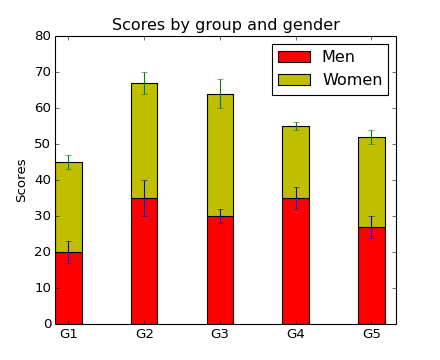Additional kwargs: hold = [True|False] overrides default hold state

matplotlib.pyplot.barbs(*args, **kw)

Plot a 2-D field of barbs.

Call signatures:

```barb(U, V, **kw)
barb(U, V, C, **kw)
barb(X, Y, U, V, **kw)
barb(X, Y, U, V, C, **kw)
```

Arguments:

X, Y:
The x and y coordinates of the barb locations (default is head of barb; see pivot kwarg)
U, V:
Give the x and y components of the barb shaft
C:
An optional array used to map colors to the barbs

All arguments may be 1-D or 2-D arrays or sequences. If X and Y are absent, they will be generated as a uniform grid. If U and V are 2-D arrays but X and Y are 1-D, and if len(X) and len(Y) match the column and row dimensions of U, then X and Y will be expanded with numpy.meshgrid().

U, V, C may be masked arrays, but masked X, Y are not supported at present.

Keyword arguments:

length:
Length of the barb in points; the other parts of the barb are scaled against this. Default is 9
pivot: [ ‘tip’ | ‘middle’ ]
The part of the arrow that is at the grid point; the arrow rotates about this point, hence the name pivot. Default is ‘tip’
barbcolor: [ color | color sequence ]
Specifies the color all parts of the barb except any flags. This parameter is analagous to the edgecolor parameter for polygons, which can be used instead. However this parameter will override facecolor.
flagcolor: [ color | color sequence ]
Specifies the color of any flags on the barb. This parameter is analagous to the facecolor parameter for polygons, which can be used instead. However this parameter will override facecolor. If this is not set (and C has not either) then flagcolor will be set to match barbcolor so that the barb has a uniform color. If C has been set, flagcolor has no effect.
sizes:

A dictionary of coefficients specifying the ratio of a given feature to the length of the barb. Only those values one wishes to override need to be included. These features include:

• ‘spacing’ - space between features (flags, full/half barbs)
• ‘height’ - height (distance from shaft to top) of a flag or full barb
• ‘width’ - width of a flag, twice the width of a full barb
• ‘emptybarb’ - radius of the circle used for low magnitudes
fill_empty:
A flag on whether the empty barbs (circles) that are drawn should be filled with the flag color. If they are not filled, they will be drawn such that no color is applied to the center. Default is False
rounding:
A flag to indicate whether the vector magnitude should be rounded when allocating barb components. If True, the magnitude is rounded to the nearest multiple of the half-barb increment. If False, the magnitude is simply truncated to the next lowest multiple. Default is True
barb_increments:

A dictionary of increments specifying values to associate with different parts of the barb. Only those values one wishes to override need to be included.

• ‘half’ - half barbs (Default is 5)
• ‘full’ - full barbs (Default is 10)
• ‘flag’ - flags (default is 50)
flip_barb:
Either a single boolean flag or an array of booleans. Single boolean indicates whether the lines and flags should point opposite to normal for all barbs. An array (which should be the same size as the other data arrays) indicates whether to flip for each individual barb. Normal behavior is for the barbs and lines to point right (comes from wind barbs having these features point towards low pressure in the Northern Hemisphere.) Default is False

Barbs are traditionally used in meteorology as a way to plot the speed and direction of wind observations, but can technically be used to plot any two dimensional vector quantity. As opposed to arrows, which give vector magnitude by the length of the arrow, the barbs give more quantitative information about the vector magnitude by putting slanted lines or a triangle for various increments in magnitude, as show schematically below:

```:     /\    \
:    /  \    \
:   /    \    \    \
:  /      \    \    \
: ------------------------------```

The largest increment is given by a triangle (or “flag”). After those come full lines (barbs). The smallest increment is a half line. There is only, of course, ever at most 1 half line. If the magnitude is small and only needs a single half-line and no full lines or triangles, the half-line is offset from the end of the barb so that it can be easily distinguished from barbs with a single full line. The magnitude for the barb shown above would nominally be 65, using the standard increments of 50, 10, and 5.

linewidths and edgecolors can be used to customize the barb. Additional PolyCollection keyword arguments:

Property Description
agg_filter unknown
alpha float or None
animated [True | False]
antialiased or antialiaseds Boolean or sequence of booleans
array unknown
axes an Axes instance
clim a length 2 sequence of floats
clip_box a matplotlib.transforms.Bbox instance
clip_on [True | False]
clip_path [ (Path, Transform) | Patch | None ]
cmap a colormap or registered colormap name
color matplotlib color arg or sequence of rgba tuples
contains a callable function
edgecolor or edgecolors matplotlib color arg or sequence of rgba tuples
facecolor or facecolors matplotlib color arg or sequence of rgba tuples
figure a matplotlib.figure.Figure instance
gid an id string
hatch [ ‘/’ | ‘\’ | ‘|’ | ‘-‘ | ‘+’ | ‘x’ | ‘o’ | ‘O’ | ‘.’ | ‘*’ ]
label string or anything printable with ‘%s’ conversion.
linestyle or linestyles or dashes [‘solid’ | ‘dashed’, ‘dashdot’, ‘dotted’ | (offset, on-off-dash-seq) ]
linewidth or lw or linewidths float or sequence of floats
lod [True | False]
norm unknown
offset_position unknown
offsets float or sequence of floats
path_effects unknown
picker [None|float|boolean|callable]
rasterized [True | False | None]
sketch_params unknown
snap unknown
transform Transform instance
url a url string
urls unknown
visible [True | False]
zorder any number

Example: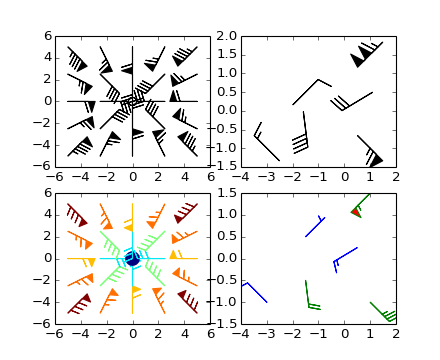(png, hires.png, pdf)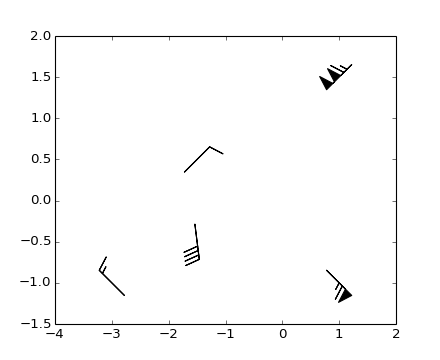(png, hires.png, pdf)

Additional kwargs: hold = [True|False] overrides default hold state

matplotlib.pyplot.barh(bottom, width, height=0.8, left=None, hold=None, **kwargs)

Make a horizontal bar plot.

Call signature:

```barh(bottom, width, height=0.8, left=0, **kwargs)
```

Make a horizontal bar plot with rectangles bounded by:

left, left + width, bottom, bottom + height
(left, right, bottom and top edges)

bottom, width, height, and left can be either scalars or sequences

Return value is a list of matplotlib.patches.Rectangle instances.

Required arguments:

Argument Description
bottom the vertical positions of the bottom edges of the bars
width the lengths of the bars

Optional keyword arguments:

Keyword Description
height the heights (thicknesses) of the bars
left the x coordinates of the left edges of the bars
color the colors of the bars
edgecolor the colors of the bar edges
linewidth width of bar edges; None means use default linewidth; 0 means don’t draw edges.
xerr if not None, will be used to generate errorbars on the bar chart
yerr if not None, will be used to generate errorbars on the bar chart
ecolor specifies the color of any errorbar
capsize (default 3) determines the length in points of the error bar caps
align ‘edge’ (default) | ‘center’
log [False|True] False (default) leaves the horizontal axis as-is; True sets it to log scale

Setting align = ‘edge’ aligns bars by their bottom edges in bottom, while align = ‘center’ interprets these values as the y coordinates of the bar centers.

The optional arguments color, edgecolor, linewidth, xerr, and yerr can be either scalars or sequences of length equal to the number of bars. This enables you to use barh as the basis for stacked bar charts, or candlestick plots.

other optional kwargs:

Property Description
agg_filter unknown
alpha float or None
animated [True | False]
antialiased or aa [True | False] or None for default
axes an Axes instance
clip_box a matplotlib.transforms.Bbox instance
clip_on [True | False]
clip_path [ (Path, Transform) | Patch | None ]
color matplotlib color spec
contains a callable function
edgecolor or ec mpl color spec, or None for default, or ‘none’ for no color
facecolor or fc mpl color spec, or None for default, or ‘none’ for no color
figure a matplotlib.figure.Figure instance
fill [True | False]
gid an id string
hatch [‘/’ | ‘\’ | ‘|’ | ‘-‘ | ‘+’ | ‘x’ | ‘o’ | ‘O’ | ‘.’ | ‘*’]
label string or anything printable with ‘%s’ conversion.
linestyle or ls [‘solid’ | ‘dashed’ | ‘dashdot’ | ‘dotted’]
linewidth or lw float or None for default
lod [True | False]
path_effects unknown
picker [None|float|boolean|callable]
rasterized [True | False | None]
sketch_params unknown
snap unknown
transform Transform instance
url a url string
visible [True | False]
zorder any number

Additional kwargs: hold = [True|False] overrides default hold state

matplotlib.pyplot.bone()

set the default colormap to bone and apply to current image if any. See help(colormaps) for more information

matplotlib.pyplot.box(on=None)

Turn the axes box on or off. on may be a boolean or a string, ‘on’ or ‘off’.

If on is None, toggle state.

matplotlib.pyplot.boxplot(x, notch=False, sym='b+', vert=True, whis=1.5, positions=None, widths=None, patch_artist=False, bootstrap=None, usermedians=None, conf_intervals=None, hold=None)

Make a box and whisker plot.

Call signature:

```boxplot(x, notch=False, sym='+', vert=True, whis=1.5,
positions=None, widths=None, patch_artist=False,
bootstrap=None, usermedians=None, conf_intervals=None)
```

Make a box and whisker plot for each column of x or each vector in sequence x. The box extends from the lower to upper quartile values of the data, with a line at the median. The whiskers extend from the box to show the range of the data. Flier points are those past the end of the whiskers.

Function Arguments:

x :
Array or a sequence of vectors.
notch : [ False (default) | True ]
If False (default), produces a rectangular box plot. If True, will produce a notched box plot
sym : [ default ‘b+’ ]
The default symbol for flier points. Enter an empty string (‘’) if you don’t want to show fliers.
vert : [ False | True (default) ]
If True (default), makes the boxes vertical. If False, makes horizontal boxes.
whis : [ default 1.5 ]
Defines the length of the whiskers as a function of the inner quartile range. They extend to the most extreme data point within ( whis*(75%-25%) ) data range.
bootstrap : [ None (default) | integer ]
Specifies whether to bootstrap the confidence intervals around the median for notched boxplots. If bootstrap==None, no bootstrapping is performed, and notches are calculated using a Gaussian-based asymptotic approximation (see McGill, R., Tukey, J.W., and Larsen, W.A., 1978, and Kendall and Stuart, 1967). Otherwise, bootstrap specifies the number of times to bootstrap the median to determine it’s 95% confidence intervals. Values between 1000 and 10000 are recommended.
usermedians : [ default None ]
An array or sequence whose first dimension (or length) is compatible with x. This overrides the medians computed by matplotlib for each element of usermedians that is not None. When an element of usermedians == None, the median will be computed directly as normal.
conf_intervals : [ default None ]
Array or sequence whose first dimension (or length) is compatible with x and whose second dimension is 2. When the current element of conf_intervals is not None, the notch locations computed by matplotlib are overridden (assuming notch is True). When an element of conf_intervals is None, boxplot compute notches the method specified by the other kwargs (e.g., bootstrap).
positions : [ default 1,2,...,n ]
Sets the horizontal positions of the boxes. The ticks and limits are automatically set to match the positions.
widths : [ default 0.5 ]
Either a scalar or a vector and sets the width of each box. The default is 0.5, or 0.15*(distance between extreme positions) if that is smaller.
patch_artist : [ False (default) | True ]
If False produces boxes with the Line2D artist If True produces boxes with the Patch artist

Returns a dictionary mapping each component of the boxplot to a list of the matplotlib.lines.Line2D instances created. That dictionary has the following keys (assuming vertical boxplots):

• boxes: the main body of the boxplot showing the quartiles and the median’s confidence intervals if enabled.
• medians: horizonal lines at the median of each box.
• whiskers: the vertical lines extending to the most extreme, n-outlier data points.
• caps: the horizontal lines at the ends of the whiskers.
• fliers: points representing data that extend beyone the whiskers (outliers).

Example: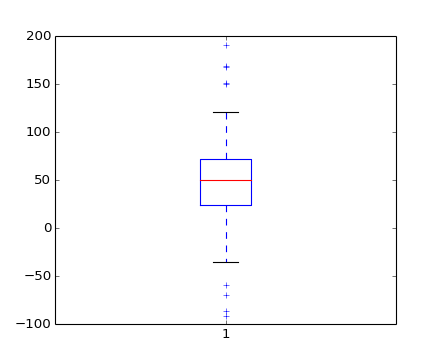(png, hires.png, pdf)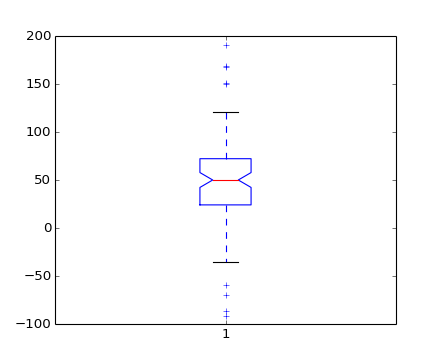(png, hires.png, pdf)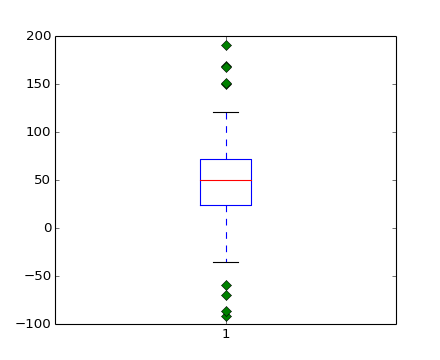(png, hires.png, pdf)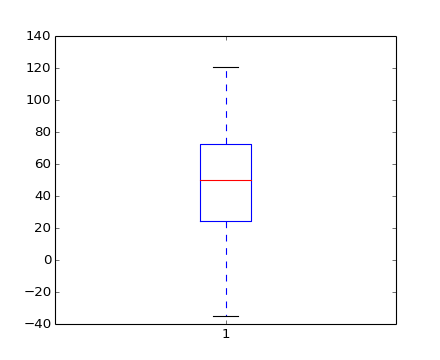(png, hires.png, pdf)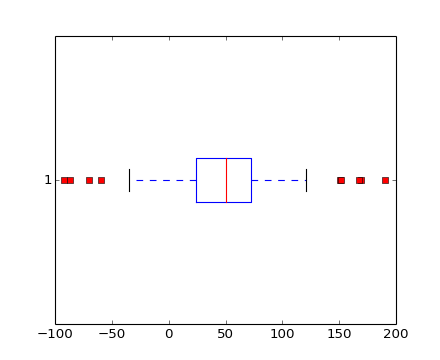(png, hires.png, pdf)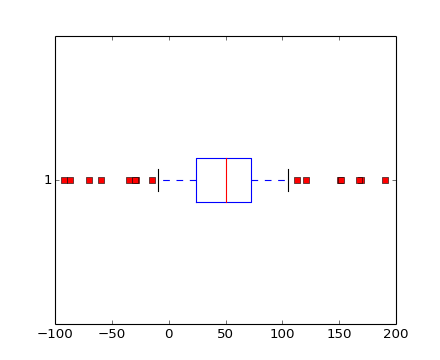(png, hires.png, pdf)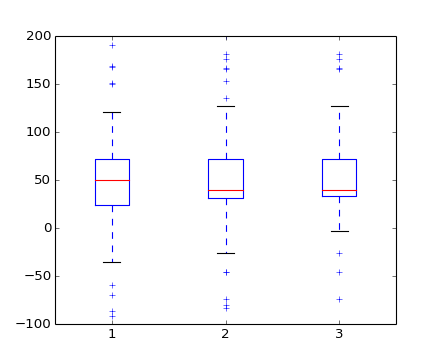(png, hires.png, pdf)

Additional kwargs: hold = [True|False] overrides default hold state

matplotlib.pyplot.broken_barh(xranges, yrange, hold=None, **kwargs)

Plot horizontal bars.

Call signature:

```broken_barh(self, xranges, yrange, **kwargs)
```

A collection of horizontal bars spanning yrange with a sequence of xranges.

Required arguments:

Argument Description
xranges sequence of (xmin, xwidth)
yrange sequence of (ymin, ywidth)

kwargs are matplotlib.collections.BrokenBarHCollection properties:

Property Description
agg_filter unknown
alpha float or None
animated [True | False]
antialiased or antialiaseds Boolean or sequence of booleans
array unknown
axes an Axes instance
clim a length 2 sequence of floats
clip_box a matplotlib.transforms.Bbox instance
clip_on [True | False]
clip_path [ (Path, Transform) | Patch | None ]
cmap a colormap or registered colormap name
color matplotlib color arg or sequence of rgba tuples
contains a callable function
edgecolor or edgecolors matplotlib color arg or sequence of rgba tuples
facecolor or facecolors matplotlib color arg or sequence of rgba tuples
figure a matplotlib.figure.Figure instance
gid an id string
hatch [ ‘/’ | ‘\’ | ‘|’ | ‘-‘ | ‘+’ | ‘x’ | ‘o’ | ‘O’ | ‘.’ | ‘*’ ]
label string or anything printable with ‘%s’ conversion.
linestyle or linestyles or dashes [‘solid’ | ‘dashed’, ‘dashdot’, ‘dotted’ | (offset, on-off-dash-seq) ]
linewidth or lw or linewidths float or sequence of floats
lod [True | False]
norm unknown
offset_position unknown
offsets float or sequence of floats
path_effects unknown
picker [None|float|boolean|callable]
rasterized [True | False | None]
sketch_params unknown
snap unknown
transform Transform instance
url a url string
urls unknown
visible [True | False]
zorder any number

these can either be a single argument, ie:

```facecolors = 'black'
```

or a sequence of arguments for the various bars, ie:

```facecolors = ('black', 'red', 'green')
```

Example: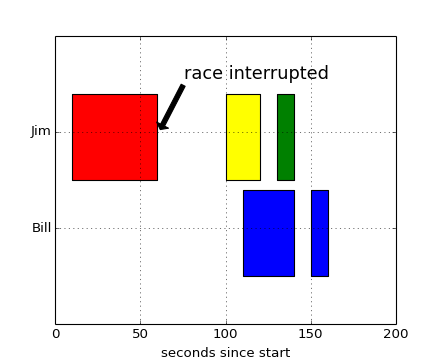Additional kwargs: hold = [True|False] overrides default hold state

matplotlib.pyplot.cla()

Clear the current axes.

matplotlib.pyplot.clabel(CS, *args, **kwargs)

Label a contour plot.

Call signature:

```clabel(cs, **kwargs)
```

Adds labels to line contours in cs, where cs is a ContourSet object returned by contour.

```clabel(cs, v, **kwargs)
```

only labels contours listed in v.

Optional keyword arguments:

fontsize:
size in points or relative size eg ‘smaller’, ‘x-large’
colors:
• if None, the color of each label matches the color of the corresponding contour
• if one string color, e.g., colors = ‘r’ or colors = ‘red’, all labels will be plotted in this color
• if a tuple of matplotlib color args (string, float, rgb, etc), different labels will be plotted in different colors in the order specified
inline:
controls whether the underlying contour is removed or not. Default is True.
inline_spacing:
space in pixels to leave on each side of label when placing inline. Defaults to 5. This spacing will be exact for labels at locations where the contour is straight, less so for labels on curved contours.
fmt:
a format string for the label. Default is ‘%1.3f’ Alternatively, this can be a dictionary matching contour levels with arbitrary strings to use for each contour level (i.e., fmt[level]=string), or it can be any callable, such as a Formatter instance, that returns a string when called with a numeric contour level.
manual:

if True, contour labels will be placed manually using mouse clicks. Click the first button near a contour to add a label, click the second button (or potentially both mouse buttons at once) to finish adding labels. The third button can be used to remove the last label added, but only if labels are not inline. Alternatively, the keyboard can be used to select label locations (enter to end label placement, delete or backspace act like the third mouse button, and any other key will select a label location).

manual can be an iterable object of x,y tuples. Contour labels will be created as if mouse is clicked at each x,y positions.

rightside_up:
if True (default), label rotations will always be plus or minus 90 degrees from level.
use_clabeltext:
if True (default is False), ClabelText class (instead of matplotlib.Text) is used to create labels. ClabelText recalculates rotation angles of texts during the drawing time, therefore this can be used if aspect of the axes changes.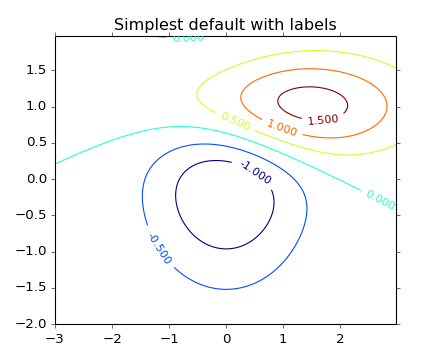(png, hires.png, pdf)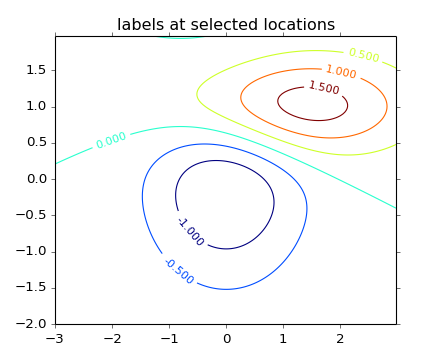(png, hires.png, pdf)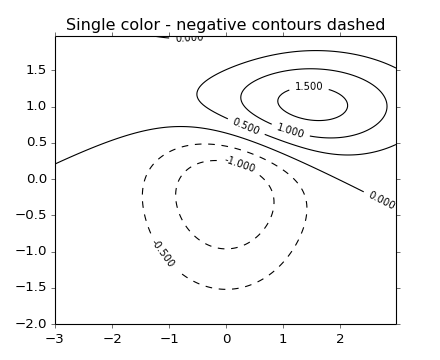(png, hires.png, pdf)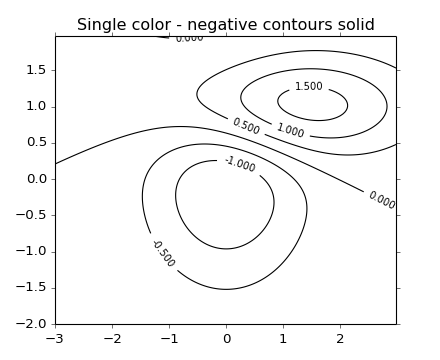(png, hires.png, pdf)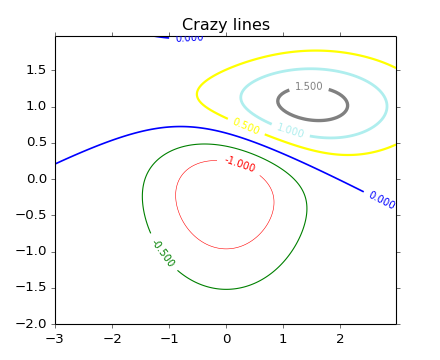(png, hires.png, pdf)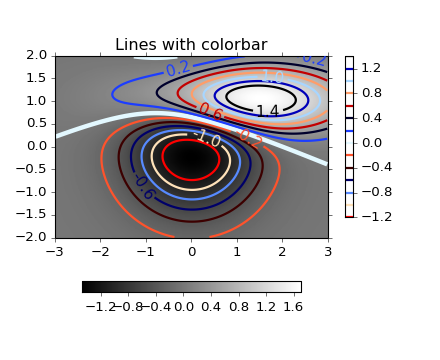(png, hires.png, pdf)

Additional kwargs: hold = [True|False] overrides default hold state

matplotlib.pyplot.clf()

Clear the current figure.

matplotlib.pyplot.clim(vmin=None, vmax=None)

Set the color limits of the current image.

To apply clim to all axes images do:

```clim(0, 0.5)
```

If either vmin or vmax is None, the image min/max respectively will be used for color scaling.

If you want to set the clim of multiple images, use, for example:

```for im in gca().get_images():
im.set_clim(0, 0.05)
```
matplotlib.pyplot.close(*args)

Close a figure window.

close() by itself closes the current figure

close(h) where h is a Figure instance, closes that figure

close(num) closes figure number num

close(name) where name is a string, closes figure with that label

close('all') closes all the figure windows

matplotlib.pyplot.cohere(x, y, NFFT=256, Fs=2, Fc=0, detrend=<function detrend_none at 0x2635de8>, window=<function window_hanning at 0x2635b90>, noverlap=0, pad_to=None, sides='default', scale_by_freq=None, hold=None, **kwargs)

Plot the coherence between x and y.

Call signature:

```cohere(x, y, NFFT=256, Fs=2, Fc=0, detrend = mlab.detrend_none,
sides='default', scale_by_freq=None, **kwargs)
```

Plot the coherence between x and y. Coherence is the normalized cross spectral density: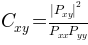Keyword arguments:

NFFT: integer
The number of data points used in each block for the FFT. Must be even; a power 2 is most efficient. The default value is 256. This should NOT be used to get zero padding, or the scaling of the result will be incorrect. Use pad_to for this instead.
Fs: scalar
The sampling frequency (samples per time unit). It is used to calculate the Fourier frequencies, freqs, in cycles per time unit. The default value is 2.
detrend: callable
The function applied to each segment before fft-ing, designed to remove the mean or linear trend. Unlike in MATLAB, where the detrend parameter is a vector, in matplotlib is it a function. The pylab module defines detrend_none(), detrend_mean(), and detrend_linear(), but you can use a custom function as well.
window: callable or ndarray
A function or a vector of length NFFT. To create window vectors see window_hanning(), window_none(), numpy.blackman(), numpy.hamming(), numpy.bartlett(), scipy.signal(), scipy.signal.get_window(), etc. The default is window_hanning(). If a function is passed as the argument, it must take a data segment as an argument and return the windowed version of the segment.
The number of points to which the data segment is padded when performing the FFT. This can be different from NFFT, which specifies the number of data points used. While not increasing the actual resolution of the psd (the minimum distance between resolvable peaks), this can give more points in the plot, allowing for more detail. This corresponds to the n parameter in the call to fft(). The default is None, which sets pad_to equal to NFFT
sides: [ ‘default’ | ‘onesided’ | ‘twosided’ ]
Specifies which sides of the PSD to return. Default gives the default behavior, which returns one-sided for real data and both for complex data. ‘onesided’ forces the return of a one-sided PSD, while ‘twosided’ forces two-sided.
scale_by_freq: boolean
Specifies whether the resulting density values should be scaled by the scaling frequency, which gives density in units of Hz^-1. This allows for integration over the returned frequency values. The default is True for MATLAB compatibility.
noverlap: integer
The number of points of overlap between blocks. The default value is 0 (no overlap).
Fc: integer
The center frequency of x (defaults to 0), which offsets the x extents of the plot to reflect the frequency range used when a signal is acquired and then filtered and downsampled to baseband.

The return value is a tuple (Cxy, f), where f are the frequencies of the coherence vector.

kwargs are applied to the lines.

References:

• Bendat & Piersol – Random Data: Analysis and Measurement Procedures, John Wiley & Sons (1986)

kwargs control the Line2D properties of the coherence plot:

Property Description
agg_filter unknown
alpha float (0.0 transparent through 1.0 opaque)
animated [True | False]
antialiased or aa [True | False]
axes an Axes instance
clip_box a matplotlib.transforms.Bbox instance
clip_on [True | False]
clip_path [ (Path, Transform) | Patch | None ]
color or c any matplotlib color
contains a callable function
dash_capstyle [‘butt’ | ‘round’ | ‘projecting’]
dash_joinstyle [‘miter’ | ‘round’ | ‘bevel’]
dashes sequence of on/off ink in points
drawstyle [‘default’ | ‘steps’ | ‘steps-pre’ | ‘steps-mid’ | ‘steps-post’]
figure a matplotlib.figure.Figure instance
fillstyle [‘full’ | ‘left’ | ‘right’ | ‘bottom’ | ‘top’ | ‘none’]
gid an id string
label string or anything printable with ‘%s’ conversion.
linestyle or ls ['-' | '--' | '-.' | ':' | 'None' | ' ' | ''] and any drawstyle in combination with a linestyle, e.g., 'steps--'.
linewidth or lw float value in points
lod [True | False]
marker unknown
markeredgecolor or mec any matplotlib color
markeredgewidth or mew float value in points
markerfacecolor or mfc any matplotlib color
markerfacecoloralt or mfcalt any matplotlib color
markersize or ms float
markevery None | integer | (startind, stride)
path_effects unknown
picker float distance in points or callable pick function fn(artist, event)
rasterized [True | False | None]
sketch_params unknown
snap unknown
solid_capstyle [‘butt’ | ‘round’ | ‘projecting’]
solid_joinstyle [‘miter’ | ‘round’ | ‘bevel’]
transform a matplotlib.transforms.Transform instance
url a url string
visible [True | False]
xdata 1D array
ydata 1D array
zorder any number

Example: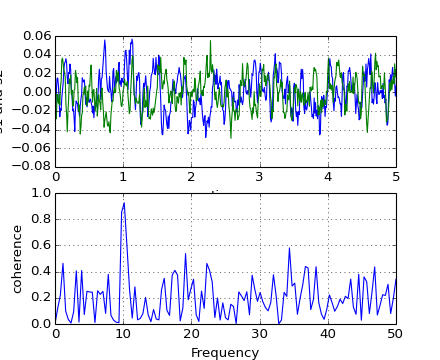Additional kwargs: hold = [True|False] overrides default hold state

matplotlib.pyplot.colorbar(mappable=None, cax=None, ax=None, **kw)

Add a colorbar to a plot.

Function signatures for the pyplot interface; all but the first are also method signatures for the colorbar() method:

```colorbar(**kwargs)
colorbar(mappable, **kwargs)
colorbar(mappable, cax=cax, **kwargs)
colorbar(mappable, ax=ax, **kwargs)
```

arguments:

mappable
the Image, ContourSet, etc. to which the colorbar applies; this argument is mandatory for the colorbar() method but optional for the colorbar() function, which sets the default to the current image.

keyword arguments:

cax
None | axes object into which the colorbar will be drawn
ax
None | parent axes object(s) from which space for a new colorbar axes will be stolen. If a list of axes is given they will all be resized to make room for the colorbar axes.
use_gridspec
False | If cax is None, a new cax is created as an instance of Axes. If ax is an instance of Subplot and use_gridspec is True, cax is created as an instance of Subplot using the grid_spec module.

Additional keyword arguments are of two kinds:

axes properties:

Property Description
orientation vertical or horizontal
fraction 0.15; fraction of original axes to use for colorbar
pad 0.05 if vertical, 0.15 if horizontal; fraction of original axes between colorbar and new image axes
shrink 1.0; fraction by which to shrink the colorbar
aspect 20; ratio of long to short dimensions
anchor (0.0, 0.5) if vertical; (0.5, 1.0) if horizontal; the anchor point of the colorbar axes
panchor (1.0, 0.5) if vertical; (0.5, 0.0) if horizontal; the anchor point of the colorbar parent axes. If False, the parent axes’ anchor will be unchanged

colorbar properties:

Property Description
extend [ ‘neither’ | ‘both’ | ‘min’ | ‘max’ ] If not ‘neither’, make pointed end(s) for out-of- range values. These are set for a given colormap using the colormap set_under and set_over methods.
extendfrac [ None | ‘auto’ | length | lengths ] If set to None, both the minimum and maximum triangular colorbar extensions with have a length of 5% of the interior colorbar length (this is the default setting). If set to ‘auto’, makes the triangular colorbar extensions the same lengths as the interior boxes (when spacing is set to ‘uniform’) or the same lengths as the respective adjacent interior boxes (when spacing is set to ‘proportional’). If a scalar, indicates the length of both the minimum and maximum triangular colorbar extensions as a fraction of the interior colorbar length. A two-element sequence of fractions may also be given, indicating the lengths of the minimum and maximum colorbar extensions respectively as a fraction of the interior colorbar length.
extendrect [ False | True ] If False the minimum and maximum colorbar extensions will be triangular (the default). If True the extensions will be rectangular.
spacing [ ‘uniform’ | ‘proportional’ ] Uniform spacing gives each discrete color the same space; proportional makes the space proportional to the data interval.
ticks [ None | list of ticks | Locator object ] If None, ticks are determined automatically from the input.
format [ None | format string | Formatter object ] If None, the ScalarFormatter is used. If a format string is given, e.g., ‘%.3f’, that is used. An alternative Formatter object may be given instead.
drawedges [ False | True ] If true, draw lines at color boundaries.

The following will probably be useful only in the context of indexed colors (that is, when the mappable has norm=NoNorm()), or other unusual circumstances.

Property Description
boundaries None or a sequence
values None or a sequence which must be of length 1 less than the sequence of boundaries. For each region delimited by adjacent entries in boundaries, the color mapped to the corresponding value in values will be used.

If mappable is a ContourSet, its extend kwarg is included automatically.

Note that the shrink kwarg provides a simple way to keep a vertical colorbar, for example, from being taller than the axes of the mappable to which the colorbar is attached; but it is a manual method requiring some trial and error. If the colorbar is too tall (or a horizontal colorbar is too wide) use a smaller value of shrink.

For more precise control, you can manually specify the positions of the axes objects in which the mappable and the colorbar are drawn. In this case, do not use any of the axes properties kwargs.

It is known that some vector graphics viewer (svg and pdf) renders white gaps between segments of the colorbar. This is due to bugs in the viewers not matplotlib. As a workaround the colorbar can be rendered with overlapping segments:

```cbar = colorbar()
cbar.solids.set_edgecolor("face")
draw()
```

However this has negative consequences in other circumstances. Particularly with semi transparent images (alpha < 1) and colorbar extensions and is not enabled by default see (issue #1188).

returns:
Colorbar instance; see also its base class, ColorbarBase. Call the set_label() method to label the colorbar.
matplotlib.pyplot.colors()

This is a do-nothing function to provide you with help on how matplotlib handles colors.

Commands which take color arguments can use several formats to specify the colors. For the basic built-in colors, you can use a single letter

Alias Color
‘b’ blue
‘g’ green
‘r’ red
‘c’ cyan
‘m’ magenta
‘y’ yellow
‘k’ black
‘w’ white

For a greater range of colors, you have two options. You can specify the color using an html hex string, as in:

```color = '#eeefff'
```

or you can pass an R,G,B tuple, where each of R,G,B are in the range [0,1].

You can also use any legal html name for a color, for example:

```color = 'red'
color = 'burlywood'
color = 'chartreuse'
```

The example below creates a subplot with a dark slate gray background:

```subplot(111, axisbg=(0.1843, 0.3098, 0.3098))
```

Here is an example that creates a pale turquoise title:

```title('Is this the best color?', color='#afeeee')
```
matplotlib.pyplot.connect(s, func)

Connect event with string s to func. The signature of func is:

`def func(event)`

where event is a matplotlib.backend_bases.Event. The following events are recognized

• ‘button_press_event’
• ‘button_release_event’
• ‘draw_event’
• ‘key_press_event’
• ‘key_release_event’
• ‘motion_notify_event’
• ‘pick_event’
• ‘resize_event’
• ‘scroll_event’
• ‘figure_enter_event’,
• ‘figure_leave_event’,
• ‘axes_enter_event’,
• ‘axes_leave_event’
• ‘close_event’

For the location events (button and key press/release), if the mouse is over the axes, the variable event.inaxes will be set to the Axes the event occurs is over, and additionally, the variables event.xdata and event.ydata will be defined. This is the mouse location in data coords. See KeyEvent and MouseEvent for more info.

Return value is a connection id that can be used with mpl_disconnect().

Example usage:

```def on_press(event):
print('you pressed', event.button, event.xdata, event.ydata)

cid = canvas.mpl_connect('button_press_event', on_press)
```
matplotlib.pyplot.contour(*args, **kwargs)

Plot contours.

contour() and contourf() draw contour lines and filled contours, respectively. Except as noted, function signatures and return values are the same for both versions.

contourf() differs from the MATLAB version in that it does not draw the polygon edges. To draw edges, add line contours with calls to contour().

Call signatures:

```contour(Z)
```

make a contour plot of an array Z. The level values are chosen automatically.

```contour(X,Y,Z)
```

X, Y specify the (x, y) coordinates of the surface

```contour(Z,N)
contour(X,Y,Z,N)
```

contour N automatically-chosen levels.

```contour(Z,V)
contour(X,Y,Z,V)
```

draw contour lines at the values specified in sequence V

```contourf(..., V)
```

fill the len(V)-1 regions between the values in V

```contour(Z, **kwargs)
```

Use keyword args to control colors, linewidth, origin, cmap ... see below for more details.

X and Y must both be 2-D with the same shape as Z, or they must both be 1-D such that len(X) is the number of columns in Z and len(Y) is the number of rows in Z.

C = contour(...) returns a QuadContourSet object.

Optional keyword arguments:

colors: [ None | string | (mpl_colors) ]

If None, the colormap specified by cmap will be used.

If a string, like ‘r’ or ‘red’, all levels will be plotted in this color.

If a tuple of matplotlib color args (string, float, rgb, etc), different levels will be plotted in different colors in the order specified.

alpha: float
The alpha blending value
cmap: [ None | Colormap ]
A cm Colormap instance or None. If cmap is None and colors is None, a default Colormap is used.
norm: [ None | Normalize ]
A matplotlib.colors.Normalize instance for scaling data values to colors. If norm is None and colors is None, the default linear scaling is used.
vmin, vmax: [ None | scalar ]
If not None, either or both of these values will be supplied to the matplotlib.colors.Normalize instance, overriding the default color scaling based on levels.
levels: [level0, level1, ..., leveln]
A list of floating point numbers indicating the level curves to draw; eg to draw just the zero contour pass levels=
origin: [ None | ‘upper’ | ‘lower’ | ‘image’ ]

If None, the first value of Z will correspond to the lower left corner, location (0,0). If ‘image’, the rc value for image.origin will be used.

This keyword is not active if X and Y are specified in the call to contour.

extent: [ None | (x0,x1,y0,y1) ]

If origin is not None, then extent is interpreted as in matplotlib.pyplot.imshow(): it gives the outer pixel boundaries. In this case, the position of Z[0,0] is the center of the pixel, not a corner. If origin is None, then (x0, y0) is the position of Z[0,0], and (x1, y1) is the position of Z[-1,-1].

This keyword is not active if X and Y are specified in the call to contour.

locator: [ None | ticker.Locator subclass ]
If locator is None, the default MaxNLocator is used. The locator is used to determine the contour levels if they are not given explicitly via the V argument.
extend: [ ‘neither’ | ‘both’ | ‘min’ | ‘max’ ]
Unless this is ‘neither’, contour levels are automatically added to one or both ends of the range so that all data are included. These added ranges are then mapped to the special colormap values which default to the ends of the colormap range, but can be set via matplotlib.colors.Colormap.set_under() and matplotlib.colors.Colormap.set_over() methods.
xunits, yunits: [ None | registered units ]
Override axis units by specifying an instance of a matplotlib.units.ConversionInterface.
antialiased: [ True | False ]
enable antialiasing, overriding the defaults. For filled contours, the default is True. For line contours, it is taken from rcParams[‘lines.antialiased’].

contour-only keyword arguments:

linewidths: [ None | number | tuple of numbers ]

If linewidths is None, the default width in lines.linewidth in matplotlibrc is used.

If a number, all levels will be plotted with this linewidth.

If a tuple, different levels will be plotted with different linewidths in the order specified

linestyles: [ None | ‘solid’ | ‘dashed’ | ‘dashdot’ | ‘dotted’ ]

If linestyles is None, the default is ‘solid’ unless the lines are monochrome. In that case, negative contours will take their linestyle from the matplotlibrc contour.negative_linestyle setting.

linestyles can also be an iterable of the above strings specifying a set of linestyles to be used. If this iterable is shorter than the number of contour levels it will be repeated as necessary.

contourf-only keyword arguments:

nchunk: [ 0 | integer ]
If 0, no subdivision of the domain. Specify a positive integer to divide the domain into subdomains of roughly nchunk by nchunk points. This may never actually be advantageous, so this option may be removed. Chunking introduces artifacts at the chunk boundaries unless antialiased is False.
hatches:
A list of cross hatch patterns to use on the filled areas. If None, no hatching will be added to the contour. Hatching is supported in the PostScript, PDF, SVG and Agg backends only.

Note: contourf fills intervals that are closed at the top; that is, for boundaries z1 and z2, the filled region is:

```z1 < z <= z2
```

There is one exception: if the lowest boundary coincides with the minimum value of the z array, then that minimum value will be included in the lowest interval.

Examples:(png, hires.png, pdf)(png, hires.png, pdf)(png, hires.png, pdf)(png, hires.png, pdf)(png, hires.png, pdf)(png, hires.png, pdf)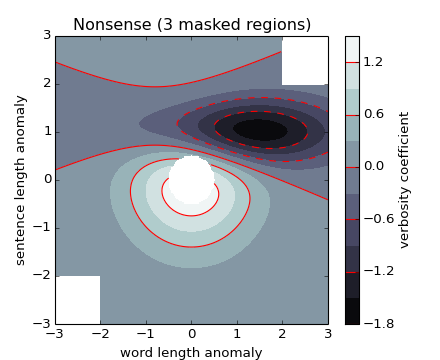(png, hires.png, pdf)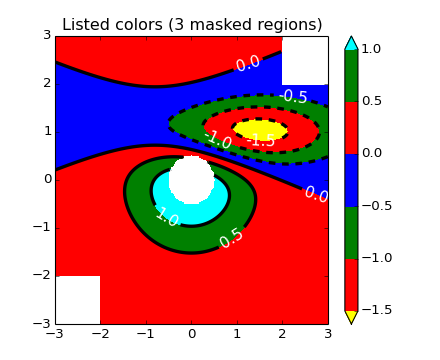(png, hires.png, pdf)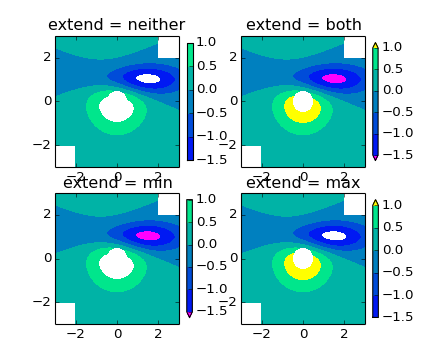(png, hires.png, pdf)

Additional kwargs: hold = [True|False] overrides default hold state

matplotlib.pyplot.contourf(*args, **kwargs)

Plot contours.

contour() and contourf() draw contour lines and filled contours, respectively. Except as noted, function signatures and return values are the same for both versions.

contourf() differs from the MATLAB version in that it does not draw the polygon edges. To draw edges, add line contours with calls to contour().

Call signatures:

```contour(Z)
```

make a contour plot of an array Z. The level values are chosen automatically.

```contour(X,Y,Z)
```

X, Y specify the (x, y) coordinates of the surface

```contour(Z,N)
contour(X,Y,Z,N)
```

contour N automatically-chosen levels.

```contour(Z,V)
contour(X,Y,Z,V)
```

draw contour lines at the values specified in sequence V

```contourf(..., V)
```

fill the len(V)-1 regions between the values in V

```contour(Z, **kwargs)
```

Use keyword args to control colors, linewidth, origin, cmap ... see below for more details.

X and Y must both be 2-D with the same shape as Z, or they must both be 1-D such that len(X) is the number of columns in Z and len(Y) is the number of rows in Z.

C = contour(...) returns a QuadContourSet object.

Optional keyword arguments:

colors: [ None | string | (mpl_colors) ]

If None, the colormap specified by cmap will be used.

If a string, like ‘r’ or ‘red’, all levels will be plotted in this color.

If a tuple of matplotlib color args (string, float, rgb, etc), different levels will be plotted in different colors in the order specified.

alpha: float
The alpha blending value
cmap: [ None | Colormap ]
A cm Colormap instance or None. If cmap is None and colors is None, a default Colormap is used.
norm: [ None | Normalize ]
A matplotlib.colors.Normalize instance for scaling data values to colors. If norm is None and colors is None, the default linear scaling is used.
vmin, vmax: [ None | scalar ]
If not None, either or both of these values will be supplied to the matplotlib.colors.Normalize instance, overriding the default color scaling based on levels.
levels: [level0, level1, ..., leveln]
A list of floating point numbers indicating the level curves to draw; eg to draw just the zero contour pass levels=
origin: [ None | ‘upper’ | ‘lower’ | ‘image’ ]

If None, the first value of Z will correspond to the lower left corner, location (0,0). If ‘image’, the rc value for image.origin will be used.

This keyword is not active if X and Y are specified in the call to contour.

extent: [ None | (x0,x1,y0,y1) ]

If origin is not None, then extent is interpreted as in matplotlib.pyplot.imshow(): it gives the outer pixel boundaries. In this case, the position of Z[0,0] is the center of the pixel, not a corner. If origin is None, then (x0, y0) is the position of Z[0,0], and (x1, y1) is the position of Z[-1,-1].

This keyword is not active if X and Y are specified in the call to contour.

locator: [ None | ticker.Locator subclass ]
If locator is None, the default MaxNLocator is used. The locator is used to determine the contour levels if they are not given explicitly via the V argument.
extend: [ ‘neither’ | ‘both’ | ‘min’ | ‘max’ ]
Unless this is ‘neither’, contour levels are automatically added to one or both ends of the range so that all data are included. These added ranges are then mapped to the special colormap values which default to the ends of the colormap range, but can be set via matplotlib.colors.Colormap.set_under() and matplotlib.colors.Colormap.set_over() methods.
xunits, yunits: [ None | registered units ]
Override axis units by specifying an instance of a matplotlib.units.ConversionInterface.
antialiased: [ True | False ]
enable antialiasing, overriding the defaults. For filled contours, the default is True. For line contours, it is taken from rcParams[‘lines.antialiased’].

contour-only keyword arguments:

linewidths: [ None | number | tuple of numbers ]

If linewidths is None, the default width in lines.linewidth in matplotlibrc is used.

If a number, all levels will be plotted with this linewidth.

If a tuple, different levels will be plotted with different linewidths in the order specified

linestyles: [ None | ‘solid’ | ‘dashed’ | ‘dashdot’ | ‘dotted’ ]

If linestyles is None, the default is ‘solid’ unless the lines are monochrome. In that case, negative contours will take their linestyle from the matplotlibrc contour.negative_linestyle setting.

linestyles can also be an iterable of the above strings specifying a set of linestyles to be used. If this iterable is shorter than the number of contour levels it will be repeated as necessary.

contourf-only keyword arguments:

nchunk: [ 0 | integer ]
If 0, no subdivision of the domain. Specify a positive integer to divide the domain into subdomains of roughly nchunk by nchunk points. This may never actually be advantageous, so this option may be removed. Chunking introduces artifacts at the chunk boundaries unless antialiased is False.
hatches:
A list of cross hatch patterns to use on the filled areas. If None, no hatching will be added to the contour. Hatching is supported in the PostScript, PDF, SVG and Agg backends only.

Note: contourf fills intervals that are closed at the top; that is, for boundaries z1 and z2, the filled region is:

```z1 < z <= z2
```

There is one exception: if the lowest boundary coincides with the minimum value of the z array, then that minimum value will be included in the lowest interval.

Examples:(png, hires.png, pdf)(png, hires.png, pdf)(png, hires.png, pdf)(png, hires.png, pdf)(png, hires.png, pdf)(png, hires.png, pdf)(png, hires.png, pdf)(png, hires.png, pdf)(png, hires.png, pdf)

Additional kwargs: hold = [True|False] overrides default hold state

matplotlib.pyplot.cool()

set the default colormap to cool and apply to current image if any. See help(colormaps) for more information

matplotlib.pyplot.copper()

set the default colormap to copper and apply to current image if any. See help(colormaps) for more information

matplotlib.pyplot.csd(x, y, NFFT=256, Fs=2, Fc=0, detrend=<function detrend_none at 0x2635de8>, window=<function window_hanning at 0x2635b90>, noverlap=0, pad_to=None, sides='default', scale_by_freq=None, hold=None, **kwargs)

Plot cross-spectral density.

Call signature:

```csd(x, y, NFFT=256, Fs=2, Fc=0, detrend=mlab.detrend_none,
sides='default', scale_by_freq=None, **kwargs)
```

The cross spectral density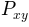by Welch’s average periodogram method. The vectors x and y are divided into NFFT length segments. Each segment is detrended by function detrend and windowed by function window. The product of the direct FFTs of x and y are averaged over each segment to compute, with a scaling to correct for power loss due to windowing.

Returns the tuple (Pxy, freqs). P is the cross spectrum (complex valued), and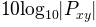is plotted.

Keyword arguments:

NFFT: integer
The number of data points used in each block for the FFT. Must be even; a power 2 is most efficient. The default value is 256. This should NOT be used to get zero padding, or the scaling of the result will be incorrect. Use pad_to for this instead.
Fs: scalar
The sampling frequency (samples per time unit). It is used to calculate the Fourier frequencies, freqs, in cycles per time unit. The default value is 2.
detrend: callable
The function applied to each segment before fft-ing, designed to remove the mean or linear trend. Unlike in MATLAB, where the detrend parameter is a vector, in matplotlib is it a function. The pylab module defines detrend_none(), detrend_mean(), and detrend_linear(), but you can use a custom function as well.
window: callable or ndarray
A function or a vector of length NFFT. To create window vectors see window_hanning(), window_none(), numpy.blackman(), numpy.hamming(), numpy.bartlett(), scipy.signal(), scipy.signal.get_window(), etc. The default is window_hanning(). If a function is passed as the argument, it must take a data segment as an argument and return the windowed version of the segment.
The number of points to which the data segment is padded when performing the FFT. This can be different from NFFT, which specifies the number of data points used. While not increasing the actual resolution of the psd (the minimum distance between resolvable peaks), this can give more points in the plot, allowing for more detail. This corresponds to the n parameter in the call to fft(). The default is None, which sets pad_to equal to NFFT
sides: [ ‘default’ | ‘onesided’ | ‘twosided’ ]
Specifies which sides of the PSD to return. Default gives the default behavior, which returns one-sided for real data and both for complex data. ‘onesided’ forces the return of a one-sided PSD, while ‘twosided’ forces two-sided.
scale_by_freq: boolean
Specifies whether the resulting density values should be scaled by the scaling frequency, which gives density in units of Hz^-1. This allows for integration over the returned frequency values. The default is True for MATLAB compatibility.
noverlap: integer
The number of points of overlap between blocks. The default value is 0 (no overlap).
Fc: integer
The center frequency of x (defaults to 0), which offsets the x extents of the plot to reflect the frequency range used when a signal is acquired and then filtered and downsampled to baseband.
References:
Bendat & Piersol – Random Data: Analysis and Measurement Procedures, John Wiley & Sons (1986)

kwargs control the Line2D properties:

Property Description
agg_filter unknown
alpha float (0.0 transparent through 1.0 opaque)
animated [True | False]
antialiased or aa [True | False]
axes an Axes instance
clip_box a matplotlib.transforms.Bbox instance
clip_on [True | False]
clip_path [ (Path, Transform) | Patch | None ]
color or c any matplotlib color
contains a callable function
dash_capstyle [‘butt’ | ‘round’ | ‘projecting’]
dash_joinstyle [‘miter’ | ‘round’ | ‘bevel’]
dashes sequence of on/off ink in points
drawstyle [‘default’ | ‘steps’ | ‘steps-pre’ | ‘steps-mid’ | ‘steps-post’]
figure a matplotlib.figure.Figure instance
fillstyle [‘full’ | ‘left’ | ‘right’ | ‘bottom’ | ‘top’ | ‘none’]
gid an id string
label string or anything printable with ‘%s’ conversion.
linestyle or ls ['-' | '--' | '-.' | ':' | 'None' | ' ' | ''] and any drawstyle in combination with a linestyle, e.g., 'steps--'.
linewidth or lw float value in points
lod [True | False]
marker unknown
markeredgecolor or mec any matplotlib color
markeredgewidth or mew float value in points
markerfacecolor or mfc any matplotlib color
markerfacecoloralt or mfcalt any matplotlib color
markersize or ms float
markevery None | integer | (startind, stride)
path_effects unknown
picker float distance in points or callable pick function fn(artist, event)
rasterized [True | False | None]
sketch_params unknown
snap unknown
solid_capstyle [‘butt’ | ‘round’ | ‘projecting’]
solid_joinstyle [‘miter’ | ‘round’ | ‘bevel’]
transform a matplotlib.transforms.Transform instance
url a url string
visible [True | False]
xdata 1D array
ydata 1D array
zorder any number

Example: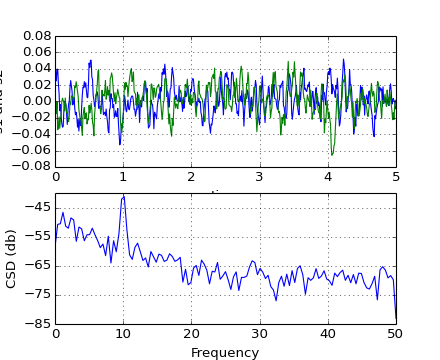Additional kwargs: hold = [True|False] overrides default hold state

matplotlib.pyplot.delaxes(*args)

Remove an axes from the current figure. If ax doesn’t exist, an error will be raised.

delaxes(): delete the current axes

matplotlib.pyplot.disconnect(cid)

Disconnect callback id cid

Example usage:

```cid = canvas.mpl_connect('button_press_event', on_press)
#...later
canvas.mpl_disconnect(cid)
```
matplotlib.pyplot.draw()

Redraw the current figure.

This is used in interactive mode to update a figure that has been altered using one or more plot object method calls; it is not needed if figure modification is done entirely with pyplot functions, if a sequence of modifications ends with a pyplot function, or if matplotlib is in non-interactive mode and the sequence of modifications ends with show() or savefig().

A more object-oriented alternative, given any Figure instance, fig, that was created using a pyplot function, is:

```fig.canvas.draw()
```
matplotlib.pyplot.errorbar(x, y, yerr=None, xerr=None, fmt='-', ecolor=None, elinewidth=None, capsize=3, barsabove=False, lolims=False, uplims=False, xlolims=False, xuplims=False, errorevery=1, capthick=None, hold=None, **kwargs)

Plot an errorbar graph.

Call signature:

```errorbar(x, y, yerr=None, xerr=None,
fmt='-', ecolor=None, elinewidth=None, capsize=3,
barsabove=False, lolims=False, uplims=False,
xlolims=False, xuplims=False, errorevery=1,
capthick=None)
```

Plot x versus y with error deltas in yerr and xerr. Vertical errorbars are plotted if yerr is not None. Horizontal errorbars are plotted if xerr is not None.

x, y, xerr, and yerr can all be scalars, which plots a single error bar at x, y.

Optional keyword arguments:

xerr/yerr: [ scalar | N, Nx1, or 2xN array-like ]

If a scalar number, len(N) array-like object, or an Nx1 array-like object, errorbars are drawn at +/-value relative to the data.

If a sequence of shape 2xN, errorbars are drawn at -row1 and +row2 relative to the data.

fmt: ‘-‘
The plot format symbol. If fmt is None, only the errorbars are plotted. This is used for adding errorbars to a bar plot, for example.
ecolor: [ None | mpl color ]
A matplotlib color arg which gives the color the errorbar lines; if None, use the marker color.
elinewidth: scalar
The linewidth of the errorbar lines. If None, use the linewidth.
capsize: scalar
The length of the error bar caps in points
capthick: scalar
An alias kwarg to markeredgewidth (a.k.a. - mew). This setting is a more sensible name for the property that controls the thickness of the error bar cap in points. For backwards compatibility, if mew or markeredgewidth are given, then they will over-ride capthick. This may change in future releases.
barsabove: [ True | False ]
if True, will plot the errorbars above the plot symbols. Default is below.
lolims / uplims / xlolims / xuplims: [ False | True ]
These arguments can be used to indicate that a value gives only upper/lower limits. In that case a caret symbol is used to indicate this. lims-arguments may be of the same type as xerr and yerr.
errorevery: positive integer
subsamples the errorbars. e.g., if everyerror=5, errorbars for every 5-th datapoint will be plotted. The data plot itself still shows all data points.

All other keyword arguments are passed on to the plot command for the markers. For example, this code makes big red squares with thick green edges:

```x,y,yerr = rand(3,10)
errorbar(x, y, yerr, marker='s',
mfc='red', mec='green', ms=20, mew=4)
```

where mfc, mec, ms and mew are aliases for the longer property names, markerfacecolor, markeredgecolor, markersize and markeredgewith.

valid kwargs for the marker properties are

Property Description
agg_filter unknown
alpha float (0.0 transparent through 1.0 opaque)
animated [True | False]
antialiased or aa [True | False]
axes an Axes instance
clip_box a matplotlib.transforms.Bbox instance
clip_on [True | False]
clip_path [ (Path, Transform) | Patch | None ]
color or c any matplotlib color
contains a callable function
dash_capstyle [‘butt’ | ‘round’ | ‘projecting’]
dash_joinstyle [‘miter’ | ‘round’ | ‘bevel’]
dashes sequence of on/off ink in points
drawstyle [‘default’ | ‘steps’ | ‘steps-pre’ | ‘steps-mid’ | ‘steps-post’]
figure a matplotlib.figure.Figure instance
fillstyle [‘full’ | ‘left’ | ‘right’ | ‘bottom’ | ‘top’ | ‘none’]
gid an id string
label string or anything printable with ‘%s’ conversion.
linestyle or ls ['-' | '--' | '-.' | ':' | 'None' | ' ' | ''] and any drawstyle in combination with a linestyle, e.g., 'steps--'.
linewidth or lw float value in points
lod [True | False]
marker unknown
markeredgecolor or mec any matplotlib color
markeredgewidth or mew float value in points
markerfacecolor or mfc any matplotlib color
markerfacecoloralt or mfcalt any matplotlib color
markersize or ms float
markevery None | integer | (startind, stride)
path_effects unknown
picker float distance in points or callable pick function fn(artist, event)
rasterized [True | False | None]
sketch_params unknown
snap unknown
solid_capstyle [‘butt’ | ‘round’ | ‘projecting’]
solid_joinstyle [‘miter’ | ‘round’ | ‘bevel’]
transform a matplotlib.transforms.Transform instance
url a url string
visible [True | False]
xdata 1D array
ydata 1D array
zorder any number

Returns (plotline, caplines, barlinecols):

plotline: Line2D instance
x, y plot markers and/or line
caplines: list of error bar cap
Line2D instances
barlinecols: list of
LineCollection instances for the horizontal and vertical error ranges.

Example: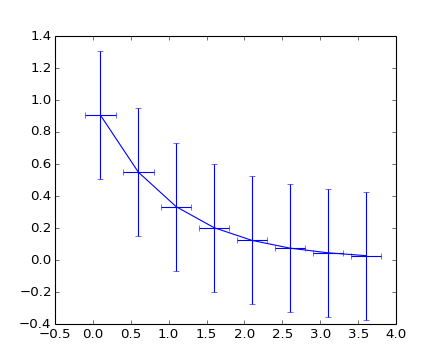Additional kwargs: hold = [True|False] overrides default hold state

matplotlib.pyplot.eventplot(positions, orientation='horizontal', lineoffsets=1, linelengths=1, linewidths=None, colors=None, linestyles='solid', hold=None, **kwargs)

Plot identical parallel lines at specific positions.

Call signature:

```eventplot(positions, orientation='horizontal', lineoffsets=0,
linelengths=1, linewidths=None, color =None,
linestyles='solid'```

Plot parallel lines at the given positions. positions should be a 1D or 2D array-like object, with each row corresponding to a row or column of lines.

This type of plot is commonly used in neuroscience for representing neural events, where it is commonly called a spike raster, dot raster, or raster plot.

However, it is useful in any situation where you wish to show the timing or position of multiple sets of discrete events, such as the arrival times of people to a business on each day of the month or the date of hurricanes each year of the last century.

orientation : [ ‘horizonal’ | ‘vertical’ ]
‘horizonal’ : the lines will be vertical and arranged in rows “vertical’ : lines will be horizontal and arranged in columns
lineoffsets :
A float or array-like containing floats.
linelengths :
A float or array-like containing floats.
linewidths :
A float or array-like containing floats.
colors
must be a sequence of RGBA tuples (eg arbitrary color strings, etc, not allowed) or a list of such sequences
linestyles :
[ ‘solid’ | ‘dashed’ | ‘dashdot’ | ‘dotted’ ] or an array of these values

For linelengths, linewidths, colors, and linestyles, if only a single value is given, that value is applied to all lines. If an array-like is given, it must have the same length as positions, and each value will be applied to the corresponding row or column in positions.

Returns a list of matplotlib.collections.EventCollection objects that were added.

kwargs are LineCollection properties:

Property Description
agg_filter unknown
alpha float or None
animated [True | False]
antialiased or antialiaseds Boolean or sequence of booleans
array unknown
axes an Axes instance
clim a length 2 sequence of floats
clip_box a matplotlib.transforms.Bbox instance
clip_on [True | False]
clip_path [ (Path, Transform) | Patch | None ]
cmap a colormap or registered colormap name
color matplotlib color arg or sequence of rgba tuples
contains a callable function
edgecolor or edgecolors matplotlib color arg or sequence of rgba tuples
facecolor or facecolors matplotlib color arg or sequence of rgba tuples
figure a matplotlib.figure.Figure instance
gid an id string
hatch [ ‘/’ | ‘\’ | ‘|’ | ‘-‘ | ‘+’ | ‘x’ | ‘o’ | ‘O’ | ‘.’ | ‘*’ ]
label string or anything printable with ‘%s’ conversion.
linestyle or linestyles or dashes [‘solid’ | ‘dashed’, ‘dashdot’, ‘dotted’ | (offset, on-off-dash-seq) ]
linewidth or lw or linewidths float or sequence of floats
lod [True | False]
norm unknown
offset_position unknown
offsets float or sequence of floats
path_effects unknown
paths unknown
picker [None|float|boolean|callable]
rasterized [True | False | None]
segments unknown
sketch_params unknown
snap unknown
transform Transform instance
url a url string
urls unknown
verts unknown
visible [True | False]
zorder any number

Example: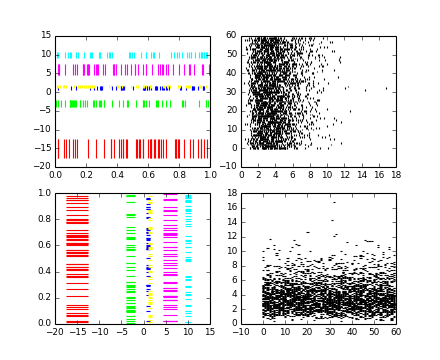Additional kwargs: hold = [True|False] overrides default hold state

matplotlib.pyplot.figimage(*args, **kwargs)

Adds a non-resampled image to the figure.

call signatures:

```figimage(X, **kwargs)
```

adds a non-resampled array X to the figure.

```figimage(X, xo, yo)
```

with pixel offsets xo, yo,

X must be a float array:

• If X is MxN, assume luminance (grayscale)
• If X is MxNx3, assume RGB
• If X is MxNx4, assume RGBA

Optional keyword arguments:

Keyword Description
xo or yo An integer, the x and y image offset in pixels
cmap a matplotlib.colors.Colormap instance, eg cm.jet. If None, default to the rc image.cmap value
norm a matplotlib.colors.Normalize instance. The default is normalization(). This scales luminance -> 0-1
vmin|vmax are used to scale a luminance image to 0-1. If either is None, the min and max of the luminance values will be used. Note if you pass a norm instance, the settings for vmin and vmax will be ignored.
alpha the alpha blending value, default is None
origin [ ‘upper’ | ‘lower’ ] Indicates where the [0,0] index of the array is in the upper left or lower left corner of the axes. Defaults to the rc image.origin value

figimage complements the axes image (imshow()) which will be resampled to fit the current axes. If you want a resampled image to fill the entire figure, you can define an Axes with size [0,1,0,1].

An matplotlib.image.FigureImage instance is returned.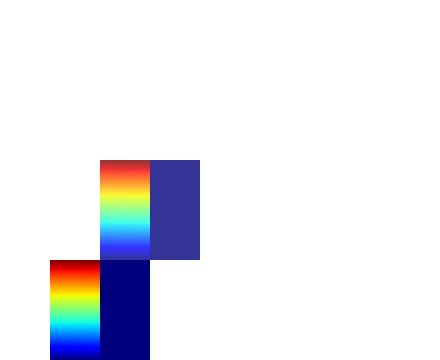Additional kwargs are Artist kwargs passed on to :class:`~matplotlib.image.FigureImage`Addition kwargs: hold = [True|False] overrides default hold state

matplotlib.pyplot.figlegend(handles, labels, loc, **kwargs)

Place a legend in the figure.

labels
a sequence of strings
handles
a sequence of Line2D or Patch instances
loc
can be a string or an integer specifying the legend location

A matplotlib.legend.Legend instance is returned.

Example:

```figlegend( (line1, line2, line3),
('label1', 'label2', 'label3'),
'upper right' )
```

legend()

matplotlib.pyplot.figtext(*args, **kwargs)

Call signature:

```text(x, y, s, fontdict=None, **kwargs)
```

Add text to figure at location x, y (relative 0-1 coords). See text() for the meaning of the other arguments.

kwargs control the Text properties:

Property Description
agg_filter unknown
alpha float (0.0 transparent through 1.0 opaque)
animated [True | False]
axes an Axes instance
backgroundcolor any matplotlib color
bbox rectangle prop dict
clip_box a matplotlib.transforms.Bbox instance
clip_on [True | False]
clip_path [ (Path, Transform) | Patch | None ]
color any matplotlib color
contains a callable function
family or fontfamily or fontname or name [FONTNAME | ‘serif’ | ‘sans-serif’ | ‘cursive’ | ‘fantasy’ | ‘monospace’ ]
figure a matplotlib.figure.Figure instance
fontproperties or font_properties a matplotlib.font_manager.FontProperties instance
gid an id string
horizontalalignment or ha [ ‘center’ | ‘right’ | ‘left’ ]
label string or anything printable with ‘%s’ conversion.
linespacing float (multiple of font size)
lod [True | False]
multialignment [‘left’ | ‘right’ | ‘center’ ]
path_effects unknown
picker [None|float|boolean|callable]
position (x,y)
rasterized [True | False | None]
rotation [ angle in degrees | ‘vertical’ | ‘horizontal’ ]
rotation_mode unknown
size or fontsize [size in points | ‘xx-small’ | ‘x-small’ | ‘small’ | ‘medium’ | ‘large’ | ‘x-large’ | ‘xx-large’ ]
sketch_params unknown
snap unknown
stretch or fontstretch [a numeric value in range 0-1000 | ‘ultra-condensed’ | ‘extra-condensed’ | ‘condensed’ | ‘semi-condensed’ | ‘normal’ | ‘semi-expanded’ | ‘expanded’ | ‘extra-expanded’ | ‘ultra-expanded’ ]
style or fontstyle [ ‘normal’ | ‘italic’ | ‘oblique’]
text string or anything printable with ‘%s’ conversion.
transform Transform instance
url a url string
variant or fontvariant [ ‘normal’ | ‘small-caps’ ]
verticalalignment or va or ma [ ‘center’ | ‘top’ | ‘bottom’ | ‘baseline’ ]
visible [True | False]
weight or fontweight [a numeric value in range 0-1000 | ‘ultralight’ | ‘light’ | ‘normal’ | ‘regular’ | ‘book’ | ‘medium’ | ‘roman’ | ‘semibold’ | ‘demibold’ | ‘demi’ | ‘bold’ | ‘heavy’ | ‘extra bold’ | ‘black’ ]
x float
y float
zorder any number
matplotlib.pyplot.figure(num=None, figsize=None, dpi=None, facecolor=None, edgecolor=None, frameon=True, FigureClass=<class 'matplotlib.figure.Figure'>, **kwargs)

Creates a new figure.

Parameters: num : integer or string, optional, default: none If not provided, a new figure will be created, and a the figure number will be increamted. The figure objects holds this number in a number attribute. If num is provided, and a figure with this id already exists, make it active, and returns a reference to it. If this figure does not exists, create it and returns it. If num is a string, the window title will be set to this figure’s num. figsize : tuple of integers, optional, default width, height in inches. If not provided, defaults to rc figure.figsize. dpi : integer, optional, default ; None resolution of the figure. If not provided, defaults to rc figure.dpi. facecolor : : the background color; If not provided, defaults to rc figure.facecolor edgecolor : : the border color. If not provided, defaults to rc figure.edgecolor figure : Figure The Figure instance returned will also be passed to new_figure_manager in the backends, which allows to hook custom Figure classes into the pylab interface. Additional kwargs will be passed to the figure init function.
matplotlib.pyplot.fill(*args, **kwargs)

Plot filled polygons.

Call signature:

```fill(*args, **kwargs)
```

args is a variable length argument, allowing for multiple x, y pairs with an optional color format string; see plot() for details on the argument parsing. For example, to plot a polygon with vertices at x, y in blue.:

```ax.fill(x,y, 'b' )
```

An arbitrary number of x, y, color groups can be specified:

```ax.fill(x1, y1, 'g', x2, y2, 'r')
```

Return value is a list of Patch instances that were added.

The same color strings that plot() supports are supported by the fill format string.

If you would like to fill below a curve, e.g., shade a region between 0 and y along x, use fill_between()

The closed kwarg will close the polygon when True (default).

kwargs control the Polygon properties:

Property Description
agg_filter unknown
alpha float or None
animated [True | False]
antialiased or aa [True | False] or None for default
axes an Axes instance
clip_box a matplotlib.transforms.Bbox instance
clip_on [True | False]
clip_path [ (Path, Transform) | Patch | None ]
color matplotlib color spec
contains a callable function
edgecolor or ec mpl color spec, or None for default, or ‘none’ for no color
facecolor or fc mpl color spec, or None for default, or ‘none’ for no color
figure a matplotlib.figure.Figure instance
fill [True | False]
gid an id string
hatch [‘/’ | ‘\’ | ‘|’ | ‘-‘ | ‘+’ | ‘x’ | ‘o’ | ‘O’ | ‘.’ | ‘*’]
label string or anything printable with ‘%s’ conversion.
linestyle or ls [‘solid’ | ‘dashed’ | ‘dashdot’ | ‘dotted’]
linewidth or lw float or None for default
lod [True | False]
path_effects unknown
picker [None|float|boolean|callable]
rasterized [True | False | None]
sketch_params unknown
snap unknown
transform Transform instance
url a url string
visible [True | False]
zorder any number

Example: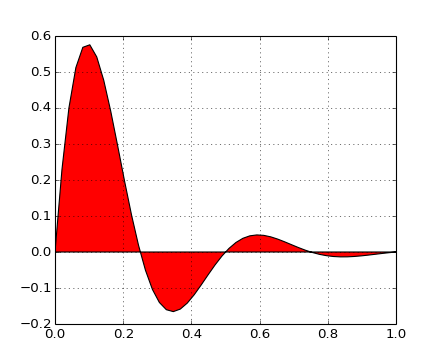Additional kwargs: hold = [True|False] overrides default hold state

matplotlib.pyplot.fill_between(x, y1, y2=0, where=None, interpolate=False, hold=None, **kwargs)

Make filled polygons between two curves.

Call signature:

```fill_between(x, y1, y2=0, where=None, **kwargs)
```

Create a PolyCollection filling the regions between y1 and y2 where where==True

x :
An N-length array of the x data
y1 :
An N-length array (or scalar) of the y data
y2 :
An N-length array (or scalar) of the y data
where :
If None, default to fill between everywhere. If not None, it is an N-length numpy boolean array and the fill will only happen over the regions where where==True.
interpolate :
If True, interpolate between the two lines to find the precise point of intersection. Otherwise, the start and end points of the filled region will only occur on explicit values in the x array.
kwargs :
Keyword args passed on to the PolyCollection.

kwargs control the Polygon properties:

Property Description
agg_filter unknown
alpha float or None
animated [True | False]
antialiased or antialiaseds Boolean or sequence of booleans
array unknown
axes an Axes instance
clim a length 2 sequence of floats
clip_box a matplotlib.transforms.Bbox instance
clip_on [True | False]
clip_path [ (Path, Transform) | Patch | None ]
cmap a colormap or registered colormap name
color matplotlib color arg or sequence of rgba tuples
contains a callable function
edgecolor or edgecolors matplotlib color arg or sequence of rgba tuples
facecolor or facecolors matplotlib color arg or sequence of rgba tuples
figure a matplotlib.figure.Figure instance
gid an id string
hatch [ ‘/’ | ‘\’ | ‘|’ | ‘-‘ | ‘+’ | ‘x’ | ‘o’ | ‘O’ | ‘.’ | ‘*’ ]
label string or anything printable with ‘%s’ conversion.
linestyle or linestyles or dashes [‘solid’ | ‘dashed’, ‘dashdot’, ‘dotted’ | (offset, on-off-dash-seq) ]
linewidth or lw or linewidths float or sequence of floats
lod [True | False]
norm unknown
offset_position unknown
offsets float or sequence of floats
path_effects unknown
picker [None|float|boolean|callable]
rasterized [True | False | None]
sketch_params unknown
snap unknown
transform Transform instance
url a url string
urls unknown
visible [True | False]
zorder any number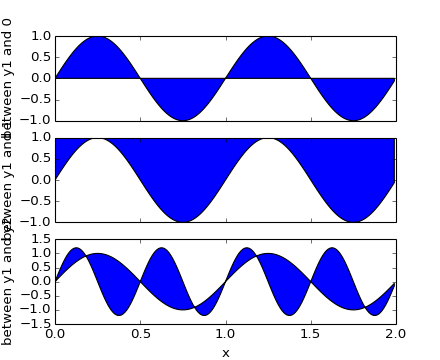(png, hires.png, pdf)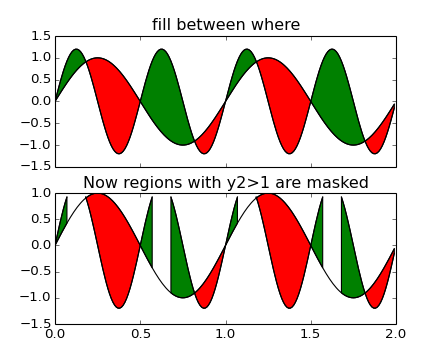(png, hires.png, pdf)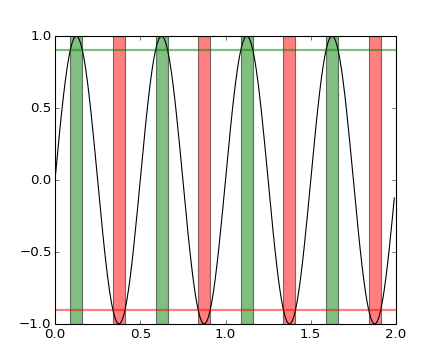(png, hires.png, pdf)

fill_betweenx()
for filling between two sets of x-values

Additional kwargs: hold = [True|False] overrides default hold state

matplotlib.pyplot.fill_betweenx(y, x1, x2=0, where=None, hold=None, **kwargs)

Make filled polygons between two horizontal curves.

Call signature:

```fill_betweenx(y, x1, x2=0, where=None, **kwargs)
```

Create a PolyCollection filling the regions between x1 and x2 where where==True

y :
An N-length array of the y data
x1 :
An N-length array (or scalar) of the x data
x2 :
An N-length array (or scalar) of the x data
where :
If None, default to fill between everywhere. If not None, it is a N length numpy boolean array and the fill will only happen over the regions where where==True
kwargs :
keyword args passed on to the PolyCollection

kwargs control the Polygon properties:

Property Description
agg_filter unknown
alpha float or None
animated [True | False]
antialiased or antialiaseds Boolean or sequence of booleans
array unknown
axes an Axes instance
clim a length 2 sequence of floats
clip_box a matplotlib.transforms.Bbox instance
clip_on [True | False]
clip_path [ (Path, Transform) | Patch | None ]
cmap a colormap or registered colormap name
color matplotlib color arg or sequence of rgba tuples
contains a callable function
edgecolor or edgecolors matplotlib color arg or sequence of rgba tuples
facecolor or facecolors matplotlib color arg or sequence of rgba tuples
figure a matplotlib.figure.Figure instance
gid an id string
hatch [ ‘/’ | ‘\’ | ‘|’ | ‘-‘ | ‘+’ | ‘x’ | ‘o’ | ‘O’ | ‘.’ | ‘*’ ]
label string or anything printable with ‘%s’ conversion.
linestyle or linestyles or dashes [‘solid’ | ‘dashed’, ‘dashdot’, ‘dotted’ | (offset, on-off-dash-seq) ]
linewidth or lw or linewidths float or sequence of floats
lod [True | False]
norm unknown
offset_position unknown
offsets float or sequence of floats
path_effects unknown
picker [None|float|boolean|callable]
rasterized [True | False | None]
sketch_params unknown
snap unknown
transform Transform instance
url a url string
urls unknown
visible [True | False]
zorder any number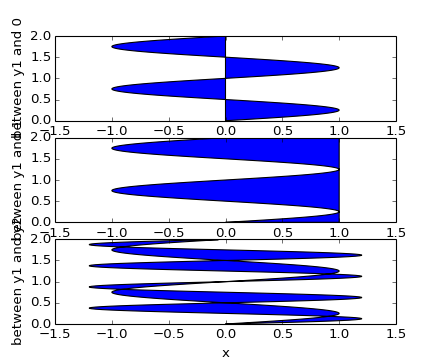(png, hires.png, pdf)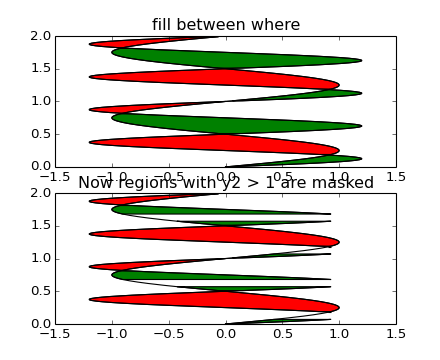(png, hires.png, pdf)

fill_between()
for filling between two sets of y-values

Additional kwargs: hold = [True|False] overrides default hold state

matplotlib.pyplot.findobj(o=None, match=None, include_self=True)

Find artist objects.

Recursively find all Artist instances contained in self.

match can be

• None: return all objects contained in artist.
• function with signature boolean = match(artist) used to filter matches
• class instance: e.g., Line2D. Only return artists of class type.

If include_self is True (default), include self in the list to be checked for a match.

matplotlib.pyplot.flag()

set the default colormap to flag and apply to current image if any. See help(colormaps) for more information

matplotlib.pyplot.gca(**kwargs)

Return the current axis instance. This can be used to control axis properties either using set or the Axes methods, for example, setting the xaxis range:

```plot(t,s)
set(gca(), 'xlim', [0,10])
```

or:

```plot(t,s)
a = gca()
a.set_xlim([0,10])
```
matplotlib.pyplot.gcf()

Return a reference to the current figure.

matplotlib.pyplot.gci()

Get the current colorable artist. Specifically, returns the current ScalarMappable instance (image or patch collection), or None if no images or patch collections have been defined. The commands imshow() and figimage() create Image instances, and the commands pcolor() and scatter() create Collection instances. The current image is an attribute of the current axes, or the nearest earlier axes in the current figure that contains an image.

matplotlib.pyplot.get_current_fig_manager()
matplotlib.pyplot.get_figlabels()

Return a list of existing figure labels.

matplotlib.pyplot.get_fignums()

Return a list of existing figure numbers.

matplotlib.pyplot.get_plot_commands()

Get a sorted list of all of the plotting commands.

matplotlib.pyplot.ginput(*args, **kwargs)

Call signature:

```ginput(self, n=1, timeout=30, show_clicks=True,
```

Blocking call to interact with the figure.

This will wait for n clicks from the user and return a list of the coordinates of each click.

If timeout is zero or negative, does not timeout.

If n is zero or negative, accumulate clicks until a middle click (or potentially both mouse buttons at once) terminates the input.

Right clicking cancels last input.

The buttons used for the various actions (adding points, removing points, terminating the inputs) can be overriden via the arguments mouse_add, mouse_pop and mouse_stop, that give the associated mouse button: 1 for left, 2 for middle, 3 for right.

The keyboard can also be used to select points in case your mouse does not have one or more of the buttons. The delete and backspace keys act like right clicking (i.e., remove last point), the enter key terminates input and any other key (not already used by the window manager) selects a point.

matplotlib.pyplot.gray()

set the default colormap to gray and apply to current image if any. See help(colormaps) for more information

matplotlib.pyplot.grid(b=None, which='major', axis='both', **kwargs)

Turn the axes grids on or off.

Call signature:

```grid(self, b=None, which='major', axis='both', **kwargs)
```

Set the axes grids on or off; b is a boolean. (For MATLAB compatibility, b may also be a string, ‘on’ or ‘off’.)

If b is None and len(kwargs)==0, toggle the grid state. If kwargs are supplied, it is assumed that you want a grid and b is thus set to True.

which can be ‘major’ (default), ‘minor’, or ‘both’ to control whether major tick grids, minor tick grids, or both are affected.

axis can be ‘both’ (default), ‘x’, or ‘y’ to control which set of gridlines are drawn.

kwargs are used to set the grid line properties, eg:

```ax.grid(color='r', linestyle='-', linewidth=2)
```

Valid Line2D kwargs are

Property Description
agg_filter unknown
alpha float (0.0 transparent through 1.0 opaque)
animated [True | False]
antialiased or aa [True | False]
axes an Axes instance
clip_box a matplotlib.transforms.Bbox instance
clip_on [True | False]
clip_path [ (Path, Transform) | Patch | None ]
color or c any matplotlib color
contains a callable function
dash_capstyle [‘butt’ | ‘round’ | ‘projecting’]
dash_joinstyle [‘miter’ | ‘round’ | ‘bevel’]
dashes sequence of on/off ink in points
drawstyle [‘default’ | ‘steps’ | ‘steps-pre’ | ‘steps-mid’ | ‘steps-post’]
figure a matplotlib.figure.Figure instance
fillstyle [‘full’ | ‘left’ | ‘right’ | ‘bottom’ | ‘top’ | ‘none’]
gid an id string
label string or anything printable with ‘%s’ conversion.
linestyle or ls ['-' | '--' | '-.' | ':' | 'None' | ' ' | ''] and any drawstyle in combination with a linestyle, e.g., 'steps--'.
linewidth or lw float value in points
lod [True | False]
marker unknown
markeredgecolor or mec any matplotlib color
markeredgewidth or mew float value in points
markerfacecolor or mfc any matplotlib color
markerfacecoloralt or mfcalt any matplotlib color
markersize or ms float
markevery None | integer | (startind, stride)
path_effects unknown
picker float distance in points or callable pick function fn(artist, event)
rasterized [True | False | None]
sketch_params unknown
snap unknown
solid_capstyle [‘butt’ | ‘round’ | ‘projecting’]
solid_joinstyle [‘miter’ | ‘round’ | ‘bevel’]
transform a matplotlib.transforms.Transform instance
url a url string
visible [True | False]
xdata 1D array
ydata 1D array
zorder any number
matplotlib.pyplot.hexbin(x, y, C=None, gridsize=100, bins=None, xscale='linear', yscale='linear', extent=None, cmap=None, norm=None, vmin=None, vmax=None, alpha=None, linewidths=None, edgecolors='none', reduce_C_function=<function mean at 0x16ead70>, mincnt=None, marginals=False, hold=None, **kwargs)

Make a hexagonal binning plot.

Call signature:

```hexbin(x, y, C = None, gridsize = 100, bins = None,
xscale = 'linear', yscale = 'linear',
cmap=None, norm=None, vmin=None, vmax=None,
alpha=None, linewidths=None, edgecolors='none'
reduce_C_function = np.mean, mincnt=None, marginals=True
**kwargs)```

Make a hexagonal binning plot of x versus y, where x, y are 1-D sequences of the same length, N. If C is None (the default), this is a histogram of the number of occurences of the observations at (x[i],y[i]).

If C is specified, it specifies values at the coordinate (x[i],y[i]). These values are accumulated for each hexagonal bin and then reduced according to reduce_C_function, which defaults to numpy’s mean function (np.mean). (If C is specified, it must also be a 1-D sequence of the same length as x and y.)

x, y and/or C may be masked arrays, in which case only unmasked points will be plotted.

Optional keyword arguments:

gridsize: [ 100 | integer ]
The number of hexagons in the x-direction, default is 100. The corresponding number of hexagons in the y-direction is chosen such that the hexagons are approximately regular. Alternatively, gridsize can be a tuple with two elements specifying the number of hexagons in the x-direction and the y-direction.
bins: [ None | ‘log’ | integer | sequence ]

If None, no binning is applied; the color of each hexagon directly corresponds to its count value.

If ‘log’, use a logarithmic scale for the color map. Internally,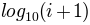is used to determine the hexagon color.

If an integer, divide the counts in the specified number of bins, and color the hexagons accordingly.

If a sequence of values, the values of the lower bound of the bins to be used.

xscale: [ ‘linear’ | ‘log’ ]
Use a linear or log10 scale on the horizontal axis.
scale: [ ‘linear’ | ‘log’ ]
Use a linear or log10 scale on the vertical axis.
mincnt: [ None | a positive integer ]
If not None, only display cells with more than mincnt number of points in the cell
marginals: [ True | False ]
if marginals is True, plot the marginal density as colormapped rectagles along the bottom of the x-axis and left of the y-axis
extent: [ None | scalars (left, right, bottom, top) ]
The limits of the bins. The default assigns the limits based on gridsize, x, y, xscale and yscale.

Other keyword arguments controlling color mapping and normalization arguments:

cmap: [ None | Colormap ]
a matplotlib.colors.Colormap instance. If None, defaults to rc image.cmap.
norm: [ None | Normalize ]
matplotlib.colors.Normalize instance is used to scale luminance data to 0,1.
vmin / vmax: scalar
vmin and vmax are used in conjunction with norm to normalize luminance data. If either are None, the min and max of the color array C is used. Note if you pass a norm instance, your settings for vmin and vmax will be ignored.
alpha: scalar between 0 and 1, or None
the alpha value for the patches
linewidths: [ None | scalar ]
If None, defaults to rc lines.linewidth. Note that this is a tuple, and if you set the linewidths argument you must set it as a sequence of floats, as required by RegularPolyCollection.

Other keyword arguments controlling the Collection properties:

edgecolors: [ None | 'none' | mpl color | color sequence ]

If 'none', draws the edges in the same color as the fill color. This is the default, as it avoids unsightly unpainted pixels between the hexagons.

If None, draws the outlines in the default color.

If a matplotlib color arg or sequence of rgba tuples, draws the outlines in the specified color.

Here are the standard descriptions of all the Collection kwargs:

Property Description
agg_filter unknown
alpha float or None
animated [True | False]
antialiased or antialiaseds Boolean or sequence of booleans
array unknown
axes an Axes instance
clim a length 2 sequence of floats
clip_box a matplotlib.transforms.Bbox instance
clip_on [True | False]
clip_path [ (Path, Transform) | Patch | None ]
cmap a colormap or registered colormap name
color matplotlib color arg or sequence of rgba tuples
contains a callable function
edgecolor or edgecolors matplotlib color arg or sequence of rgba tuples
facecolor or facecolors matplotlib color arg or sequence of rgba tuples
figure a matplotlib.figure.Figure instance
gid an id string
hatch [ ‘/’ | ‘\’ | ‘|’ | ‘-‘ | ‘+’ | ‘x’ | ‘o’ | ‘O’ | ‘.’ | ‘*’ ]
label string or anything printable with ‘%s’ conversion.
linestyle or linestyles or dashes [‘solid’ | ‘dashed’, ‘dashdot’, ‘dotted’ | (offset, on-off-dash-seq) ]
linewidth or lw or linewidths float or sequence of floats
lod [True | False]
norm unknown
offset_position unknown
offsets float or sequence of floats
path_effects unknown
picker [None|float|boolean|callable]
rasterized [True | False | None]
sketch_params unknown
snap unknown
transform Transform instance
url a url string
urls unknown
visible [True | False]
zorder any number

The return value is a PolyCollection instance; use get_array() on this PolyCollection to get the counts in each hexagon. If marginals is True, horizontal bar and vertical bar (both PolyCollections) will be attached to the return collection as attributes hbar and vbar.

Example: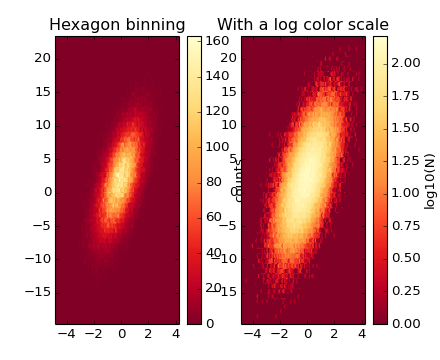Additional kwargs: hold = [True|False] overrides default hold state

matplotlib.pyplot.hist(x, bins=10, range=None, normed=False, weights=None, cumulative=False, bottom=None, histtype='bar', align='mid', orientation='vertical', rwidth=None, log=False, color=None, label=None, stacked=False, hold=None, **kwargs)

Plot a histogram.

Compute and draw the histogram of x. The return value is a tuple (n, bins, patches) or ([n0, n1, ...], bins, [patches0, patches1,...]) if the input contains multiple data.

Multiple data can be provided via x as a list of datasets of potentially different length ([x0, x1, ...]), or as a 2-D ndarray in which each column is a dataset. Note that the ndarray form is transposed relative to the list form.

Masked arrays are not supported at present.

Parameters: Returns: x : array_like, shape (n, ) Input values. bins : integer or array_like, optional, default: 10 If an integer is given, bins + 1 bin edges are returned, consistently with numpy.histogram() for numpy version >= 1.3. Unequally spaced bins are supported if bins is a sequence. range : tuple, optional, default: None The lower and upper range of the bins. Lower and upper outliers are ignored. If not provided, range is (x.min(), x.max()). Range has no effect if bins is a sequence. If bins is a sequence or range is specified, autoscaling is based on the specified bin range instead of the range of x. normed : boolean, optional, default: False If True, the first element of the return tuple will be the counts normalized to form a probability density, i.e., n/(len(x)`dbin), ie the integral of the histogram will sum to 1. If stacked is also True, the sum of the histograms is normalized to 1. weights : array_like, shape (n, ), optional, default: None An array of weights, of the same shape as x. Each value in x only contributes its associated weight towards the bin count (instead of 1). If normed is True, the weights are normalized, so that the integral of the density over the range remains 1. cumulative : boolean, optional, default If True, then a histogram is computed where each bin gives the counts in that bin plus all bins for smaller values. The last bin gives the total number of datapoints. If normed is also True then the histogram is normalized such that the last bin equals 1. If cumulative evaluates to less than 0 (e.g., -1), the direction of accumulation is reversed. In this case, if normed is also True, then the histogram is normalized such that the first bin equals 1. histtype : [‘bar’ | ‘barstacked’ | ‘step’ | ‘stepfilled’], optional The type of histogram to draw. ‘bar’ is a traditional bar-type histogram. If multiple data are given the bars are aranged side by side. ‘barstacked’ is a bar-type histogram where multiple data are stacked on top of each other. ‘step’ generates a lineplot that is by default unfilled. ‘stepfilled’ generates a lineplot that is by default filled. align : [‘left’ | ‘mid’ | ‘right’], optional, default: ‘mid’ Controls how the histogram is plotted. ‘left’: bars are centered on the left bin edges. ‘mid’: bars are centered between the bin edges. ‘right’: bars are centered on the right bin edges. orientation : [‘horizontal’ | ‘vertical’], optional If ‘horizontal’, barh will be used for bar-type histograms and the bottom kwarg will be the left edges. rwidth : scalar, optional, default: None The relative width of the bars as a fraction of the bin width. If None, automatically compute the width. Ignored if histtype = ‘step’ or ‘stepfilled’. log : boolean, optional, default If True, the histogram axis will be set to a log scale. If log is True and x is a 1D array, empty bins will be filtered out and only the non-empty (n, bins, patches) will be returned. color : color or array_like of colors, optional, default: None Color spec or sequence of color specs, one per dataset. Default (None) uses the standard line color sequence. label : string, optional, default: ‘’ String, or sequence of strings to match multiple datasets. Bar charts yield multiple patches per dataset, but only the first gets the label, so that the legend command will work as expected. stacked : boolean, optional, default If True, multiple data are stacked on top of each other If False multiple data are aranged side by side if histtype is ‘bar’ or on top of each other if histtype is ‘step’ tuple : (n, bins, patches) or ([n0, n1, ...], bins, [patches0, patches1,...]) kwargs : Patch properties

hist2d
2D histograms

Notes

Until numpy release 1.5, the underlying numpy histogram function was incorrect with normed`=`True if bin sizes were unequal. MPL inherited that error. It is now corrected within MPL when using earlier numpy versions.

Examples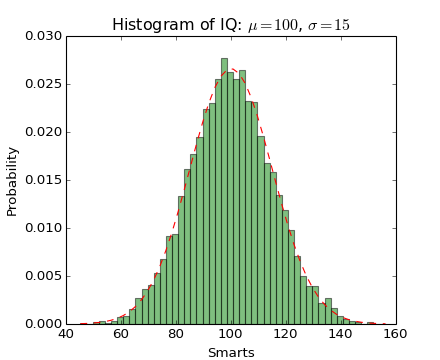Additional kwargs: hold = [True|False] overrides default hold state

matplotlib.pyplot.hist2d(x, y, bins=10, range=None, normed=False, weights=None, cmin=None, cmax=None, hold=None, **kwargs)

Make a 2D histogram plot.

Parameters: Returns: x, y: array_like, shape (n, ) : Input values bins: [None | int | [int, int] | array_like | [array, array]] : The bin specification: If int, the number of bins for the two dimensions (nx=ny=bins). If [int, int], the number of bins in each dimension (nx, ny = bins). If array_like, the bin edges for the two dimensions (x_edges=y_edges=bins). If [array, array], the bin edges in each dimension (x_edges, y_edges = bins). The default value is 10. range : array_like shape(2, 2), optional, default: None The leftmost and rightmost edges of the bins along each dimension (if not specified explicitly in the bins parameters): [[xmin, xmax], [ymin, ymax]]. All values outside of this range will be considered outliers and not tallied in the histogram. normed : boolean, optional, default: False Normalize histogram. weights : array_like, shape (n, ), optional, default: None An array of values w_i weighing each sample (x_i, y_i). cmin : scalar, optional, default: None All bins that has count less than cmin will not be displayed and these count values in the return value count histogram will also be set to nan upon return cmax : scalar, optional, default: None All bins that has count more than cmax will not be displayed (set to none before passing to imshow) and these count values in the return value count histogram will also be set to nan upon return The return value is ``(counts, xedges, yedges, Image)``. : kwargs : pcolorfast() properties.

hist
1D histogram

Notes

Rendering the histogram with a logarithmic color scale is accomplished by passing a colors.LogNorm instance to the norm keyword argument.

Examples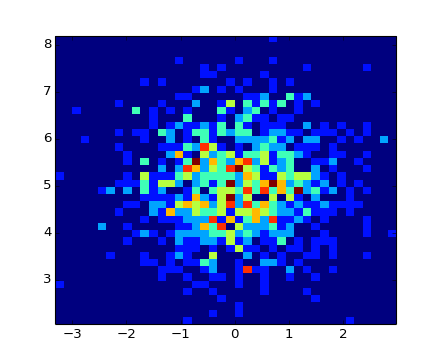Additional kwargs: hold = [True|False] overrides default hold state

matplotlib.pyplot.hlines(y, xmin, xmax, colors='k', linestyles='solid', label='', hold=None, **kwargs)

Plot horizontal lines.

Plot horizontal lines at each y from xmin to xmax.

Parameters: Returns: y : scalar or 1D array_like y-indexes where to plot the lines. xmin, xmax : scalar or 1D array_like Respective beginning and end of each line. If scalars are provided, all lines will have same length. colors : array_like of colors, optional, default: ‘k’ linestyles : [‘solid’ | ‘dashed’ | ‘dashdot’ | ‘dotted’], optional label : string, optional, default: ‘’ lines : LineCollection kwargs : LineCollection properties.

vlines
vertical lines

Examples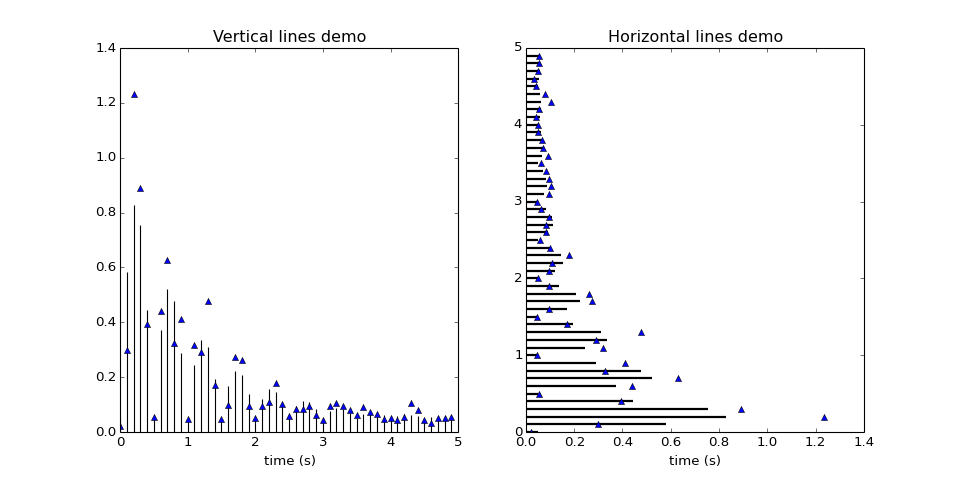Additional kwargs: hold = [True|False] overrides default hold state

matplotlib.pyplot.hold(b=None)

Set the hold state. If b is None (default), toggle the hold state, else set the hold state to boolean value b:

```hold()      # toggle hold
hold(True)  # hold is on
hold(False) # hold is off
```

When hold is True, subsequent plot commands will be added to the current axes. When hold is False, the current axes and figure will be cleared on the next plot command.

matplotlib.pyplot.hot()

set the default colormap to hot and apply to current image if any. See help(colormaps) for more information

matplotlib.pyplot.hsv()

set the default colormap to hsv and apply to current image if any. See help(colormaps) for more information

Read an image from a file into an array.

fname may be a string path or a Python file-like object. If using a file object, it must be opened in binary mode.

If format is provided, will try to read file of that type, otherwise the format is deduced from the filename. If nothing can be deduced, PNG is tried.

Return value is a numpy.array. For grayscale images, the return array is MxN. For RGB images, the return value is MxNx3. For RGBA images the return value is MxNx4.

matplotlib can only read PNGs natively, but if PIL is installed, it will use it to load the image and return an array (if possible) which can be used with imshow().

matplotlib.pyplot.imsave(*args, **kwargs)

Save an array as in image file.

The output formats available depend on the backend being used.

Arguments:
fname:
A string containing a path to a filename, or a Python file-like object. If format is None and fname is a string, the output format is deduced from the extension of the filename.
arr:
An MxN (luminance), MxNx3 (RGB) or MxNx4 (RGBA) array.
Keyword arguments:
vmin/vmax: [ None | scalar ]
vmin and vmax set the color scaling for the image by fixing the values that map to the colormap color limits. If either vmin or vmax is None, that limit is determined from the arr min/max value.
cmap:
cmap is a colors.Colormap instance, eg cm.jet. If None, default to the rc image.cmap value.
format:
One of the file extensions supported by the active backend. Most backends support png, pdf, ps, eps and svg.
origin
[ ‘upper’ | ‘lower’ ] Indicates where the [0,0] index of the array is in the upper left or lower left corner of the axes. Defaults to the rc image.origin value.
dpi
The DPI to store in the metadata of the file. This does not affect the resolution of the output image.
matplotlib.pyplot.imshow(X, cmap=None, norm=None, aspect=None, interpolation=None, alpha=None, vmin=None, vmax=None, origin=None, extent=None, shape=None, filternorm=1, filterrad=4.0, imlim=None, resample=None, url=None, hold=None, **kwargs)

Display an image on the axes.

Parameters: Returns: X : array_like, shape (n, m) or (n, m, 3) or (n, m, 4) Display the image in X to current axes. X may be a float array, a uint8 array or a PIL image. If X is an array, it can have the following shapes: MxN – luminance (grayscale, float array only) MxNx3 – RGB (float or uint8 array) MxNx4 – RGBA (float or uint8 array) The value for each component of MxNx3 and MxNx4 float arrays should be in the range 0.0 to 1.0; MxN float arrays may be normalised. cmap : Colormap, optional, default: None If None, default to rc image.cmap value. cmap is ignored when X has RGB(A) information aspect : [‘auto’ | ‘equal’ | scalar], optional, default: None If ‘auto’, changes the image aspect ratio to match that of the axes. If ‘equal’, and extent is None, changes the axes aspect ratio to match that of the image. If extent is not None, the axes aspect ratio is changed to match that of the extent. If None, default to rc image.aspect value. interpolation : string, optional, default: None Acceptable values are ‘none’, ‘nearest’, ‘bilinear’, ‘bicubic’, ‘spline16’, ‘spline36’, ‘hanning’, ‘hamming’, ‘hermite’, ‘kaiser’, ‘quadric’, ‘catrom’, ‘gaussian’, ‘bessel’, ‘mitchell’, ‘sinc’, ‘lanczos’ If interpolation is None, default to rc image.interpolation. See also the filternorm and filterrad parameters. If interpolation is ‘none’, then no interpolation is performed on the Agg, ps and pdf backends. Other backends will fall back to ‘nearest’. norm : Normalize, optional, default: None A Normalize instance is used to scale luminance data to 0, 1. If None, use the default func:normalize. norm is only used if X is an array of floats. vmin, vmax : scalar, optional, default: None vmin and vmax are used in conjunction with norm to normalize luminance data. Note if you pass a norm instance, your settings for vmin and vmax will be ignored. alpha : scalar, optional, default: None The alpha blending value, between 0 (transparent) and 1 (opaque) origin : [‘upper’ | ‘lower’], optional, default: None Place the [0,0] index of the array in the upper left or lower left corner of the axes. If None, default to rc image.origin. extent : scalars (left, right, bottom, top), optional, default: None Data limits for the axes. The default assigns zero-based row, column indices to the x, y centers of the pixels. shape : scalars (columns, rows), optional, default: None For raw buffer images filternorm : scalar, optional, default: 1 A parameter for the antigrain image resize filter. From the antigrain documentation, if filternorm = 1, the filter normalizes integer values and corrects the rounding errors. It doesn’t do anything with the source floating point values, it corrects only integers according to the rule of 1.0 which means that any sum of pixel weights must be equal to 1.0. So, the filter function must produce a graph of the proper shape. filterrad : scalar, optional, default: 4.0 The filter radius for filters that have a radius parameter, i.e. when interpolation is one of: ‘sinc’, ‘lanczos’ or ‘blackman’ image : AxesImage kwargs : Artist properties.

matshow
Plot a matrix or an array as an image.

Examples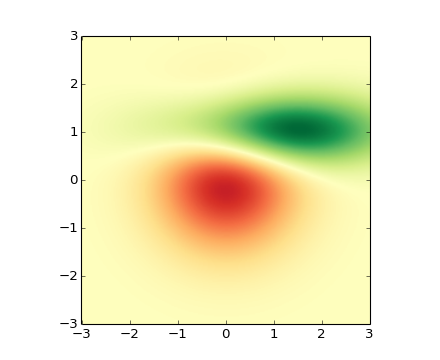Additional kwargs: hold = [True|False] overrides default hold state

matplotlib.pyplot.ioff()

Turn interactive mode off.

matplotlib.pyplot.ion()

Turn interactive mode on.

matplotlib.pyplot.ishold()

Return the hold status of the current axes.

matplotlib.pyplot.isinteractive()

Return status of interactive mode.

matplotlib.pyplot.jet()

set the default colormap to jet and apply to current image if any. See help(colormaps) for more information

matplotlib.pyplot.legend(*args, **kwargs)

Place a legend on the current axes.

Call signature:

```legend(*args, **kwargs)
```

Places legend at location loc. Labels are a sequence of strings and loc can be a string or an integer specifying the legend location.

To make a legend with existing lines:

```legend()
```

legend() by itself will try and build a legend using the label property of the lines/patches/collections. You can set the label of a line by doing:

```plot(x, y, label='my data')
```

or:

`line.set_label('my data').`

If label is set to ‘_nolegend_’, the item will not be shown in legend.

To automatically generate the legend from labels:

```legend( ('label1', 'label2', 'label3') )
```

To make a legend for a list of lines and labels:

```legend( (line1, line2, line3), ('label1', 'label2', 'label3') )
```

To make a legend at a given location, using a location argument:

```legend( ('label1', 'label2', 'label3'), loc='upper left')
```

or:

```legend((line1, line2, line3), ('label1', 'label2', 'label3'), loc=2)
```

The location codes are

Location String Location Code
‘best’ 0
‘upper right’ 1
‘upper left’ 2
‘lower left’ 3
‘lower right’ 4
‘right’ 5
‘center left’ 6
‘center right’ 7
‘lower center’ 8
‘upper center’ 9
‘center’ 10

Users can specify any arbitrary location for the legend using the bbox_to_anchor keyword argument. bbox_to_anchor can be an instance of BboxBase(or its derivatives) or a tuple of 2 or 4 floats. For example:

```loc = 'upper right', bbox_to_anchor = (0.5, 0.5)
```

will place the legend so that the upper right corner of the legend at the center of the axes.

The legend location can be specified in other coordinate, by using the bbox_transform keyword.

The loc itslef can be a 2-tuple giving x,y of the lower-left corner of the legend in axes coords (bbox_to_anchor is ignored).

Keyword arguments:

prop: [ None | FontProperties | dict ]
A matplotlib.font_manager.FontProperties instance. If prop is a dictionary, a new instance will be created with prop. If None, use rc settings.
fontsize: [size in points | ‘xx-small’ | ‘x-small’ | ‘small’ |
‘medium’ | ‘large’ | ‘x-large’ | ‘xx-large’]

Set the font size. May be either a size string, relative to the default font size, or an absolute font size in points. This argument is only used if prop is not specified.

numpoints: integer
The number of points in the legend for line
scatterpoints: integer
The number of points in the legend for scatter plot
scatteryoffsets: list of floats
a list of yoffsets for scatter symbols in legend
markerscale: [ None | scalar ]
The relative size of legend markers vs. original. If None, use rc settings.
frameon: [ True | False ]
if True, draw a frame around the legend. The default is set by the rcParam ‘legend.frameon’
fancybox: [ None | False | True ]
if True, draw a frame with a round fancybox. If None, use rc settings
shadow: [ None | False | True ]
If True, draw a shadow behind legend. If None, use rc settings.
framealpha: [None | float]
If not None, alpha channel for legend frame. Default None.
ncol : integer
number of columns. default is 1
mode : [ “expand” | None ]
if mode is “expand”, the legend will be horizontally expanded to fill the axes area (or bbox_to_anchor)
bbox_to_anchor: an instance of BboxBase or a tuple of 2 or 4 floats
the bbox that the legend will be anchored.
bbox_transform : [ an instance of Transform | None ]
the transform for the bbox. transAxes if None.
title : string
the legend title

Padding and spacing between various elements use following keywords parameters. These values are measure in font-size units. e.g., a fontsize of 10 points and a handlelength=5 implies a handlelength of 50 points. Values from rcParams will be used if None.

Keyword Description
borderpad the fractional whitespace inside the legend border
labelspacing the vertical space between the legend entries
handlelength the length of the legend handles
columnspacing the spacing between columns

Note

Not all kinds of artist are supported by the legend command. See Legend guide for details.

Example: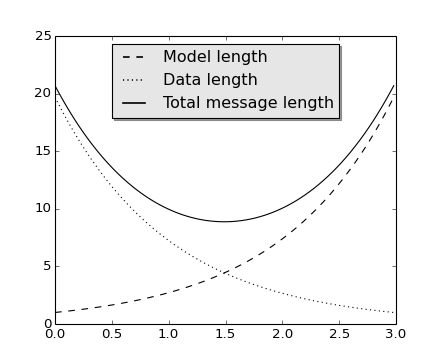matplotlib.pyplot.locator_params(axis='both', tight=None, **kwargs)

Control behavior of tick locators.

Keyword arguments:

axis
[‘x’ | ‘y’ | ‘both’] Axis on which to operate; default is ‘both’.
tight
[True | False | None] Parameter passed to autoscale_view(). Default is None, for no change.

Remaining keyword arguments are passed to directly to the set_params() method.

Typically one might want to reduce the maximum number of ticks and use tight bounds when plotting small subplots, for example:

```ax.locator_params(tight=True, nbins=4)
```

Because the locator is involved in autoscaling, autoscale_view() is called automatically after the parameters are changed.

This presently works only for the MaxNLocator used by default on linear axes, but it may be generalized.

matplotlib.pyplot.loglog(*args, **kwargs)

Make a plot with log scaling on both the x and y axis.

Call signature:

```loglog(*args, **kwargs)
```

loglog() supports all the keyword arguments of plot() and matplotlib.axes.Axes.set_xscale() / matplotlib.axes.Axes.set_yscale().

Notable keyword arguments:

basex/basey: scalar > 1
Base of the x/y logarithm
subsx/subsy: [ None | sequence ]
The location of the minor x/y ticks; None defaults to autosubs, which depend on the number of decades in the plot; see matplotlib.axes.Axes.set_xscale() / matplotlib.axes.Axes.set_yscale() for details
Non-positive values in x or y can be masked as invalid, or clipped to a very small positive number

The remaining valid kwargs are Line2D properties:

Property Description
agg_filter unknown
alpha float (0.0 transparent through 1.0 opaque)
animated [True | False]
antialiased or aa [True | False]
axes an Axes instance
clip_box a matplotlib.transforms.Bbox instance
clip_on [True | False]
clip_path [ (Path, Transform) | Patch | None ]
color or c any matplotlib color
contains a callable function
dash_capstyle [‘butt’ | ‘round’ | ‘projecting’]
dash_joinstyle [‘miter’ | ‘round’ | ‘bevel’]
dashes sequence of on/off ink in points
drawstyle [‘default’ | ‘steps’ | ‘steps-pre’ | ‘steps-mid’ | ‘steps-post’]
figure a matplotlib.figure.Figure instance
fillstyle [‘full’ | ‘left’ | ‘right’ | ‘bottom’ | ‘top’ | ‘none’]
gid an id string
label string or anything printable with ‘%s’ conversion.
linestyle or ls ['-' | '--' | '-.' | ':' | 'None' | ' ' | ''] and any drawstyle in combination with a linestyle, e.g., 'steps--'.
linewidth or lw float value in points
lod [True | False]
marker unknown
markeredgecolor or mec any matplotlib color
markeredgewidth or mew float value in points
markerfacecolor or mfc any matplotlib color
markerfacecoloralt or mfcalt any matplotlib color
markersize or ms float
markevery None | integer | (startind, stride)
path_effects unknown
picker float distance in points or callable pick function fn(artist, event)
rasterized [True | False | None]
sketch_params unknown
snap unknown
solid_capstyle [‘butt’ | ‘round’ | ‘projecting’]
solid_joinstyle [‘miter’ | ‘round’ | ‘bevel’]
transform a matplotlib.transforms.Transform instance
url a url string
visible [True | False]
xdata 1D array
ydata 1D array
zorder any number

Example: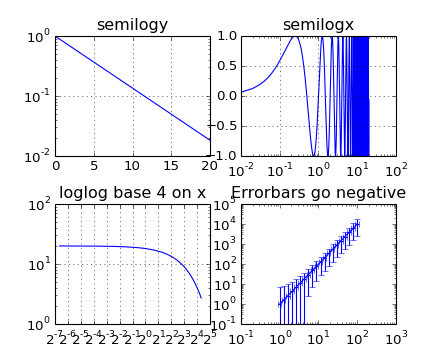Additional kwargs: hold = [True|False] overrides default hold state

matplotlib.pyplot.margins(*args, **kw)

Set or retrieve autoscaling margins.

signatures:

```margins()
```

returns xmargin, ymargin

```margins(margin)

margins(xmargin, ymargin)

margins(x=xmargin, y=ymargin)

margins(..., tight=False)
```

All three forms above set the xmargin and ymargin parameters. All keyword parameters are optional. A single argument specifies both xmargin and ymargin. The tight parameter is passed to autoscale_view(), which is executed after a margin is changed; the default here is True, on the assumption that when margins are specified, no additional padding to match tick marks is usually desired. Setting tight to None will preserve the previous setting.

Specifying any margin changes only the autoscaling; for example, if xmargin is not None, then xmargin times the X data interval will be added to each end of that interval before it is used in autoscaling.

matplotlib.pyplot.matshow(A, fignum=None, **kw)

Display an array as a matrix in a new figure window.

The origin is set at the upper left hand corner and rows (first dimension of the array) are displayed horizontally. The aspect ratio of the figure window is that of the array, unless this would make an excessively short or narrow figure.

Tick labels for the xaxis are placed on top.

With the exception of fignum, keyword arguments are passed to imshow(). You may set the origin kwarg to “lower” if you want the first row in the array to be at the bottom instead of the top.

fignum: [ None | integer | False ]

By default, matshow() creates a new figure window with automatic numbering. If fignum is given as an integer, the created figure will use this figure number. Because of how matshow() tries to set the figure aspect ratio to be the one of the array, if you provide the number of an already existing figure, strange things may happen.

If fignum is False or 0, a new figure window will NOT be created.

matplotlib.pyplot.minorticks_off()

Remove minor ticks from the current plot.

matplotlib.pyplot.minorticks_on()

Display minor ticks on the current plot.

Displaying minor ticks reduces performance; turn them off using minorticks_off() if drawing speed is a problem.

matplotlib.pyplot.over(func, *args, **kwargs)

Call a function with hold(True).

Calls:

```func(*args, **kwargs)
```

with hold(True) and then restores the hold state.

matplotlib.pyplot.pause(interval)

Pause for interval seconds.

If there is an active figure it will be updated and displayed, and the GUI event loop will run during the pause.

If there is no active figure, or if a non-interactive backend is in use, this executes time.sleep(interval).

This can be used for crude animation. For more complex animation, see matplotlib.animation.

This function is experimental; its behavior may be changed or extended in a future release.

matplotlib.pyplot.pcolor(*args, **kwargs)

Create a pseudocolor plot of a 2-D array.

Note

pcolor can be very slow for large arrays; consider using the similar but much faster pcolormesh() instead.

Call signatures:

```pcolor(C, **kwargs)
pcolor(X, Y, C, **kwargs)
```

C is the array of color values.

X and Y, if given, specify the (x, y) coordinates of the colored quadrilaterals; the quadrilateral for C[i,j] has corners at:

```(X[i,   j],   Y[i,   j]),
(X[i,   j+1], Y[i,   j+1]),
(X[i+1, j],   Y[i+1, j]),
(X[i+1, j+1], Y[i+1, j+1]).```

Ideally the dimensions of X and Y should be one greater than those of C; if the dimensions are the same, then the last row and column of C will be ignored.

Note that the the column index corresponds to the x-coordinate, and the row index corresponds to y; for details, see the Grid Orientation section below.

If either or both of X and Y are 1-D arrays or column vectors, they will be expanded as needed into the appropriate 2-D arrays, making a rectangular grid.

X, Y and C may be masked arrays. If either C[i, j], or one of the vertices surrounding C[i,j] (X or Y at [i, j], [i+1, j], [i, j+1],[i+1, j+1]) is masked, nothing is plotted.

Keyword arguments:

cmap: [ None | Colormap ]
A matplotlib.colors.Colormap instance. If None, use rc settings.
norm: [ None | Normalize ]
An matplotlib.colors.Normalize instance is used to scale luminance data to 0,1. If None, defaults to normalize().
vmin/vmax: [ None | scalar ]
vmin and vmax are used in conjunction with norm to normalize luminance data. If either is None, it is autoscaled to the respective min or max of the color array C. If not None, vmin or vmax passed in here override any pre-existing values supplied in the norm instance.
shading: [ ‘flat’ | ‘faceted’ ]

If ‘faceted’, a black grid is drawn around each rectangle; if ‘flat’, edges are not drawn. Default is ‘flat’, contrary to MATLAB.

edgecolors: [ None | 'none' | color | color sequence]

If None, the rc setting is used by default.

If 'none', edges will not be visible.

An mpl color or sequence of colors will set the edge color

alpha: 0 <= scalar <= 1 or None
the alpha blending value

Return value is a matplotlib.collections.Collection instance.

The grid orientation follows the MATLAB convention: an array C with shape (nrows, ncolumns) is plotted with the column number as X and the row number as Y, increasing up; hence it is plotted the way the array would be printed, except that the Y axis is reversed. That is, C is taken as C*(*y, x).

Similarly for meshgrid():

```x = np.arange(5)
y = np.arange(3)
X, Y = np.meshgrid(x, y)
```

is equivalent to:

```X = array([[0, 1, 2, 3, 4],
[0, 1, 2, 3, 4],
[0, 1, 2, 3, 4]])

Y = array([[0, 0, 0, 0, 0],
[1, 1, 1, 1, 1],
[2, 2, 2, 2, 2]])
```

so if you have:

```C = rand(len(x), len(y))
```

then you need to transpose C:

```pcolor(X, Y, C.T)
```

or:

```pcolor(C.T)
```

MATLAB pcolor() always discards the last row and column of C, but matplotlib displays the last row and column if X and Y are not specified, or if X and Y have one more row and column than C.

kwargs can be used to control the PolyCollection properties:

Property Description
agg_filter unknown
alpha float or None
animated [True | False]
antialiased or antialiaseds Boolean or sequence of booleans
array unknown
axes an Axes instance
clim a length 2 sequence of floats
clip_box a matplotlib.transforms.Bbox instance
clip_on [True | False]
clip_path [ (Path, Transform) | Patch | None ]
cmap a colormap or registered colormap name
color matplotlib color arg or sequence of rgba tuples
contains a callable function
edgecolor or edgecolors matplotlib color arg or sequence of rgba tuples
facecolor or facecolors matplotlib color arg or sequence of rgba tuples
figure a matplotlib.figure.Figure instance
gid an id string
hatch [ ‘/’ | ‘\’ | ‘|’ | ‘-‘ | ‘+’ | ‘x’ | ‘o’ | ‘O’ | ‘.’ | ‘*’ ]
label string or anything printable with ‘%s’ conversion.
linestyle or linestyles or dashes [‘solid’ | ‘dashed’, ‘dashdot’, ‘dotted’ | (offset, on-off-dash-seq) ]
linewidth or lw or linewidths float or sequence of floats
lod [True | False]
norm unknown
offset_position unknown
offsets float or sequence of floats
path_effects unknown
picker [None|float|boolean|callable]
rasterized [True | False | None]
sketch_params unknown
snap unknown
transform Transform instance
url a url string
urls unknown
visible [True | False]
zorder any number

Note

The default antialiaseds is False if the default edgecolors*=”none” is used. This eliminates artificial lines at patch boundaries, and works regardless of the value of alpha. If *edgecolors is not “none”, then the default antialiaseds is taken from rcParams[‘patch.antialiased’], which defaults to True. Stroking the edges may be preferred if alpha is 1, but will cause artifacts otherwise.

pcolormesh()
For an explanation of the differences between pcolor and pcolormesh.

Additional kwargs: hold = [True|False] overrides default hold state

matplotlib.pyplot.pcolormesh(*args, **kwargs)

Call signatures:

```pcolormesh(C)
pcolormesh(X, Y, C)
pcolormesh(C, **kwargs)
```

Create a pseudocolor plot of a 2-D array.

pcolormesh is similar to pcolor(), but uses a different mechanism and returns a different object; pcolor returns a PolyCollection but pcolormesh returns a QuadMesh. It is much faster, so it is almost always preferred for large arrays.

C may be a masked array, but X and Y may not. Masked array support is implemented via cmap and norm; in contrast, pcolor() simply does not draw quadrilaterals with masked colors or vertices.

Keyword arguments:

cmap: [ None | Colormap ]
A matplotlib.colors.Colormap instance. If None, use rc settings.
norm: [ None | Normalize ]
A matplotlib.colors.Normalize instance is used to scale luminance data to 0,1. If None, defaults to normalize().
vmin/vmax: [ None | scalar ]
vmin and vmax are used in conjunction with norm to normalize luminance data. If either is None, it is autoscaled to the respective min or max of the color array C. If not None, vmin or vmax passed in here override any pre-existing values supplied in the norm instance.
shading: [ ‘flat’ | ‘gouraud’ ]
edgecolors: [None | 'None' | 'face' | color |
color sequence]

If None, the rc setting is used by default.

If 'None', edges will not be visible.

If 'face', edges will have the same color as the faces.

An mpl color or sequence of colors will set the edge color

alpha: 0 <= scalar <= 1 or None
the alpha blending value

Return value is a matplotlib.collections.QuadMesh object.

kwargs can be used to control the matplotlib.collections.QuadMesh properties:

Property Description
agg_filter unknown
alpha float or None
animated [True | False]
antialiased or antialiaseds Boolean or sequence of booleans
array unknown
axes an Axes instance
clim a length 2 sequence of floats
clip_box a matplotlib.transforms.Bbox instance
clip_on [True | False]
clip_path [ (Path, Transform) | Patch | None ]
cmap a colormap or registered colormap name
color matplotlib color arg or sequence of rgba tuples
contains a callable function
edgecolor or edgecolors matplotlib color arg or sequence of rgba tuples
facecolor or facecolors matplotlib color arg or sequence of rgba tuples
figure a matplotlib.figure.Figure instance
gid an id string
hatch [ ‘/’ | ‘\’ | ‘|’ | ‘-‘ | ‘+’ | ‘x’ | ‘o’ | ‘O’ | ‘.’ | ‘*’ ]
label string or anything printable with ‘%s’ conversion.
linestyle or linestyles or dashes [‘solid’ | ‘dashed’, ‘dashdot’, ‘dotted’ | (offset, on-off-dash-seq) ]
linewidth or lw or linewidths float or sequence of floats
lod [True | False]
norm unknown
offset_position unknown
offsets float or sequence of floats
path_effects unknown
picker [None|float|boolean|callable]
rasterized [True | False | None]
sketch_params unknown
snap unknown
transform Transform instance
url a url string
urls unknown
visible [True | False]
zorder any number

pcolor()
For an explanation of the grid orientation and the expansion of 1-D X and/or Y to 2-D arrays.

Additional kwargs: hold = [True|False] overrides default hold state

Plot a pie chart.

Call signature:

```pie(x, explode=None, labels=None,
colors=('b', 'g', 'r', 'c', 'm', 'y', 'k', 'w'),
```

Make a pie chart of array x. The fractional area of each wedge is given by x/sum(x). If sum(x) <= 1, then the values of x give the fractional area directly and the array will not be normalized. The wedges are plotted counterclockwise, by default starting from the x-axis.

Keyword arguments:

explode: [ None | len(x) sequence ]
If not None, is a len(x) array which specifies the fraction of the radius with which to offset each wedge.
colors: [ None | color sequence ]
A sequence of matplotlib color args through which the pie chart will cycle.
labels: [ None | len(x) sequence of strings ]
A sequence of strings providing the labels for each wedge
autopct: [ None | format string | format function ]
If not None, is a string or function used to label the wedges with their numeric value. The label will be placed inside the wedge. If it is a format string, the label will be fmt%pct. If it is a function, it will be called.
pctdistance: scalar
The ratio between the center of each pie slice and the start of the text generated by autopct. Ignored if autopct is None; default is 0.6.
labeldistance: scalar
The radial distance at which the pie labels are drawn
shadow: [ False | True ]
Draw a shadow beneath the pie.
startangle: [ None | Offset angle ]
If not None, rotates the start of the pie chart by angle degrees counterclockwise from the x-axis.

radius: [ None | scalar ] The radius of the pie, if radius is None it will be set to 1.

The pie chart will probably look best if the figure and axes are square, or the Axes aspect is equal. e.g.:

```figure(figsize=(8,8))
ax = axes([0.1, 0.1, 0.8, 0.8])
```

or:

```axes(aspect=1)
```
Return value:

If autopct is None, return the tuple (patches, texts):

If autopct is not None, return the tuple (patches, texts, autotexts), where patches and texts are as above, and autotexts is a list of Text instances for the numeric labels.

Additional kwargs: hold = [True|False] overrides default hold state

matplotlib.pyplot.pink()

set the default colormap to pink and apply to current image if any. See help(colormaps) for more information

matplotlib.pyplot.plot(*args, **kwargs)

Plot lines and/or markers to the Axes. args is a variable length argument, allowing for multiple x, y pairs with an optional format string. For example, each of the following is legal:

```plot(x, y)        # plot x and y using default line style and color
plot(x, y, 'bo')  # plot x and y using blue circle markers
plot(y)           # plot y using x as index array 0..N-1
plot(y, 'r+')     # ditto, but with red plusses
```

If x and/or y is 2-dimensional, then the corresponding columns will be plotted.

An arbitrary number of x, y, fmt groups can be specified, as in:

```a.plot(x1, y1, 'g^', x2, y2, 'g-')
```

Return value is a list of lines that were added.

By default, each line is assigned a different color specified by a ‘color cycle’. To change this behavior, you can edit the axes.color_cycle rcParam. Alternatively, you can use set_default_color_cycle().

The following format string characters are accepted to control the line style or marker:

character description
'-' solid line style
'--' dashed line style
'-.' dash-dot line style
':' dotted line style
'.' point marker
',' pixel marker
'o' circle marker
'v' triangle_down marker
'^' triangle_up marker
'<' triangle_left marker
'>' triangle_right marker
'1' tri_down marker
'2' tri_up marker
'3' tri_left marker
'4' tri_right marker
's' square marker
'p' pentagon marker
'*' star marker
'h' hexagon1 marker
'H' hexagon2 marker
'+' plus marker
'x' x marker
'D' diamond marker
'd' thin_diamond marker
'|' vline marker
'_' hline marker

The following color abbreviations are supported:

character color
‘b’ blue
‘g’ green
‘r’ red
‘c’ cyan
‘m’ magenta
‘y’ yellow
‘k’ black
‘w’ white

In addition, you can specify colors in many weird and wonderful ways, including full names ('green'), hex strings ('#008000'), RGB or RGBA tuples ((0,1,0,1)) or grayscale intensities as a string ('0.8'). Of these, the string specifications can be used in place of a fmt group, but the tuple forms can be used only as kwargs.

Line styles and colors are combined in a single format string, as in 'bo' for blue circles.

The kwargs can be used to set line properties (any property that has a set_* method). You can use this to set a line label (for auto legends), linewidth, anitialising, marker face color, etc. Here is an example:

```plot([1,2,3], [1,2,3], 'go-', label='line 1', linewidth=2)
plot([1,2,3], [1,4,9], 'rs',  label='line 2')
axis([0, 4, 0, 10])
legend()
```

If you make multiple lines with one plot command, the kwargs apply to all those lines, e.g.:

```plot(x1, y1, x2, y2, antialised=False)
```

Neither line will be antialiased.

You do not need to use format strings, which are just abbreviations. All of the line properties can be controlled by keyword arguments. For example, you can set the color, marker, linestyle, and markercolor with:

```plot(x, y, color='green', linestyle='dashed', marker='o',
markerfacecolor='blue', markersize=12).```

See Line2D for details.

The kwargs are Line2D properties:

Property Description
agg_filter unknown
alpha float (0.0 transparent through 1.0 opaque)
animated [True | False]
antialiased or aa [True | False]
axes an Axes instance
clip_box a matplotlib.transforms.Bbox instance
clip_on [True | False]
clip_path [ (Path, Transform) | Patch | None ]
color or c any matplotlib color
contains a callable function
dash_capstyle [‘butt’ | ‘round’ | ‘projecting’]
dash_joinstyle [‘miter’ | ‘round’ | ‘bevel’]
dashes sequence of on/off ink in points
drawstyle [‘default’ | ‘steps’ | ‘steps-pre’ | ‘steps-mid’ | ‘steps-post’]
figure a matplotlib.figure.Figure instance
fillstyle [‘full’ | ‘left’ | ‘right’ | ‘bottom’ | ‘top’ | ‘none’]
gid an id string
label string or anything printable with ‘%s’ conversion.
linestyle or ls ['-' | '--' | '-.' | ':' | 'None' | ' ' | ''] and any drawstyle in combination with a linestyle, e.g., 'steps--'.
linewidth or lw float value in points
lod [True | False]
marker unknown
markeredgecolor or mec any matplotlib color
markeredgewidth or mew float value in points
markerfacecolor or mfc any matplotlib color
markerfacecoloralt or mfcalt any matplotlib color
markersize or ms float
markevery None | integer | (startind, stride)
path_effects unknown
picker float distance in points or callable pick function fn(artist, event)
rasterized [True | False | None]
sketch_params unknown
snap unknown
solid_capstyle [‘butt’ | ‘round’ | ‘projecting’]
solid_joinstyle [‘miter’ | ‘round’ | ‘bevel’]
transform a matplotlib.transforms.Transform instance
url a url string
visible [True | False]
xdata 1D array
ydata 1D array
zorder any number

kwargs scalex and scaley, if defined, are passed on to autoscale_view() to determine whether the x and y axes are autoscaled; the default is True.

Additional kwargs: hold = [True|False] overrides default hold state

matplotlib.pyplot.plot_date(x, y, fmt='bo', tz=None, xdate=True, ydate=False, hold=None, **kwargs)

Plot with data with dates.

Call signature:

```plot_date(x, y, fmt='bo', tz=None, xdate=True,
ydate=False, **kwargs)
```

Similar to the plot() command, except the x or y (or both) data is considered to be dates, and the axis is labeled accordingly.

x and/or y can be a sequence of dates represented as float days since 0001-01-01 UTC.

Keyword arguments:

fmt: string
The plot format string.
tz: [ None | timezone string | tzinfo instance]
The time zone to use in labeling dates. If None, defaults to rc value.
xdate: [ True | False ]
If True, the x-axis will be labeled with dates.
ydate: [ False | True ]
If True, the y-axis will be labeled with dates.

Note if you are using custom date tickers and formatters, it may be necessary to set the formatters/locators after the call to plot_date() since plot_date() will set the default tick locator to matplotlib.dates.AutoDateLocator (if the tick locator is not already set to a matplotlib.dates.DateLocator instance) and the default tick formatter to matplotlib.dates.AutoDateFormatter (if the tick formatter is not already set to a matplotlib.dates.DateFormatter instance).

Valid kwargs are Line2D properties:

Property Description
agg_filter unknown
alpha float (0.0 transparent through 1.0 opaque)
animated [True | False]
antialiased or aa [True | False]
axes an Axes instance
clip_box a matplotlib.transforms.Bbox instance
clip_on [True | False]
clip_path [ (Path, Transform) | Patch | None ]
color or c any matplotlib color
contains a callable function
dash_capstyle [‘butt’ | ‘round’ | ‘projecting’]
dash_joinstyle [‘miter’ | ‘round’ | ‘bevel’]
dashes sequence of on/off ink in points
drawstyle [‘default’ | ‘steps’ | ‘steps-pre’ | ‘steps-mid’ | ‘steps-post’]
figure a matplotlib.figure.Figure instance
fillstyle [‘full’ | ‘left’ | ‘right’ | ‘bottom’ | ‘top’ | ‘none’]
gid an id string
label string or anything printable with ‘%s’ conversion.
linestyle or ls ['-' | '--' | '-.' | ':' | 'None' | ' ' | ''] and any drawstyle in combination with a linestyle, e.g., 'steps--'.
linewidth or lw float value in points
lod [True | False]
marker unknown
markeredgecolor or mec any matplotlib color
markeredgewidth or mew float value in points
markerfacecolor or mfc any matplotlib color
markerfacecoloralt or mfcalt any matplotlib color
markersize or ms float
markevery None | integer | (startind, stride)
path_effects unknown
picker float distance in points or callable pick function fn(artist, event)
rasterized [True | False | None]
sketch_params unknown
snap unknown
solid_capstyle [‘butt’ | ‘round’ | ‘projecting’]
solid_joinstyle [‘miter’ | ‘round’ | ‘bevel’]
transform a matplotlib.transforms.Transform instance
url a url string
visible [True | False]
xdata 1D array
ydata 1D array
zorder any number

dates for helper functions

date2num(), num2date() and drange() for help on creating the required floating point dates.

Additional kwargs: hold = [True|False] overrides default hold state

matplotlib.pyplot.plotfile(fname, cols=(0, ), plotfuncs=None, comments='#', skiprows=0, checkrows=5, delimiter=', ', names=None, subplots=True, newfig=True, **kwargs)

Plot the data in in a file.

cols is a sequence of column identifiers to plot. An identifier is either an int or a string. If it is an int, it indicates the column number. If it is a string, it indicates the column header. matplotlib will make column headers lower case, replace spaces with underscores, and remove all illegal characters; so 'Adj Close*' will have name 'adj_close'.

• If len(cols) == 1, only that column will be plotted on the y axis.
• If len(cols) > 1, the first element will be an identifier for data for the x axis and the remaining elements will be the column indexes for multiple subplots if subplots is True (the default), or for lines in a single subplot if subplots is False.

plotfuncs, if not None, is a dictionary mapping identifier to an Axes plotting function as a string. Default is ‘plot’, other choices are ‘semilogy’, ‘fill’, ‘bar’, etc. You must use the same type of identifier in the cols vector as you use in the plotfuncs dictionary, e.g., integer column numbers in both or column names in both. If subplots is False, then including any function such as ‘semilogy’ that changes the axis scaling will set the scaling for all columns.

comments, skiprows, checkrows, delimiter, and names are all passed on to matplotlib.pylab.csv2rec() to load the data into a record array.

If newfig is True, the plot always will be made in a new figure; if False, it will be made in the current figure if one exists, else in a new figure.

kwargs are passed on to plotting functions.

Example usage:

```# plot the 2nd and 4th column against the 1st in two subplots
plotfile(fname, (0,1,3))

# plot using column names; specify an alternate plot type for volume
plotfuncs={'volume': 'semilogy'})
```

Note: plotfile is intended as a convenience for quickly plotting data from flat files; it is not intended as an alternative interface to general plotting with pyplot or matplotlib.

matplotlib.pyplot.polar(*args, **kwargs)

Make a polar plot.

call signature:

```polar(theta, r, **kwargs)
```

Multiple theta, r arguments are supported, with format strings, as in plot().

matplotlib.pyplot.prism()

set the default colormap to prism and apply to current image if any. See help(colormaps) for more information

matplotlib.pyplot.psd(x, NFFT=256, Fs=2, Fc=0, detrend=<function detrend_none at 0x2635de8>, window=<function window_hanning at 0x2635b90>, noverlap=0, pad_to=None, sides='default', scale_by_freq=None, hold=None, **kwargs)

Plot the power spectral density.

Call signature:

```psd(x, NFFT=256, Fs=2, Fc=0, detrend=mlab.detrend_none,
sides='default', scale_by_freq=None, **kwargs)
```

The power spectral density by Welch’s average periodogram method. The vector x is divided into NFFT length segments. Each segment is detrended by function detrend and windowed by function window. noverlap gives the length of the overlap between segments. The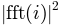of each segment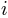are averaged to compute Pxx, with a scaling to correct for power loss due to windowing. Fs is the sampling frequency.

Keyword arguments:

NFFT: integer
The number of data points used in each block for the FFT. Must be even; a power 2 is most efficient. The default value is 256. This should NOT be used to get zero padding, or the scaling of the result will be incorrect. Use pad_to for this instead.
Fs: scalar
The sampling frequency (samples per time unit). It is used to calculate the Fourier frequencies, freqs, in cycles per time unit. The default value is 2.
detrend: callable
The function applied to each segment before fft-ing, designed to remove the mean or linear trend. Unlike in MATLAB, where the detrend parameter is a vector, in matplotlib is it a function. The pylab module defines detrend_none(), detrend_mean(), and detrend_linear(), but you can use a custom function as well.
window: callable or ndarray
A function or a vector of length NFFT. To create window vectors see window_hanning(), window_none(), numpy.blackman(), numpy.hamming(), numpy.bartlett(), scipy.signal(), scipy.signal.get_window(), etc. The default is window_hanning(). If a function is passed as the argument, it must take a data segment as an argument and return the windowed version of the segment.
The number of points to which the data segment is padded when performing the FFT. This can be different from NFFT, which specifies the number of data points used. While not increasing the actual resolution of the psd (the minimum distance between resolvable peaks), this can give more points in the plot, allowing for more detail. This corresponds to the n parameter in the call to fft(). The default is None, which sets pad_to equal to NFFT
sides: [ ‘default’ | ‘onesided’ | ‘twosided’ ]
Specifies which sides of the PSD to return. Default gives the default behavior, which returns one-sided for real data and both for complex data. ‘onesided’ forces the return of a one-sided PSD, while ‘twosided’ forces two-sided.
scale_by_freq: boolean
Specifies whether the resulting density values should be scaled by the scaling frequency, which gives density in units of Hz^-1. This allows for integration over the returned frequency values. The default is True for MATLAB compatibility.
noverlap: integer
The number of points of overlap between blocks. The default value is 0 (no overlap).
Fc: integer
The center frequency of x (defaults to 0), which offsets the x extents of the plot to reflect the frequency range used when a signal is acquired and then filtered and downsampled to baseband.

Returns the tuple (Pxx, freqs).

For plotting, the power is plotted as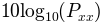for decibels, though Pxx itself is returned.

References:
Bendat & Piersol – Random Data: Analysis and Measurement Procedures, John Wiley & Sons (1986)

kwargs control the Line2D properties:

Property Description
agg_filter unknown
alpha float (0.0 transparent through 1.0 opaque)
animated [True | False]
antialiased or aa [True | False]
axes an Axes instance
clip_box a matplotlib.transforms.Bbox instance
clip_on [True | False]
clip_path [ (Path, Transform) | Patch | None ]
color or c any matplotlib color
contains a callable function
dash_capstyle [‘butt’ | ‘round’ | ‘projecting’]
dash_joinstyle [‘miter’ | ‘round’ | ‘bevel’]
dashes sequence of on/off ink in points
drawstyle [‘default’ | ‘steps’ | ‘steps-pre’ | ‘steps-mid’ | ‘steps-post’]
figure a matplotlib.figure.Figure instance
fillstyle [‘full’ | ‘left’ | ‘right’ | ‘bottom’ | ‘top’ | ‘none’]
gid an id string
label string or anything printable with ‘%s’ conversion.
linestyle or ls ['-' | '--' | '-.' | ':' | 'None' | ' ' | ''] and any drawstyle in combination with a linestyle, e.g., 'steps--'.
linewidth or lw float value in points
lod [True | False]
marker unknown
markeredgecolor or mec any matplotlib color
markeredgewidth or mew float value in points
markerfacecolor or mfc any matplotlib color
markerfacecoloralt or mfcalt any matplotlib color
markersize or ms float
markevery None | integer | (startind, stride)
path_effects unknown
picker float distance in points or callable pick function fn(artist, event)
rasterized [True | False | None]
sketch_params unknown
snap unknown
solid_capstyle [‘butt’ | ‘round’ | ‘projecting’]
solid_joinstyle [‘miter’ | ‘round’ | ‘bevel’]
transform a matplotlib.transforms.Transform instance
url a url string
visible [True | False]
xdata 1D array
ydata 1D array
zorder any number

Example: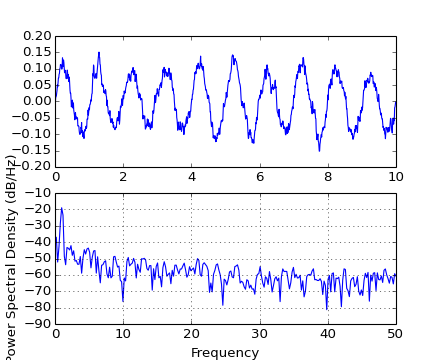Additional kwargs: hold = [True|False] overrides default hold state

matplotlib.pyplot.quiver(*args, **kw)

Plot a 2-D field of arrows.

call signatures:

```quiver(U, V, **kw)
quiver(U, V, C, **kw)
quiver(X, Y, U, V, **kw)
quiver(X, Y, U, V, C, **kw)
```

Arguments:

X, Y:
The x and y coordinates of the arrow locations (default is tail of arrow; see pivot kwarg)
U, V:
Give the x and y components of the arrow vectors
C:
An optional array used to map colors to the arrows

All arguments may be 1-D or 2-D arrays or sequences. If X and Y are absent, they will be generated as a uniform grid. If U and V are 2-D arrays but X and Y are 1-D, and if len(X) and len(Y) match the column and row dimensions of U, then X and Y will be expanded with numpy.meshgrid().

U, V, C may be masked arrays, but masked X, Y are not supported at present.

Keyword arguments:

units: [ ‘width’ | ‘height’ | ‘dots’ | ‘inches’ | ‘x’ | ‘y’ | ‘xy’ ]

Arrow units; the arrow dimensions except for length are in multiples of this unit.

• ‘width’ or ‘height’: the width or height of the axes
• ‘dots’ or ‘inches’: pixels or inches, based on the figure dpi
• ‘x’, ‘y’, or ‘xy’: X, Y, or sqrt(X^2+Y^2) data units

The arrows scale differently depending on the units. For ‘x’ or ‘y’, the arrows get larger as one zooms in; for other units, the arrow size is independent of the zoom state. For ‘width or ‘height’, the arrow size increases with the width and height of the axes, respectively, when the the window is resized; for ‘dots’ or ‘inches’, resizing does not change the arrows.

angles: [ ‘uv’ | ‘xy’ | array ]
With the default ‘uv’, the arrow aspect ratio is 1, so that if U*==*V the angle of the arrow on the plot is 45 degrees CCW from the x-axis. With ‘xy’, the arrow points from (x,y) to (x+u, y+v). Alternatively, arbitrary angles may be specified as an array of values in degrees, CCW from the x-axis.
scale: [ None | float ]
Data units per arrow length unit, e.g., m/s per plot width; a smaller scale parameter makes the arrow longer. If None, a simple autoscaling algorithm is used, based on the average vector length and the number of vectors. The arrow length unit is given by the scale_units parameter
scale_units: None, or any of the units options.

For example, if scale_units is ‘inches’, scale is 2.0, and (u,v) = (1,0), then the vector will be 0.5 inches long. If scale_units is ‘width’, then the vector will be half the width of the axes.

If scale_units is ‘x’ then the vector will be 0.5 x-axis units. To plot vectors in the x-y plane, with u and v having the same units as x and y, use “angles=’xy’, scale_units=’xy’, scale=1”.

width:
Shaft width in arrow units; default depends on choice of units, above, and number of vectors; a typical starting value is about 0.005 times the width of the plot.
Head width as multiple of shaft width, default is 3
Head length as multiple of shaft width, default is 5
Head length at shaft intersection, default is 4.5
minshaft: scalar
Length below which arrow scales, in units of head length. Do not set this to less than 1, or small arrows will look terrible! Default is 1
minlength: scalar
Minimum length as a multiple of shaft width; if an arrow length is less than this, plot a dot (hexagon) of this diameter instead. Default is 1.
pivot: [ ‘tail’ | ‘middle’ | ‘tip’ ]
The part of the arrow that is at the grid point; the arrow rotates about this point, hence the name pivot.
color: [ color | color sequence ]
This is a synonym for the PolyCollection facecolor kwarg. If C has been set, color has no effect.

The defaults give a slightly swept-back arrow; to make the head a triangle, make headaxislength the same as headlength. To make the arrow more pointed, reduce headwidth or increase headlength and headaxislength. To make the head smaller relative to the shaft, scale down all the head parameters. You will probably do best to leave minshaft alone.

linewidths and edgecolors can be used to customize the arrow outlines. Additional PolyCollection keyword arguments:

Property Description
agg_filter unknown
alpha float or None
animated [True | False]
antialiased or antialiaseds Boolean or sequence of booleans
array unknown
axes an Axes instance
clim a length 2 sequence of floats
clip_box a matplotlib.transforms.Bbox instance
clip_on [True | False]
clip_path [ (Path, Transform) | Patch | None ]
cmap a colormap or registered colormap name
color matplotlib color arg or sequence of rgba tuples
contains a callable function
edgecolor or edgecolors matplotlib color arg or sequence of rgba tuples
facecolor or facecolors matplotlib color arg or sequence of rgba tuples
figure a matplotlib.figure.Figure instance
gid an id string
hatch [ ‘/’ | ‘\’ | ‘|’ | ‘-‘ | ‘+’ | ‘x’ | ‘o’ | ‘O’ | ‘.’ | ‘*’ ]
label string or anything printable with ‘%s’ conversion.
linestyle or linestyles or dashes [‘solid’ | ‘dashed’, ‘dashdot’, ‘dotted’ | (offset, on-off-dash-seq) ]
linewidth or lw or linewidths float or sequence of floats
lod [True | False]
norm unknown
offset_position unknown
offsets float or sequence of floats
path_effects unknown
picker [None|float|boolean|callable]
rasterized [True | False | None]
sketch_params unknown
snap unknown
transform Transform instance
url a url string
urls unknown
visible [True | False]
zorder any number

Additional kwargs: hold = [True|False] overrides default hold state

matplotlib.pyplot.quiverkey(*args, **kw)

Add a key to a quiver plot.

Call signature:

```quiverkey(Q, X, Y, U, label, **kw)
```

Arguments:

Q:
The Quiver instance returned by a call to quiver.
X, Y:
The location of the key; additional explanation follows.
U:
The length of the key
label:
A string with the length and units of the key

Keyword arguments:

coordinates = [ ‘axes’ | ‘figure’ | ‘data’ | ‘inches’ ]
Coordinate system and units for X, Y: ‘axes’ and ‘figure’ are normalized coordinate systems with 0,0 in the lower left and 1,1 in the upper right; ‘data’ are the axes data coordinates (used for the locations of the vectors in the quiver plot itself); ‘inches’ is position in the figure in inches, with 0,0 at the lower left corner.
color:
overrides face and edge colors from Q.
labelpos = [ ‘N’ | ‘S’ | ‘E’ | ‘W’ ]
Position the label above, below, to the right, to the left of the arrow, respectively.
labelsep:
Distance in inches between the arrow and the label. Default is 0.1
labelcolor:
defaults to default Text color.
fontproperties:
A dictionary with keyword arguments accepted by the FontProperties initializer: family, style, variant, size, weight

Any additional keyword arguments are used to override vector properties taken from Q.

The positioning of the key depends on X, Y, coordinates, and labelpos. If labelpos is ‘N’ or ‘S’, X, Y give the position of the middle of the key arrow. If labelpos is ‘E’, X, Y positions the head, and if labelpos is ‘W’, X, Y positions the tail; in either of these two cases, X, Y is somewhere in the middle of the arrow+label key object.

Additional kwargs: hold = [True|False] overrides default hold state

matplotlib.pyplot.rc(*args, **kwargs)

Set the current rc params. Group is the grouping for the rc, e.g., for lines.linewidth the group is lines, for axes.facecolor, the group is axes, and so on. Group may also be a list or tuple of group names, e.g., (xtick, ytick). kwargs is a dictionary attribute name/value pairs, eg:

```rc('lines', linewidth=2, color='r')
```

sets the current rc params and is equivalent to:

```rcParams['lines.linewidth'] = 2
rcParams['lines.color'] = 'r'
```

The following aliases are available to save typing for interactive users:

Alias Property
‘lw’ ‘linewidth’
‘ls’ ‘linestyle’
‘c’ ‘color’
‘fc’ ‘facecolor’
‘ec’ ‘edgecolor’
‘mew’ ‘markeredgewidth’
‘aa’ ‘antialiased’

Thus you could abbreviate the above rc command as:

```rc('lines', lw=2, c='r')
```

Note you can use python’s kwargs dictionary facility to store dictionaries of default parameters. e.g., you can customize the font rc as follows:

```font = {'family' : 'monospace',
'weight' : 'bold',
'size'   : 'larger'}

rc('font', **font)  # pass in the font dict as kwargs
```

This enables you to easily switch between several configurations. Use rcdefaults() to restore the default rc params after changes.

matplotlib.pyplot.rc_context(rc=None, fname=None)

Return a context manager for managing rc settings.

This allows one to do:

```with mpl.rc_context(fname='screen.rc'):
plt.plot(x, a)
with mpl.rc_context(fname='print.rc'):
plt.plot(x, b)
plt.plot(x, c)
```

The ‘a’ vs ‘x’ and ‘c’ vs ‘x’ plots would have settings from ‘screen.rc’, while the ‘b’ vs ‘x’ plot would have settings from ‘print.rc’.

A dictionary can also be passed to the context manager:

```with mpl.rc_context(rc={'text.usetex': True}, fname='screen.rc'):
plt.plot(x, a)
```

The ‘rc’ dictionary takes precedence over the settings loaded from ‘fname’. Passing a dictionary only is also valid.

matplotlib.pyplot.rcdefaults()

Restore the default rc params. These are not the params loaded by the rc file, but mpl’s internal params. See rc_file_defaults for reloading the default params from the rc file

matplotlib.pyplot.rgrids(*args, **kwargs)

Get or set the radial gridlines on a polar plot.

call signatures:

```lines, labels = rgrids()
lines, labels = rgrids(radii, labels=None, angle=22.5, **kwargs)
```

When called with no arguments, rgrid() simply returns the tuple (lines, labels), where lines is an array of radial gridlines (Line2D instances) and labels is an array of tick labels (Text instances). When called with arguments, the labels will appear at the specified radial distances and angles.

labels, if not None, is a len(radii) list of strings of the labels to use at each angle.

If labels is None, the rformatter will be used

Examples:

```# set the locations of the radial gridlines and labels
lines, labels = rgrids( (0.25, 0.5, 1.0) )

# set the locations and labels of the radial gridlines and labels
lines, labels = rgrids( (0.25, 0.5, 1.0), ('Tom', 'Dick', 'Harry' )```
matplotlib.pyplot.savefig(*args, **kwargs)

Save the current figure.

Call signature:

```savefig(fname, dpi=None, facecolor='w', edgecolor='w',
orientation='portrait', papertype=None, format=None,
frameon=None)
```

The output formats available depend on the backend being used.

Arguments:

fname:

A string containing a path to a filename, or a Python file-like object, or possibly some backend-dependent object such as PdfPages.

If format is None and fname is a string, the output format is deduced from the extension of the filename. If the filename has no extension, the value of the rc parameter savefig.format is used.

If fname is not a string, remember to specify format to ensure that the correct backend is used.

Keyword arguments:

dpi: [ None | scalar > 0 ]
The resolution in dots per inch. If None it will default to the value savefig.dpi in the matplotlibrc file.
facecolor, edgecolor:
the colors of the figure rectangle
orientation: [ ‘landscape’ | ‘portrait’ ]
not supported on all backends; currently only on postscript output
papertype:
One of ‘letter’, ‘legal’, ‘executive’, ‘ledger’, ‘a0’ through ‘a10’, ‘b0’ through ‘b10’. Only supported for postscript output.
format:
One of the file extensions supported by the active backend. Most backends support png, pdf, ps, eps and svg.
transparent:
If True, the axes patches will all be transparent; the figure patch will also be transparent unless facecolor and/or edgecolor are specified via kwargs. This is useful, for example, for displaying a plot on top of a colored background on a web page. The transparency of these patches will be restored to their original values upon exit of this function.
frameon:
If True, the figure patch will be colored, if False, the figure background will be transparent. If not provided, the rcParam ‘savefig.frameon’ will be used.
bbox_inches:
Bbox in inches. Only the given portion of the figure is saved. If ‘tight’, try to figure out the tight bbox of the figure.
Amount of padding around the figure when bbox_inches is ‘tight’.
bbox_extra_artists:
A list of extra artists that will be considered when the tight bbox is calculated.
matplotlib.pyplot.sca(ax)

Set the current Axes instance to ax.

The current Figure is updated to the parent of ax.

matplotlib.pyplot.scatter(x, y, s=20, c='b', marker='o', cmap=None, norm=None, vmin=None, vmax=None, alpha=None, linewidths=None, verts=None, hold=None, **kwargs)

Make a scatter plot of x vs y, where x and y are sequence like objects of the same lengths.

Parameters: Returns: x, y : array_like, shape (n, ) Input data s : scalar or array_like, shape (n, ), optional, default: 20 size in points^2. c : color or sequence of color, optional, default c can be a single color format string, or a sequence of color specifications of length N, or a sequence of N numbers to be mapped to colors using the cmap and norm specified via kwargs (see below). Note that c should not be a single numeric RGB or RGBA sequence because that is indistinguishable from an array of values to be colormapped. c can be a 2-D array in which the rows are RGB or RGBA, however. marker : MarkerStyle, optional, default: ‘o’ See markers for more information on the different styles of markers scatter supports. cmap : Colormap, optional, default: None A Colormap instance or registered name. cmap is only used if c is an array of floats. If None, defaults to rc image.cmap. norm : Normalize, optional, default: None A Normalize instance is used to scale luminance data to 0, 1. norm is only used if c is an array of floats. If None, use the default normalize(). vmin, vmax : scalar, optional, default: None vmin and vmax are used in conjunction with norm to normalize luminance data. If either are None, the min and max of the color array is used. Note if you pass a norm instance, your settings for vmin and vmax will be ignored. alpha : scalar, optional, default: None The alpha blending value, between 0 (transparent) and 1 (opaque) linewidths : scalar or array_like, optional, default: None If None, defaults to (lines.linewidth,). Note that this is a tuple, and if you set the linewidths argument you must set it as a sequence of floats, as required by RegularPolyCollection. paths : PathCollection kwargs : Collection properties

Notes

Any or all of x, y, s, and c may be masked arrays, in which case all masks will be combined and only unmasked points will be plotted.

Examples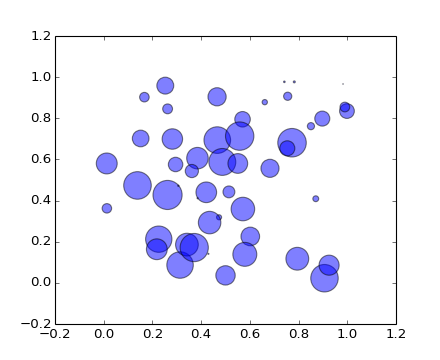Additional kwargs: hold = [True|False] overrides default hold state

matplotlib.pyplot.sci(im)

Set the current image. This image will be the target of colormap commands like jet(), hot() or clim()). The current image is an attribute of the current axes.

matplotlib.pyplot.semilogx(*args, **kwargs)

Make a plot with log scaling on the x axis.

Call signature:

```semilogx(*args, **kwargs)
```

semilogx() supports all the keyword arguments of plot() and matplotlib.axes.Axes.set_xscale().

Notable keyword arguments:

basex: scalar > 1
Base of the x logarithm
subsx: [ None | sequence ]
The location of the minor xticks; None defaults to autosubs, which depend on the number of decades in the plot; see set_xscale() for details.
nonposx: [ ‘mask’ | ‘clip’ ]
Non-positive values in x can be masked as invalid, or clipped to a very small positive number

The remaining valid kwargs are Line2D properties:

Property Description
agg_filter unknown
alpha float (0.0 transparent through 1.0 opaque)
animated [True | False]
antialiased or aa [True | False]
axes an Axes instance
clip_box a matplotlib.transforms.Bbox instance
clip_on [True | False]
clip_path [ (Path, Transform) | Patch | None ]
color or c any matplotlib color
contains a callable function
dash_capstyle [‘butt’ | ‘round’ | ‘projecting’]
dash_joinstyle [‘miter’ | ‘round’ | ‘bevel’]
dashes sequence of on/off ink in points
drawstyle [‘default’ | ‘steps’ | ‘steps-pre’ | ‘steps-mid’ | ‘steps-post’]
figure a matplotlib.figure.Figure instance
fillstyle [‘full’ | ‘left’ | ‘right’ | ‘bottom’ | ‘top’ | ‘none’]
gid an id string
label string or anything printable with ‘%s’ conversion.
linestyle or ls ['-' | '--' | '-.' | ':' | 'None' | ' ' | ''] and any drawstyle in combination with a linestyle, e.g., 'steps--'.
linewidth or lw float value in points
lod [True | False]
marker unknown
markeredgecolor or mec any matplotlib color
markeredgewidth or mew float value in points
markerfacecolor or mfc any matplotlib color
markerfacecoloralt or mfcalt any matplotlib color
markersize or ms float
markevery None | integer | (startind, stride)
path_effects unknown
picker float distance in points or callable pick function fn(artist, event)
rasterized [True | False | None]
sketch_params unknown
snap unknown
solid_capstyle [‘butt’ | ‘round’ | ‘projecting’]
solid_joinstyle [‘miter’ | ‘round’ | ‘bevel’]
transform a matplotlib.transforms.Transform instance
url a url string
visible [True | False]
xdata 1D array
ydata 1D array
zorder any number

loglog()
For example code and figure

Additional kwargs: hold = [True|False] overrides default hold state

matplotlib.pyplot.semilogy(*args, **kwargs)

Make a plot with log scaling on the y axis.

call signature:

```semilogy(*args, **kwargs)
```

semilogy() supports all the keyword arguments of plot() and matplotlib.axes.Axes.set_yscale().

Notable keyword arguments:

basey: scalar > 1
Base of the y logarithm
subsy: [ None | sequence ]
The location of the minor yticks; None defaults to autosubs, which depend on the number of decades in the plot; see set_yscale() for details.
nonposy: [ ‘mask’ | ‘clip’ ]
Non-positive values in y can be masked as invalid, or clipped to a very small positive number

The remaining valid kwargs are Line2D properties:

Property Description
agg_filter unknown
alpha float (0.0 transparent through 1.0 opaque)
animated [True | False]
antialiased or aa [True | False]
axes an Axes instance
clip_box a matplotlib.transforms.Bbox instance
clip_on [True | False]
clip_path [ (Path, Transform) | Patch | None ]
color or c any matplotlib color
contains a callable function
dash_capstyle [‘butt’ | ‘round’ | ‘projecting’]
dash_joinstyle [‘miter’ | ‘round’ | ‘bevel’]
dashes sequence of on/off ink in points
drawstyle [‘default’ | ‘steps’ | ‘steps-pre’ | ‘steps-mid’ | ‘steps-post’]
figure a matplotlib.figure.Figure instance
fillstyle [‘full’ | ‘left’ | ‘right’ | ‘bottom’ | ‘top’ | ‘none’]
gid an id string
label string or anything printable with ‘%s’ conversion.
linestyle or ls ['-' | '--' | '-.' | ':' | 'None' | ' ' | ''] and any drawstyle in combination with a linestyle, e.g., 'steps--'.
linewidth or lw float value in points
lod [True | False]
marker unknown
markeredgecolor or mec any matplotlib color
markeredgewidth or mew float value in points
markerfacecolor or mfc any matplotlib color
markerfacecoloralt or mfcalt any matplotlib color
markersize or ms float
markevery None | integer | (startind, stride)
path_effects unknown
picker float distance in points or callable pick function fn(artist, event)
rasterized [True | False | None]
sketch_params unknown
snap unknown
solid_capstyle [‘butt’ | ‘round’ | ‘projecting’]
solid_joinstyle [‘miter’ | ‘round’ | ‘bevel’]
transform a matplotlib.transforms.Transform instance
url a url string
visible [True | False]
xdata 1D array
ydata 1D array
zorder any number

loglog()
For example code and figure

Additional kwargs: hold = [True|False] overrides default hold state

matplotlib.pyplot.set_cmap(cmap)

Set the default colormap. Applies to the current image if any. See help(colormaps) for more information.

cmap must be a Colormap instance, or the name of a registered colormap.

matplotlib.pyplot.setp(*args, **kwargs)

Set a property on an artist object.

matplotlib supports the use of setp() (“set property”) and getp() to set and get object properties, as well as to do introspection on the object. For example, to set the linestyle of a line to be dashed, you can do:

```>>> line, = plot([1,2,3])
>>> setp(line, linestyle='--')
```

If you want to know the valid types of arguments, you can provide the name of the property you want to set without a value:

```>>> setp(line, 'linestyle')
linestyle: [ '-' | '--' | '-.' | ':' | 'steps' | 'None' ]
```

If you want to see all the properties that can be set, and their possible values, you can do:

```>>> setp(line)
... long output listing omitted
```

setp() operates on a single instance or a list of instances. If you are in query mode introspecting the possible values, only the first instance in the sequence is used. When actually setting values, all the instances will be set. e.g., suppose you have a list of two lines, the following will make both lines thicker and red:

```>>> x = arange(0,1.0,0.01)
>>> y1 = sin(2*pi*x)
>>> y2 = sin(4*pi*x)
>>> lines = plot(x, y1, x, y2)
>>> setp(lines, linewidth=2, color='r')
```

setp() works with the MATLAB style string/value pairs or with python kwargs. For example, the following are equivalent:

```>>> setp(lines, 'linewidth', 2, 'color', 'r')  # MATLAB style
>>> setp(lines, linewidth=2, color='r')        # python style
```
matplotlib.pyplot.show(*args, **kw)

Display a figure.

When running in ipython with its pylab mode, display all figures and return to the ipython prompt.

In non-interactive mode, display all figures and block until the figures have been closed; in interactive mode it has no effect unless figures were created prior to a change from non-interactive to interactive mode (not recommended). In that case it displays the figures but does not block.

A single experimental keyword argument, block, may be set to True or False to override the blocking behavior described above.

matplotlib.pyplot.specgram(x, NFFT=256, Fs=2, Fc=0, detrend=<function detrend_none at 0x2635de8>, window=<function window_hanning at 0x2635b90>, noverlap=128, cmap=None, xextent=None, pad_to=None, sides='default', scale_by_freq=None, hold=None, **kwargs)

Plot a spectrogram.

Call signature:

```specgram(x, NFFT=256, Fs=2, Fc=0, detrend=mlab.detrend_none,
window=mlab.window_hanning, noverlap=128,
scale_by_freq=None, **kwargs)
```

Compute and plot a spectrogram of data in x. Data are split into NFFT length segments and the PSD of each section is computed. The windowing function window is applied to each segment, and the amount of overlap of each segment is specified with noverlap. The spectrogram is plotted in decibels as a colormap (using imshow).

Keyword arguments:

NFFT: integer
The number of data points used in each block for the FFT. Must be even; a power 2 is most efficient. The default value is 256. This should NOT be used to get zero padding, or the scaling of the result will be incorrect. Use pad_to for this instead.
Fs: scalar
The sampling frequency (samples per time unit). It is used to calculate the Fourier frequencies, freqs, in cycles per time unit. The default value is 2.
detrend: callable
The function applied to each segment before fft-ing, designed to remove the mean or linear trend. Unlike in MATLAB, where the detrend parameter is a vector, in matplotlib is it a function. The pylab module defines detrend_none(), detrend_mean(), and detrend_linear(), but you can use a custom function as well.
window: callable or ndarray
A function or a vector of length NFFT. To create window vectors see window_hanning(), window_none(), numpy.blackman(), numpy.hamming(), numpy.bartlett(), scipy.signal(), scipy.signal.get_window(), etc. The default is window_hanning(). If a function is passed as the argument, it must take a data segment as an argument and return the windowed version of the segment.
The number of points to which the data segment is padded when performing the FFT. This can be different from NFFT, which specifies the number of data points used. While not increasing the actual resolution of the psd (the minimum distance between resolvable peaks), this can give more points in the plot, allowing for more detail. This corresponds to the n parameter in the call to fft(). The default is None, which sets pad_to equal to NFFT
sides: [ ‘default’ | ‘onesided’ | ‘twosided’ ]
Specifies which sides of the PSD to return. Default gives the default behavior, which returns one-sided for real data and both for complex data. ‘onesided’ forces the return of a one-sided PSD, while ‘twosided’ forces two-sided.
scale_by_freq: boolean
Specifies whether the resulting density values should be scaled by the scaling frequency, which gives density in units of Hz^-1. This allows for integration over the returned frequency values. The default is True for MATLAB compatibility.
noverlap: integer
The number of points of overlap between blocks. The default value is 128.
Fc: integer
The center frequency of x (defaults to 0), which offsets the y extents of the plot to reflect the frequency range used when a signal is acquired and then filtered and downsampled to baseband.
cmap:
A matplotlib.colors.Colormap instance; if None, use default determined by rc
xextent:
The image extent along the x-axis. xextent = (xmin,xmax) The default is (0,max(bins)), where bins is the return value from specgram()

kwargs:

Additional kwargs are passed on to imshow which makes the specgram image

Return value is (Pxx, freqs, bins, im):

• bins are the time points the spectrogram is calculated over
• freqs is an array of frequencies
• Pxx is an array of shape (len(times), len(freqs)) of power
• im is a AxesImage instance

Note

If x is real (i.e. non-complex), only the positive spectrum is shown. If x is complex, both positive and negative parts of the spectrum are shown. This can be overridden using the sides keyword argument.

Also note that while the plot is in dB, the Pxx array returned is linear in power.

Example: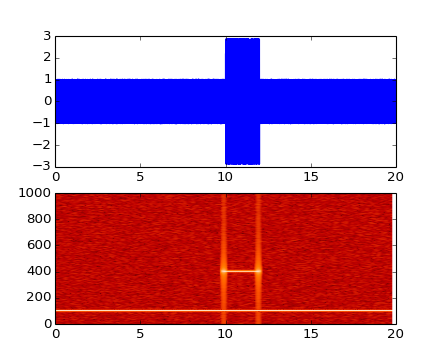Additional kwargs: hold = [True|False] overrides default hold state

matplotlib.pyplot.spectral()

set the default colormap to spectral and apply to current image if any. See help(colormaps) for more information

matplotlib.pyplot.spring()

set the default colormap to spring and apply to current image if any. See help(colormaps) for more information

matplotlib.pyplot.spy(Z, precision=0, marker=None, markersize=None, aspect='equal', hold=None, **kwargs)

Plot the sparsity pattern on a 2-D array.

Call signature:

```spy(Z, precision=0, marker=None, markersize=None,
aspect='equal', **kwargs)
```

spy(Z) plots the sparsity pattern of the 2-D array Z.

If precision is 0, any non-zero value will be plotted; else, values of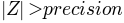will be plotted.

For scipy.sparse.spmatrix instances, there is a special case: if precision is ‘present’, any value present in the array will be plotted, even if it is identically zero.

The array will be plotted as it would be printed, with the first index (row) increasing down and the second index (column) increasing to the right.

By default aspect is ‘equal’, so that each array element occupies a square space; set the aspect kwarg to ‘auto’ to allow the plot to fill the plot box, or to any scalar number to specify the aspect ratio of an array element directly.

Two plotting styles are available: image or marker. Both are available for full arrays, but only the marker style works for scipy.sparse.spmatrix instances.

If marker and markersize are None, an image will be returned and any remaining kwargs are passed to imshow(); else, a Line2D object will be returned with the value of marker determining the marker type, and any remaining kwargs passed to the plot() method.

If marker and markersize are None, useful kwargs include:

• cmap
• alpha

imshow()
For image options.

For controlling colors, e.g., cyan background and red marks, use:

```cmap = mcolors.ListedColormap(['c','r'])
```

If marker or markersize is not None, useful kwargs include:

• marker
• markersize
• color

Useful values for marker include:

• ‘s’ square (default)
• ‘o’ circle
• ‘.’ point
• ‘,’ pixel

plot()
For plotting options

Additional kwargs: hold = [True|False] overrides default hold state

matplotlib.pyplot.stackplot(x, *args, **kwargs)

Draws a stacked area plot.

x : 1d array of dimension N

y : 2d array of dimension MxN, OR any number 1d arrays each of dimension

1xN. The data is assumed to be unstacked. Each of the following calls is legal:

```stackplot(x, y)               # where y is MxN
stackplot(x, y1, y2, y3, y4)  # where y1, y2, y3, y4, are all 1xNm
```

Keyword arguments:

baseline : [‘zero’, ‘sym’, ‘wiggle’, ‘weighted_wiggle’]
Method used to calculate the baseline. ‘zero’ is just a simple stacked plot. ‘sym’ is symmetric around zero and is sometimes called ThemeRiver. ‘wiggle’ minimizes the sum of the squared slopes. ‘weighted_wiggle’ does the same but weights to account for size of each layer. It is also called Streamgraph-layout. More details can be found at http://www.leebyron.com/else/streamgraph/.
colors : A list or tuple of colors. These will be cycled through and
used to colour the stacked areas. All other keyword arguments are passed to fill_between()

Returns r : A list of PolyCollection, one for each element in the stacked area plot.

Note that Legend does not support PolyCollection objects. To create a legend on a stackplot, use a proxy artist: http://matplotlib.org/users/legend_guide.html#using-proxy-artist

Additional kwargs: hold = [True|False] overrides default hold state

matplotlib.pyplot.stem(*args, **kwargs)

Create a stem plot.

Call signatures:

```stem(y, linefmt='b-', markerfmt='bo', basefmt='r-')
stem(x, y, linefmt='b-', markerfmt='bo', basefmt='r-')
```

A stem plot plots vertical lines (using linefmt) at each x location from the baseline to y, and places a marker there using markerfmt. A horizontal line at 0 is is plotted using basefmt.

If no x values are provided, the default is (0, 1, ..., len(y) - 1)

Return value is a tuple (markerline, stemlines, baseline).

This document for details.

Example: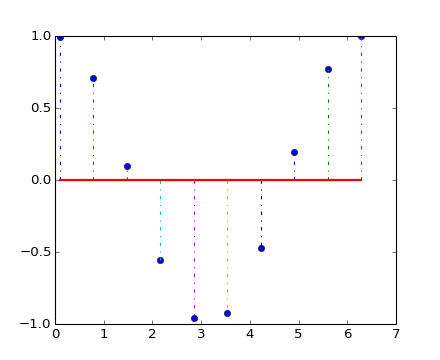Additional kwargs: hold = [True|False] overrides default hold state

matplotlib.pyplot.step(x, y, *args, **kwargs)

Make a step plot.

Call signature:

```step(x, y, *args, **kwargs)
```

Additional keyword args to step() are the same as those for plot().

x and y must be 1-D sequences, and it is assumed, but not checked, that x is uniformly increasing.

Keyword arguments:

where: [ ‘pre’ | ‘post’ | ‘mid’ ]

If ‘pre’, the interval from x[i] to x[i+1] has level y[i+1]

If ‘post’, that interval has level y[i]

If ‘mid’, the jumps in y occur half-way between the x-values.

Additional kwargs: hold = [True|False] overrides default hold state

matplotlib.pyplot.streamplot(x, y, u, v, density=1, linewidth=None, color=None, cmap=None, norm=None, arrowsize=1, arrowstyle='-|>', minlength=0.1, transform=None, hold=None)

Draws streamlines of a vector flow.

x, y : 1d arrays
an evenly spaced grid.
u, v : 2d arrays
x and y-velocities. Number of rows should match length of y, and the number of columns should match x.
density : float or 2-tuple
Controls the closeness of streamlines. When density = 1, the domain is divided into a 25x25 grid—density linearly scales this grid. Each cell in the grid can have, at most, one traversing streamline. For different densities in each direction, use [density_x, density_y].
linewidth : numeric or 2d array
vary linewidth when given a 2d array with the same shape as velocities.
color : matplotlib color code, or 2d array
Streamline color. When given an array with the same shape as velocities, color values are converted to colors using cmap.
cmap : Colormap
Colormap used to plot streamlines and arrows. Only necessary when using an array input for color.
norm : Normalize
Normalize object used to scale luminance data to 0, 1. If None, stretch (min, max) to (0, 1). Only necessary when color is an array.
arrowsize : float
Factor scale arrow size.
arrowstyle : str
Arrow style specification. See FancyArrowPatch.
minlength : float
Minimum length of streamline in axes coordinates.

Returns:

stream_container : StreamplotSet

Container object with attributes

This container will probably change in the future to allow changes to the colormap, alpha, etc. for both lines and arrows, but these changes should be backward compatible.

Additional kwargs: hold = [True|False] overrides default hold state

matplotlib.pyplot.subplot(*args, **kwargs)

Return a subplot axes positioned by the given grid definition.

Typical call signature:

```subplot(nrows, ncols, plot_number)
```

Where nrows and ncols are used to notionally split the figure into nrows * ncols sub-axes, and plot_number is used to identify the particular subplot that this function is to create within the notional grid. plot_number starts at 1, increments across rows first and has a maximum of nrows * ncols.

In the case when nrows, ncols and plot_number are all less than 10, a convenience exists, such that the a 3 digit number can be given instead, where the hundreds represent nrows, the tens represent ncols and the units represent plot_number. For instance:

```subplot(211)
```

produces a subaxes in a figure which represents the top plot (i.e. the first) in a 2 row by 1 column notional grid (no grid actually exists, but conceptually this is how the returned subplot has been positioned).

Note

Creating a new subplot with a position which is entirely inside a pre-existing axes will trigger the larger axes to be deleted:

```import matplotlib.pyplot as plt
# plot a line, implicitly creating a subplot(111)
plt.plot([1,2,3])
# now create a subplot which represents the top plot of a grid
# with 2 rows and 1 column. Since this subplot will overlap the
# first, the plot (and its axes) previously created, will be removed
plt.subplot(211)
plt.plot(range(12))
plt.subplot(212, axisbg='y') # creates 2nd subplot with yellow background
```

If you do not want this behavior, use the add_subplot() method or the axes() function instead.

Keyword arguments:

axisbg:
The background color of the subplot, which can be any valid color specifier. See matplotlib.colors for more information.
polar:
A boolean flag indicating whether the subplot plot should be a polar projection. Defaults to False.
projection:
A string giving the name of a custom projection to be used for the subplot. This projection must have been previously registered. See matplotlib.projections.

axes()
For additional information on axes() and subplot() keyword arguments.
examples/pie_and_polar_charts/polar_scatter_demo.py
For an example

Example: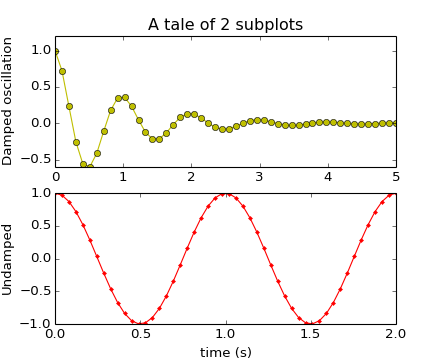matplotlib.pyplot.subplot2grid(shape, loc, rowspan=1, colspan=1, **kwargs)

Create a subplot in a grid. The grid is specified by shape, at location of loc, spanning rowspan, colspan cells in each direction. The index for loc is 0-based.

```subplot2grid(shape, loc, rowspan=1, colspan=1)
```

is identical to

```gridspec=GridSpec(shape, shape)
subplotspec=gridspec.new_subplotspec(loc, rowspan, colspan)
subplot(subplotspec)
```
matplotlib.pyplot.subplot_tool(targetfig=None)

Launch a subplot tool window for a figure.

A matplotlib.widgets.SubplotTool instance is returned.

matplotlib.pyplot.subplots(nrows=1, ncols=1, sharex=False, sharey=False, squeeze=True, subplot_kw=None, **fig_kw)

This utility wrapper makes it convenient to create common layouts of subplots, including the enclosing figure object, in a single call.

Keyword arguments:

nrows : int
Number of rows of the subplot grid. Defaults to 1.
ncols : int
Number of columns of the subplot grid. Defaults to 1.
sharex : string or bool
If True, the X axis will be shared amongst all subplots. If True and you have multiple rows, the x tick labels on all but the last row of plots will have visible set to False If a string must be one of “row”, “col”, “all”, or “none”. “all” has the same effect as True, “none” has the same effect as False. If “row”, each subplot row will share a X axis. If “col”, each subplot column will share a X axis and the x tick labels on all but the last row will have visible set to False.
sharey : string or bool
If True, the Y axis will be shared amongst all subplots. If True and you have multiple columns, the y tick labels on all but the first column of plots will have visible set to False If a string must be one of “row”, “col”, “all”, or “none”. “all” has the same effect as True, “none” has the same effect as False. If “row”, each subplot row will share a Y axis. If “col”, each subplot column will share a Y axis and the y tick labels on all but the last row will have visible set to False.
squeeze : bool

If True, extra dimensions are squeezed out from the returned axis object:

• if only one subplot is constructed (nrows=ncols=1), the resulting single Axis object is returned as a scalar.
• for Nx1 or 1xN subplots, the returned object is a 1-d numpy object array of Axis objects are returned as numpy 1-d arrays.
• for NxM subplots with N>1 and M>1 are returned as a 2d array.

If False, no squeezing at all is done: the returned axis object is always a 2-d array containing Axis instances, even if it ends up being 1x1.

subplot_kw : dict
Dict with keywords passed to the add_subplot() call used to create each subplots.
fig_kw : dict
Dict with keywords passed to the figure() call. Note that all keywords not recognized above will be automatically included here.

Returns:

fig, ax : tuple

• fig is the matplotlib.figure.Figure object
• ax can be either a single axis object or an array of axis objects if more than one subplot was created. The dimensions of the resulting array can be controlled with the squeeze keyword, see above.

Examples:

```x = np.linspace(0, 2*np.pi, 400)
y = np.sin(x**2)

# Just a figure and one subplot
f, ax = plt.subplots()
ax.plot(x, y)
ax.set_title('Simple plot')

# Two subplots, unpack the output array immediately
f, (ax1, ax2) = plt.subplots(1, 2, sharey=True)
ax1.plot(x, y)
ax1.set_title('Sharing Y axis')
ax2.scatter(x, y)

# Four polar axes
plt.subplots(2, 2, subplot_kw=dict(polar=True))

# Share a X axis with each column of subplots
plt.subplots(2, 2, sharex='col')

# Share a Y axis with each row of subplots
plt.subplots(2, 2, sharey='row')

# Share a X and Y axis with all subplots
plt.subplots(2, 2, sharex='all', sharey='all')
# same as
plt.subplots(2, 2, sharex=True, sharey=True)
```

Tune the subplot layout.

call signature:

```subplots_adjust(left=None, bottom=None, right=None, top=None,
wspace=None, hspace=None)
```

The parameter meanings (and suggested defaults) are:

```left  = 0.125  # the left side of the subplots of the figure
right = 0.9    # the right side of the subplots of the figure
bottom = 0.1   # the bottom of the subplots of the figure
top = 0.9      # the top of the subplots of the figure
wspace = 0.2   # the amount of width reserved for blank space between subplots
hspace = 0.2   # the amount of height reserved for white space between subplots
```

The actual defaults are controlled by the rc file

matplotlib.pyplot.summer()

set the default colormap to summer and apply to current image if any. See help(colormaps) for more information

matplotlib.pyplot.suptitle(*args, **kwargs)

Add a centered title to the figure.

kwargs are matplotlib.text.Text properties. Using figure coordinates, the defaults are:

x : 0.5
The x location of the text in figure coords
y : 0.98
The y location of the text in figure coords
horizontalalignment : ‘center’
The horizontal alignment of the text
verticalalignment : ‘top’
The vertical alignment of the text

A matplotlib.text.Text instance is returned.

Example:

```fig.suptitle('this is the figure title', fontsize=12)
```
matplotlib.pyplot.switch_backend(newbackend)

Switch the default backend. This feature is experimental, and is only expected to work switching to an image backend. e.g., if you have a bunch of PostScript scripts that you want to run from an interactive ipython session, you may want to switch to the PS backend before running them to avoid having a bunch of GUI windows popup. If you try to interactively switch from one GUI backend to another, you will explode.

Calling this command will close all open windows.

matplotlib.pyplot.table(**kwargs)

Add a table to the current axes.

Call signature:

```table(cellText=None, cellColours=None,
cellLoc='right', colWidths=None,
rowLabels=None, rowColours=None, rowLoc='left',
colLabels=None, colColours=None, colLoc='center',
loc='bottom', bbox=None):```

Returns a matplotlib.table.Table instance. For finer grained control over tables, use the Table class and add it to the axes with add_table().

Thanks to John Gill for providing the class and table.

kwargs control the Table properties:

Property Description
agg_filter unknown
alpha float (0.0 transparent through 1.0 opaque)
animated [True | False]
axes an Axes instance
clip_box a matplotlib.transforms.Bbox instance
clip_on [True | False]
clip_path [ (Path, Transform) | Patch | None ]
contains a callable function
figure a matplotlib.figure.Figure instance
fontsize a float in points
gid an id string
label string or anything printable with ‘%s’ conversion.
lod [True | False]
path_effects unknown
picker [None|float|boolean|callable]
rasterized [True | False | None]
sketch_params unknown
snap unknown
transform Transform instance
url a url string
visible [True | False]
zorder any number
matplotlib.pyplot.text(x, y, s, fontdict=None, withdash=False, **kwargs)

Add text in string s to axis at location x, y, data coordinates.

Parameters: Other Parameters: s : string text x, y : scalars data coordinates fontdict : dictionary, optional, default: None A dictionary to override the default text properties. If fontdict is None, the defaults are determined by your rc parameters. withdash : boolean, optional, default: False Creates a TextWithDash instance instead of a Text instance. kwargs : Text properties. Other miscellaneous text parameters.

Examples

Individual keyword arguments can be used to override any given parameter:

```>>> text(x, y, s, fontsize=12)
```

The default transform specifies that text is in data coords, alternatively, you can specify text in axis coords (0,0 is lower-left and 1,1 is upper-right). The example below places text in the center of the axes:

```>>> text(0.5, 0.5,'matplotlib', horizontalalignment='center',
...      verticalalignment='center',
...      transform=ax.transAxes)
```

You can put a rectangular box around the text instance (e.g., to set a background color) by using the keyword bbox. bbox is a dictionary of Rectangle properties. For example:

```>>> text(x, y, s, bbox=dict(facecolor='red', alpha=0.5))
```
matplotlib.pyplot.thetagrids(*args, **kwargs)

Get or set the theta locations of the gridlines in a polar plot.

If no arguments are passed, return a tuple (lines, labels) where lines is an array of radial gridlines (Line2D instances) and labels is an array of tick labels (Text instances):

```lines, labels = thetagrids()
```

Otherwise the syntax is:

```lines, labels = thetagrids(angles, labels=None, fmt='%d', frac = 1.1)
```

set the angles at which to place the theta grids (these gridlines are equal along the theta dimension).

angles is in degrees.

labels, if not None, is a len(angles) list of strings of the labels to use at each angle.

If labels is None, the labels will be fmt%angle.

frac is the fraction of the polar axes radius at which to place the label (1 is the edge). e.g., 1.05 is outside the axes and 0.95 is inside the axes.

Return value is a list of tuples (lines, labels):

• lines are Line2D instances
• labels are Text instances.

Note that on input, the labels argument is a list of strings, and on output it is a list of Text instances.

Examples:

```# set the locations of the radial gridlines and labels
lines, labels = thetagrids( range(45,360,90) )

# set the locations and labels of the radial gridlines and labels
lines, labels = thetagrids( range(45,360,90), ('NE', 'NW', 'SW','SE') )
```
matplotlib.pyplot.tick_params(axis='both', **kwargs)

Change the appearance of ticks and tick labels.

Keyword arguments:

axis : [‘x’ | ‘y’ | ‘both’]
Axis on which to operate; default is ‘both’.
reset : [True | False]
If True, set all parameters to defaults before processing other keyword arguments. Default is False.
which : [‘major’ | ‘minor’ | ‘both’]
Default is ‘major’; apply arguments to which ticks.
direction : [‘in’ | ‘out’ | ‘inout’]
Puts ticks inside the axes, outside the axes, or both.
length
Tick length in points.
width
Tick width in points.
color
Tick color; accepts any mpl color spec.
Distance in points between tick and label.
labelsize
Tick label font size in points or as a string (e.g., ‘large’).
labelcolor
Tick label color; mpl color spec.
colors
Changes the tick color and the label color to the same value: mpl color spec.
zorder
Tick and label zorder.
bottom, top, left, right : [bool | ‘on’ | ‘off’]
controls whether to draw the respective ticks.
labelbottom, labeltop, labelleft, labelright
Boolean or [‘on’ | ‘off’], controls whether to draw the respective tick labels.

Example:

```ax.tick_params(direction='out', length=6, width=2, colors='r')
```

This will make all major ticks be red, pointing out of the box, and with dimensions 6 points by 2 points. Tick labels will also be red.

matplotlib.pyplot.ticklabel_format(**kwargs)

Change the ScalarFormatter used by default for linear axes.

Optional keyword arguments:

Keyword Description
style [ ‘sci’ (or ‘scientific’) | ‘plain’ ] plain turns off scientific notation
scilimits (m, n), pair of integers; if style is ‘sci’, scientific notation will be used for numbers outside the range 10`m`:sup: to 10`n`:sup:. Use (0,0) to include all numbers.
useOffset [True | False | offset]; if True, the offset will be calculated as needed; if False, no offset will be used; if a numeric offset is specified, it will be used.
axis [ ‘x’ | ‘y’ | ‘both’ ]
useLocale If True, format the number according to the current locale. This affects things such as the character used for the decimal separator. If False, use C-style (English) formatting. The default setting is controlled by the axes.formatter.use_locale rcparam.

Only the major ticks are affected. If the method is called when the ScalarFormatter is not the Formatter being used, an AttributeError will be raised.

Parameters:

padding between the figure edge and the edges of subplots, as a fraction of the font-size.
rect : if rect is given, it is interpreted as a rectangle
(left, bottom, right, top) in the normalized figure coordinate that the whole subplots area (including labels) will fit into. Default is (0, 0, 1, 1).
matplotlib.pyplot.title(s, *args, **kwargs)

Set a title of the current axes.

Set one of the three available axes titles. The available titles are positioned above the axes in the center, flush with the left edge, and flush with the right edge.

Parameters: Returns: label : str Text to use for the title fontdict : dict A dictionary controlling the appearance of the title text, the default fontdict is: {‘fontsize’: rcParams[‘axes.titlesize’], ‘verticalalignment’: ‘baseline’, ‘horizontalalignment’: loc} loc : {‘center’, ‘left’, ‘right’}, str, optional Which title to set, defaults to ‘center’ text : Text The matplotlib text instance representing the title Other keyword arguments are text properties, see : :class:`~matplotlib.text.Text` for a list of valid text : properties. :

See
func:text for adding text to the current axes
matplotlib.pyplot.tricontour(*args, **kwargs)

Draw contours on an unstructured triangular grid. tricontour() and tricontourf() draw contour lines and filled contours, respectively. Except as noted, function signatures and return values are the same for both versions.

The triangulation can be specified in one of two ways; either:

```tricontour(triangulation, ...)
```

where triangulation is a matplotlib.tri.Triangulation object, or

```tricontour(x, y, ...)
tricontour(x, y, triangles, ...)
tricontour(x, y, triangles=triangles, ...)
```

in which case a Triangulation object will be created. See Triangulation for a explanation of these possibilities.

The remaining arguments may be:

```tricontour(..., Z)
```

where Z is the array of values to contour, one per point in the triangulation. The level values are chosen automatically.

```tricontour(..., Z, N)
```

contour N automatically-chosen levels.

```tricontour(..., Z, V)
```

draw contour lines at the values specified in sequence V

```tricontourf(..., Z, V)
```

fill the (len(V)-1) regions between the values in V

```tricontour(Z, **kwargs)
```

Use keyword args to control colors, linewidth, origin, cmap ... see below for more details.

C = tricontour(...) returns a TriContourSet object.

Optional keyword arguments:

colors: [ None | string | (mpl_colors) ]

If None, the colormap specified by cmap will be used.

If a string, like ‘r’ or ‘red’, all levels will be plotted in this color.

If a tuple of matplotlib color args (string, float, rgb, etc), different levels will be plotted in different colors in the order specified.

alpha: float
The alpha blending value
cmap: [ None | Colormap ]
A cm Colormap instance or None. If cmap is None and colors is None, a default Colormap is used.
norm: [ None | Normalize ]
A matplotlib.colors.Normalize instance for scaling data values to colors. If norm is None and colors is None, the default linear scaling is used.
levels [level0, level1, ..., leveln]
A list of floating point numbers indicating the level curves to draw; eg to draw just the zero contour pass levels=
origin: [ None | ‘upper’ | ‘lower’ | ‘image’ ]

If None, the first value of Z will correspond to the lower left corner, location (0,0). If ‘image’, the rc value for image.origin will be used.

This keyword is not active if X and Y are specified in the call to contour.

extent: [ None | (x0,x1,y0,y1) ]

If origin is not None, then extent is interpreted as in matplotlib.pyplot.imshow(): it gives the outer pixel boundaries. In this case, the position of Z[0,0] is the center of the pixel, not a corner. If origin is None, then (x0, y0) is the position of Z[0,0], and (x1, y1) is the position of Z[-1,-1].

This keyword is not active if X and Y are specified in the call to contour.

locator: [ None | ticker.Locator subclass ]
If locator is None, the default MaxNLocator is used. The locator is used to determine the contour levels if they are not given explicitly via the V argument.
extend: [ ‘neither’ | ‘both’ | ‘min’ | ‘max’ ]
Unless this is ‘neither’, contour levels are automatically added to one or both ends of the range so that all data are included. These added ranges are then mapped to the special colormap values which default to the ends of the colormap range, but can be set via matplotlib.colors.Colormap.set_under() and matplotlib.colors.Colormap.set_over() methods.
xunits, yunits: [ None | registered units ]
Override axis units by specifying an instance of a matplotlib.units.ConversionInterface.

tricontour-only keyword arguments:

linewidths: [ None | number | tuple of numbers ]

If linewidths is None, the default width in lines.linewidth in matplotlibrc is used.

If a number, all levels will be plotted with this linewidth.

If a tuple, different levels will be plotted with different linewidths in the order specified

linestyles: [ None | ‘solid’ | ‘dashed’ | ‘dashdot’ | ‘dotted’ ]

If linestyles is None, the ‘solid’ is used.

linestyles can also be an iterable of the above strings specifying a set of linestyles to be used. If this iterable is shorter than the number of contour levels it will be repeated as necessary.

If contour is using a monochrome colormap and the contour level is less than 0, then the linestyle specified in contour.negative_linestyle in matplotlibrc will be used.

tricontourf-only keyword arguments:

antialiased: [ True | False ]
enable antialiasing
nchunk: [ 0 | integer ]
If 0, no subdivision of the domain. Specify a positive integer to divide the domain into subdomains of roughly nchunk by nchunk points. This may never actually be advantageous, so this option may be removed. Chunking introduces artifacts at the chunk boundaries unless antialiased is False.

Note: tricontourf fills intervals that are closed at the top; that is, for boundaries z1 and z2, the filled region is:

```z1 < z <= z2
```

There is one exception: if the lowest boundary coincides with the minimum value of the z array, then that minimum value will be included in the lowest interval.

Examples: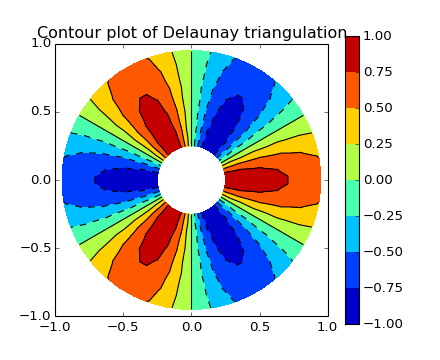(png, hires.png, pdf)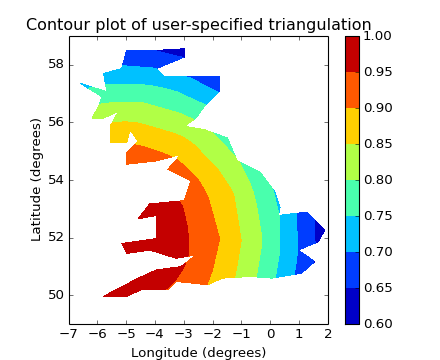(png, hires.png, pdf)

Additional kwargs: hold = [True|False] overrides default hold state

matplotlib.pyplot.tricontourf(*args, **kwargs)

Draw contours on an unstructured triangular grid. tricontour() and tricontourf() draw contour lines and filled contours, respectively. Except as noted, function signatures and return values are the same for both versions.

The triangulation can be specified in one of two ways; either:

```tricontour(triangulation, ...)
```

where triangulation is a matplotlib.tri.Triangulation object, or

```tricontour(x, y, ...)
tricontour(x, y, triangles, ...)
tricontour(x, y, triangles=triangles, ...)
```

in which case a Triangulation object will be created. See Triangulation for a explanation of these possibilities.

The remaining arguments may be:

```tricontour(..., Z)
```

where Z is the array of values to contour, one per point in the triangulation. The level values are chosen automatically.

```tricontour(..., Z, N)
```

contour N automatically-chosen levels.

```tricontour(..., Z, V)
```

draw contour lines at the values specified in sequence V

```tricontourf(..., Z, V)
```

fill the (len(V)-1) regions between the values in V

```tricontour(Z, **kwargs)
```

Use keyword args to control colors, linewidth, origin, cmap ... see below for more details.

C = tricontour(...) returns a TriContourSet object.

Optional keyword arguments:

colors: [ None | string | (mpl_colors) ]

If None, the colormap specified by cmap will be used.

If a string, like ‘r’ or ‘red’, all levels will be plotted in this color.

If a tuple of matplotlib color args (string, float, rgb, etc), different levels will be plotted in different colors in the order specified.

alpha: float
The alpha blending value
cmap: [ None | Colormap ]
A cm Colormap instance or None. If cmap is None and colors is None, a default Colormap is used.
norm: [ None | Normalize ]
A matplotlib.colors.Normalize instance for scaling data values to colors. If norm is None and colors is None, the default linear scaling is used.
levels [level0, level1, ..., leveln]
A list of floating point numbers indicating the level curves to draw; eg to draw just the zero contour pass levels=
origin: [ None | ‘upper’ | ‘lower’ | ‘image’ ]

If None, the first value of Z will correspond to the lower left corner, location (0,0). If ‘image’, the rc value for image.origin will be used.

This keyword is not active if X and Y are specified in the call to contour.

extent: [ None | (x0,x1,y0,y1) ]

If origin is not None, then extent is interpreted as in matplotlib.pyplot.imshow(): it gives the outer pixel boundaries. In this case, the position of Z[0,0] is the center of the pixel, not a corner. If origin is None, then (x0, y0) is the position of Z[0,0], and (x1, y1) is the position of Z[-1,-1].

This keyword is not active if X and Y are specified in the call to contour.

locator: [ None | ticker.Locator subclass ]
If locator is None, the default MaxNLocator is used. The locator is used to determine the contour levels if they are not given explicitly via the V argument.
extend: [ ‘neither’ | ‘both’ | ‘min’ | ‘max’ ]
Unless this is ‘neither’, contour levels are automatically added to one or both ends of the range so that all data are included. These added ranges are then mapped to the special colormap values which default to the ends of the colormap range, but can be set via matplotlib.colors.Colormap.set_under() and matplotlib.colors.Colormap.set_over() methods.
xunits, yunits: [ None | registered units ]
Override axis units by specifying an instance of a matplotlib.units.ConversionInterface.

tricontour-only keyword arguments:

linewidths: [ None | number | tuple of numbers ]

If linewidths is None, the default width in lines.linewidth in matplotlibrc is used.

If a number, all levels will be plotted with this linewidth.

If a tuple, different levels will be plotted with different linewidths in the order specified

linestyles: [ None | ‘solid’ | ‘dashed’ | ‘dashdot’ | ‘dotted’ ]

If linestyles is None, the ‘solid’ is used.

linestyles can also be an iterable of the above strings specifying a set of linestyles to be used. If this iterable is shorter than the number of contour levels it will be repeated as necessary.

If contour is using a monochrome colormap and the contour level is less than 0, then the linestyle specified in contour.negative_linestyle in matplotlibrc will be used.

tricontourf-only keyword arguments:

antialiased: [ True | False ]
enable antialiasing
nchunk: [ 0 | integer ]
If 0, no subdivision of the domain. Specify a positive integer to divide the domain into subdomains of roughly nchunk by nchunk points. This may never actually be advantageous, so this option may be removed. Chunking introduces artifacts at the chunk boundaries unless antialiased is False.

Note: tricontourf fills intervals that are closed at the top; that is, for boundaries z1 and z2, the filled region is:

```z1 < z <= z2
```

There is one exception: if the lowest boundary coincides with the minimum value of the z array, then that minimum value will be included in the lowest interval.

Examples:(png, hires.png, pdf)(png, hires.png, pdf)

Additional kwargs: hold = [True|False] overrides default hold state

matplotlib.pyplot.tripcolor(*args, **kwargs)

Create a pseudocolor plot of an unstructured triangular grid.

The triangulation can be specified in one of two ways; either:

```tripcolor(triangulation, ...)
```

where triangulation is a matplotlib.tri.Triangulation object, or

```tripcolor(x, y, ...)
tripcolor(x, y, triangles, ...)
tripcolor(x, y, triangles=triangles, ...)
```

in which case a Triangulation object will be created. See Triangulation for a explanation of these possibilities.

The next argument must be C, the array of color values, either one per point in the triangulation if color values are defined at points, or one per triangle in the triangulation if color values are defined at triangles. If there are the same number of points and triangles in the triangulation it is assumed that color values are defined at points; to force the use of color values at triangles use the kwarg facecolors*=C instead of just *C.

shading may be ‘flat’ (the default) or ‘gouraud’. If shading is ‘flat’ and C values are defined at points, the color values used for each triangle are from the mean C of the triangle’s three points. If shading is ‘gouraud’ then color values must be defined at points. shading of ‘faceted’ is deprecated; please use edgecolors instead.

The remaining kwargs are the same as for pcolor().

Example: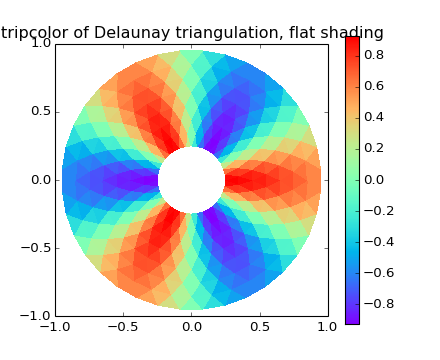(png, hires.png, pdf)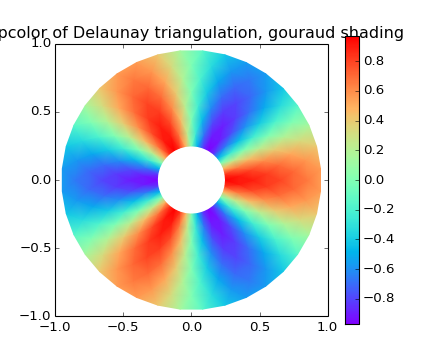(png, hires.png, pdf)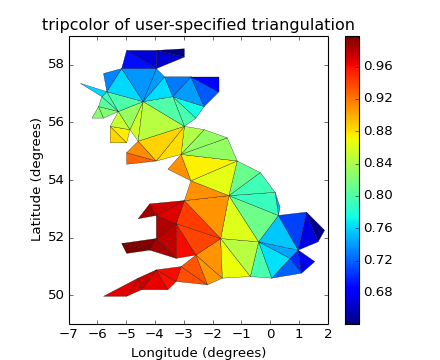(png, hires.png, pdf)

Additional kwargs: hold = [True|False] overrides default hold state

matplotlib.pyplot.triplot(*args, **kwargs)

Draw a unstructured triangular grid as lines and/or markers.

The triangulation to plot can be specified in one of two ways; either:

```triplot(triangulation, ...)
```

where triangulation is a matplotlib.tri.Triangulation object, or

```triplot(x, y, ...)
triplot(x, y, triangles, ...)
triplot(x, y, triangles=triangles, ...)
```

in which case a Triangulation object will be created. See Triangulation for a explanation of these possibilities.

The remaining args and kwargs are the same as for plot().

Example: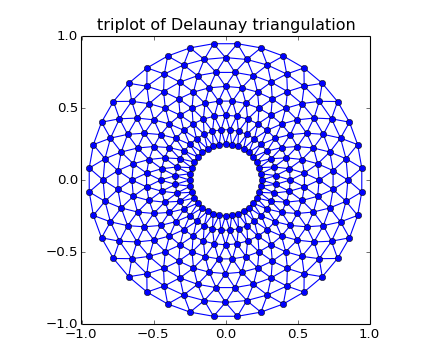(png, hires.png, pdf)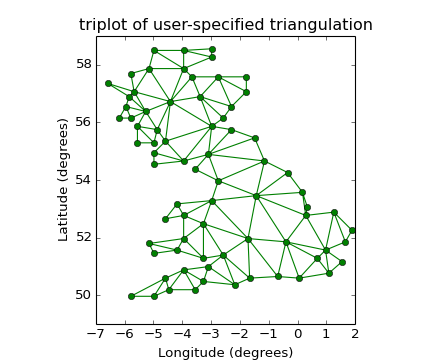(png, hires.png, pdf)

Additional kwargs: hold = [True|False] overrides default hold state

matplotlib.pyplot.twinx(ax=None)

Make a second axes that shares the x-axis. The new axes will overlay ax (or the current axes if ax is None). The ticks for ax2 will be placed on the right, and the ax2 instance is returned.

examples/api_examples/two_scales.py
For an example
matplotlib.pyplot.twiny(ax=None)

Make a second axes that shares the y-axis. The new axis will overlay ax (or the current axes if ax is None). The ticks for ax2 will be placed on the top, and the ax2 instance is returned.

matplotlib.pyplot.vlines(x, ymin, ymax, colors='k', linestyles='solid', label='', hold=None, **kwargs)

Plot vertical lines.

Plot vertical lines at each x from ymin to ymax.

Parameters: Returns: x : scalar or 1D array_like x-indexes where to plot the lines. xmin, xmax : scalar or 1D array_like Respective beginning and end of each line. If scalars are provided, all lines will have same length. colors : array_like of colors, optional, default: ‘k’ linestyles : [‘solid’ | ‘dashed’ | ‘dashdot’ | ‘dotted’], optional label : string, optional, default: ‘’ lines : LineCollection kwargs : LineCollection properties.

hlines
horizontal lines

ExamplesAdditional kwargs: hold = [True|False] overrides default hold state

matplotlib.pyplot.waitforbuttonpress(*args, **kwargs)

Call signature:

```waitforbuttonpress(self, timeout=-1)
```

Blocking call to interact with the figure.

This will return True is a key was pressed, False if a mouse button was pressed and None if timeout was reached without either being pressed.

If timeout is negative, does not timeout.

matplotlib.pyplot.winter()

set the default colormap to winter and apply to current image if any. See help(colormaps) for more information

matplotlib.pyplot.xcorr(x, y, normed=True, detrend=<function detrend_none at 0x2635de8>, usevlines=True, maxlags=10, hold=None, **kwargs)

Plot the cross correlation between x and y.

Call signature:

```xcorr(self, x, y, normed=True, detrend=mlab.detrend_none,
usevlines=True, maxlags=10, **kwargs)
```

If normed = True, normalize the data by the cross correlation at 0-th lag. x and y are detrended by the detrend callable (default no normalization). x and y must be equal length.

Data are plotted as plot(lags, c, **kwargs)

Return value is a tuple (lags, c, line) where:

• lags are a length 2*maxlags+1 lag vector

• c is the 2*maxlags+1 auto correlation vector

• line is a Line2D instance

returned by plot().

The default linestyle is None and the default marker is ‘o’, though these can be overridden with keyword args. The cross correlation is performed with numpy.correlate() with mode = 2.

If usevlines is True:

vlines() rather than plot() is used to draw vertical lines from the origin to the xcorr. Otherwise the plotstyle is determined by the kwargs, which are Line2D properties.

The return value is a tuple (lags, c, linecol, b) where linecol is the matplotlib.collections.LineCollection instance and b is the x-axis.

maxlags is a positive integer detailing the number of lags to show. The default value of None will return all (2*len(x)-1) lags.

Example:

xcorr() is top graph, and acorr() is bottom graph.Additional kwargs: hold = [True|False] overrides default hold state

matplotlib.pyplot.xkcd(scale=1, length=100, randomness=2)

Turns on xkcd sketch-style drawing mode. This will only have effect on things drawn after this function is called.

For best results, the “Humor Sans” font should be installed: it is not included with matplotlib.

Parameters: scale: float, optional : The amplitude of the wiggle perpendicular to the source line. length: float, optional : The length of the wiggle along the line. randomness: float, optional : The scale factor by which the length is shrunken or expanded. This function works by a number of rcParams, so it will probably : override others you have set before. : If you want the effects of this function to be temporary, it can : be used as a context manager, for example:: : with plt.xkcd(): # This figure will be in XKCD-style fig1 = plt.figure() # ... # This figure will be in regular style fig2 = plt.figure()
matplotlib.pyplot.xlabel(s, *args, **kwargs)

Set the x axis label of the current axis.

Default override is:

```override = {
'fontsize'            : 'small',
'verticalalignment'   : 'top',
'horizontalalignment' : 'center'
}
```

text()
For information on how override and the optional args work
matplotlib.pyplot.xlim(*args, **kwargs)

Get or set the x limits of the current axes.

```xmin, xmax = xlim()   # return the current xlim
xlim( (xmin, xmax) )  # set the xlim to xmin, xmax
xlim( xmin, xmax )    # set the xlim to xmin, xmax
```

If you do not specify args, you can pass the xmin and xmax as kwargs, e.g.:

```xlim(xmax=3) # adjust the max leaving min unchanged
xlim(xmin=1) # adjust the min leaving max unchanged
```

Setting limits turns autoscaling off for the x-axis.

The new axis limits are returned as a length 2 tuple.

matplotlib.pyplot.xscale(*args, **kwargs)

Set the scaling of the x-axis.

call signature:

```xscale(scale, **kwargs)
```

The available scales are: ‘linear’ | ‘log’ | ‘symlog’

Different keywords may be accepted, depending on the scale:

‘linear’

‘log’

basex/basey:
The base of the logarithm
non-positive values in x or y can be masked as invalid, or clipped to a very small positive number
subsx/subsy:

Where to place the subticks between each major tick. Should be a sequence of integers. For example, in a log10 scale: [2, 3, 4, 5, 6, 7, 8, 9]

will place 8 logarithmically spaced minor ticks between each major tick.

‘symlog’

basex/basey:
The base of the logarithm
linthreshx/linthreshy:
The range (-x, x) within which the plot is linear (to avoid having the plot go to infinity around zero).
subsx/subsy:

Where to place the subticks between each major tick. Should be a sequence of integers. For example, in a log10 scale: [2, 3, 4, 5, 6, 7, 8, 9]

will place 8 logarithmically spaced minor ticks between each major tick.

linscalex/linscaley:
This allows the linear range (-linthresh to linthresh) to be stretched relative to the logarithmic range. Its value is the number of decades to use for each half of the linear range. For example, when linscale == 1.0 (the default), the space used for the positive and negative halves of the linear range will be equal to one decade in the logarithmic range.
matplotlib.pyplot.xticks(*args, **kwargs)

Get or set the x-limits of the current tick locations and labels.

```# return locs, labels where locs is an array of tick locations and
# labels is an array of tick labels.
locs, labels = xticks()

# set the locations of the xticks
xticks( arange(6) )

# set the locations and labels of the xticks
xticks( arange(5), ('Tom', 'Dick', 'Harry', 'Sally', 'Sue') )
```

The keyword args, if any, are Text properties. For example, to rotate long labels:

```xticks( arange(12), calendar.month_name[1:13], rotation=17 )
```
matplotlib.pyplot.ylabel(s, *args, **kwargs)

Set the y axis label of the current axis.

Defaults override is:

```override = {
'fontsize'            : 'small',
'verticalalignment'   : 'center',
'horizontalalignment' : 'right',
'rotation'='vertical' : }```

text()
For information on how override and the optional args work.
matplotlib.pyplot.ylim(*args, **kwargs)

Get or set the y-limits of the current axes.

```ymin, ymax = ylim()   # return the current ylim
ylim( (ymin, ymax) )  # set the ylim to ymin, ymax
ylim( ymin, ymax )    # set the ylim to ymin, ymax
```

If you do not specify args, you can pass the ymin and ymax as kwargs, e.g.:

```ylim(ymax=3) # adjust the max leaving min unchanged
ylim(ymin=1) # adjust the min leaving max unchanged
```

Setting limits turns autoscaling off for the y-axis.

The new axis limits are returned as a length 2 tuple.

matplotlib.pyplot.yscale(*args, **kwargs)

Set the scaling of the y-axis.

call signature:

```yscale(scale, **kwargs)
```

The available scales are: ‘linear’ | ‘log’ | ‘symlog’

Different keywords may be accepted, depending on the scale:

‘linear’

‘log’

basex/basey:
The base of the logarithm
non-positive values in x or y can be masked as invalid, or clipped to a very small positive number
subsx/subsy:

Where to place the subticks between each major tick. Should be a sequence of integers. For example, in a log10 scale: [2, 3, 4, 5, 6, 7, 8, 9]

will place 8 logarithmically spaced minor ticks between each major tick.

‘symlog’

basex/basey:
The base of the logarithm
linthreshx/linthreshy:
The range (-x, x) within which the plot is linear (to avoid having the plot go to infinity around zero).
subsx/subsy:

Where to place the subticks between each major tick. Should be a sequence of integers. For example, in a log10 scale: [2, 3, 4, 5, 6, 7, 8, 9]

will place 8 logarithmically spaced minor ticks between each major tick.

linscalex/linscaley:
This allows the linear range (-linthresh to linthresh) to be stretched relative to the logarithmic range. Its value is the number of decades to use for each half of the linear range. For example, when linscale == 1.0 (the default), the space used for the positive and negative halves of the linear range will be equal to one decade in the logarithmic range.
matplotlib.pyplot.yticks(*args, **kwargs)

Get or set the y-limits of the current tick locations and labels.

```# return locs, labels where locs is an array of tick locations and
# labels is an array of tick labels.
locs, labels = yticks()

# set the locations of the yticks
yticks( arange(6) )

# set the locations and labels of the yticks
yticks( arange(5), ('Tom', 'Dick', 'Harry', 'Sally', 'Sue') )
```

The keyword args, if any, are Text properties. For example, to rotate long labels:

```yticks( arange(12), calendar.month_name[1:13], rotation=45 )
```